# 2 Package ggplot2

An elegant modern graphic method based on Wilkinson's Grammar of Graphics

## 2.1 Maps:

### 2.1.1 Polygon

library(ggplot2)
require(maps) 
## Loading required package: maps
portugal = map_data('world', region = 'Portugal')
ggplot(portugal, aes(x = long, y = lat, group = group)) +
geom_polygon(fill = 'white', colour = 'black')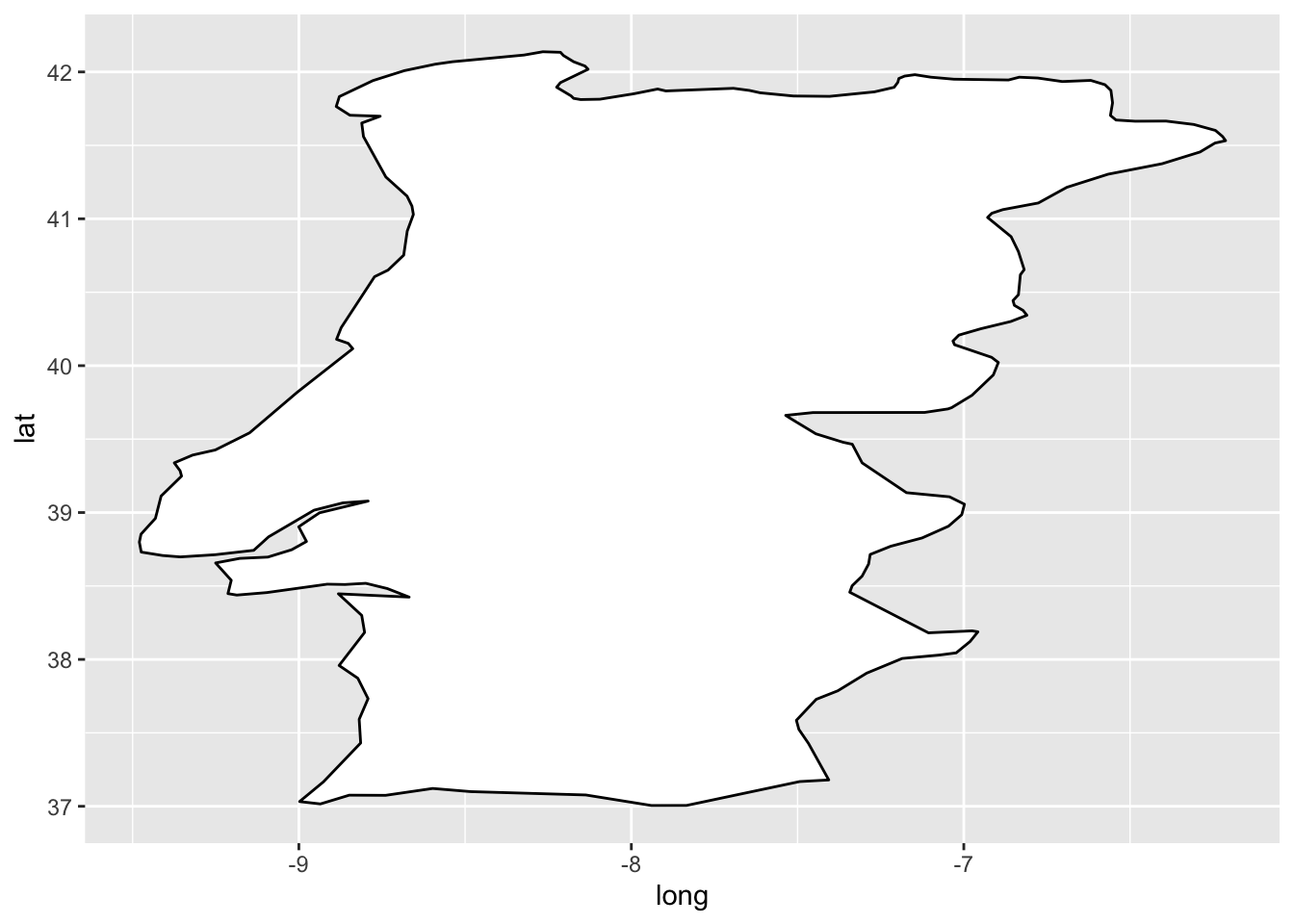## 2.2 Path, ribbon and rectangles.

h <- ggplot(economics, aes(date, unemploy))
# Path
h + geom_path()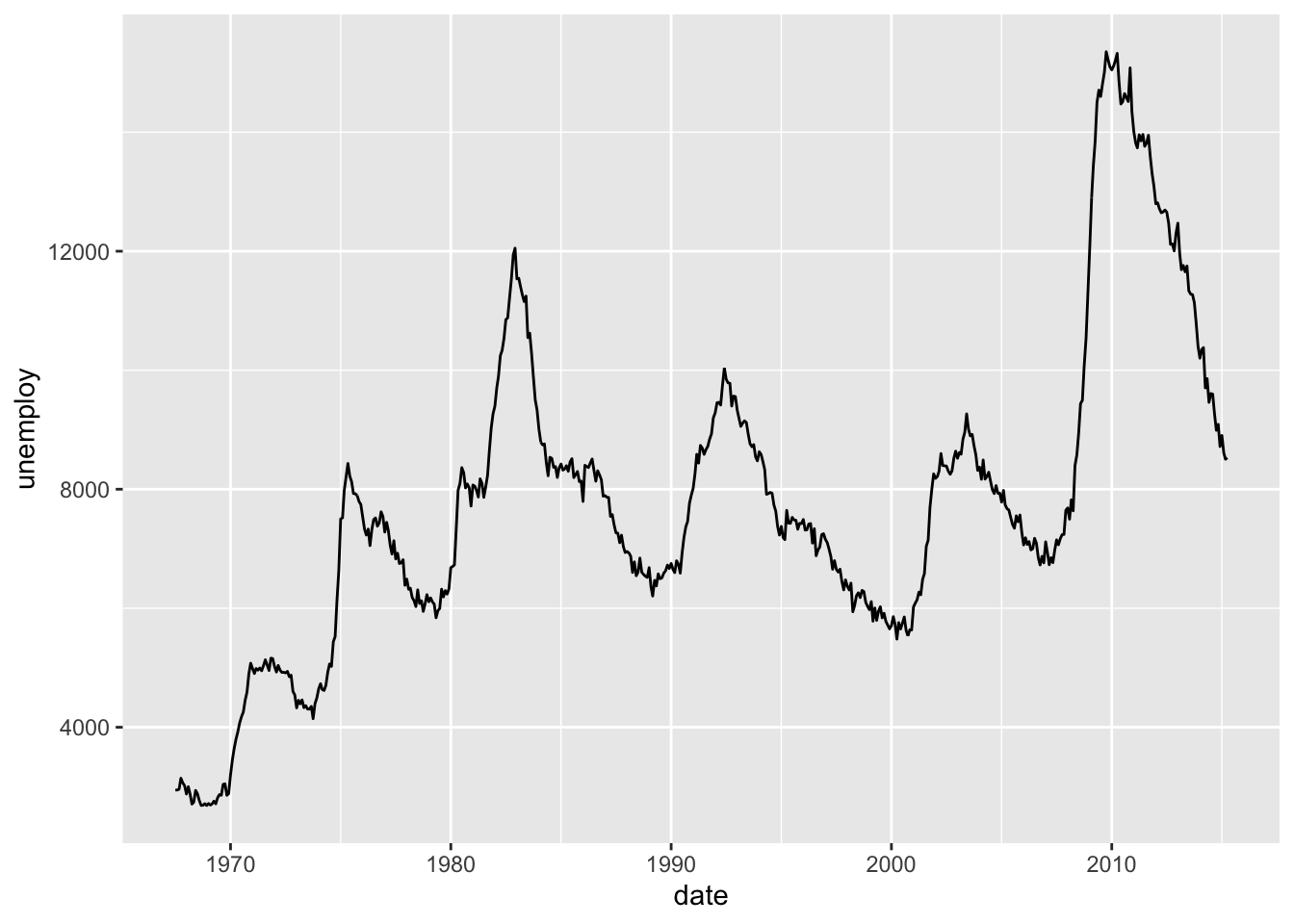# Ribbon
h + geom_ribbon(aes(ymin = unemploy-900, ymax = unemploy+900), fill = "steelblue") + geom_path(size = 0.8)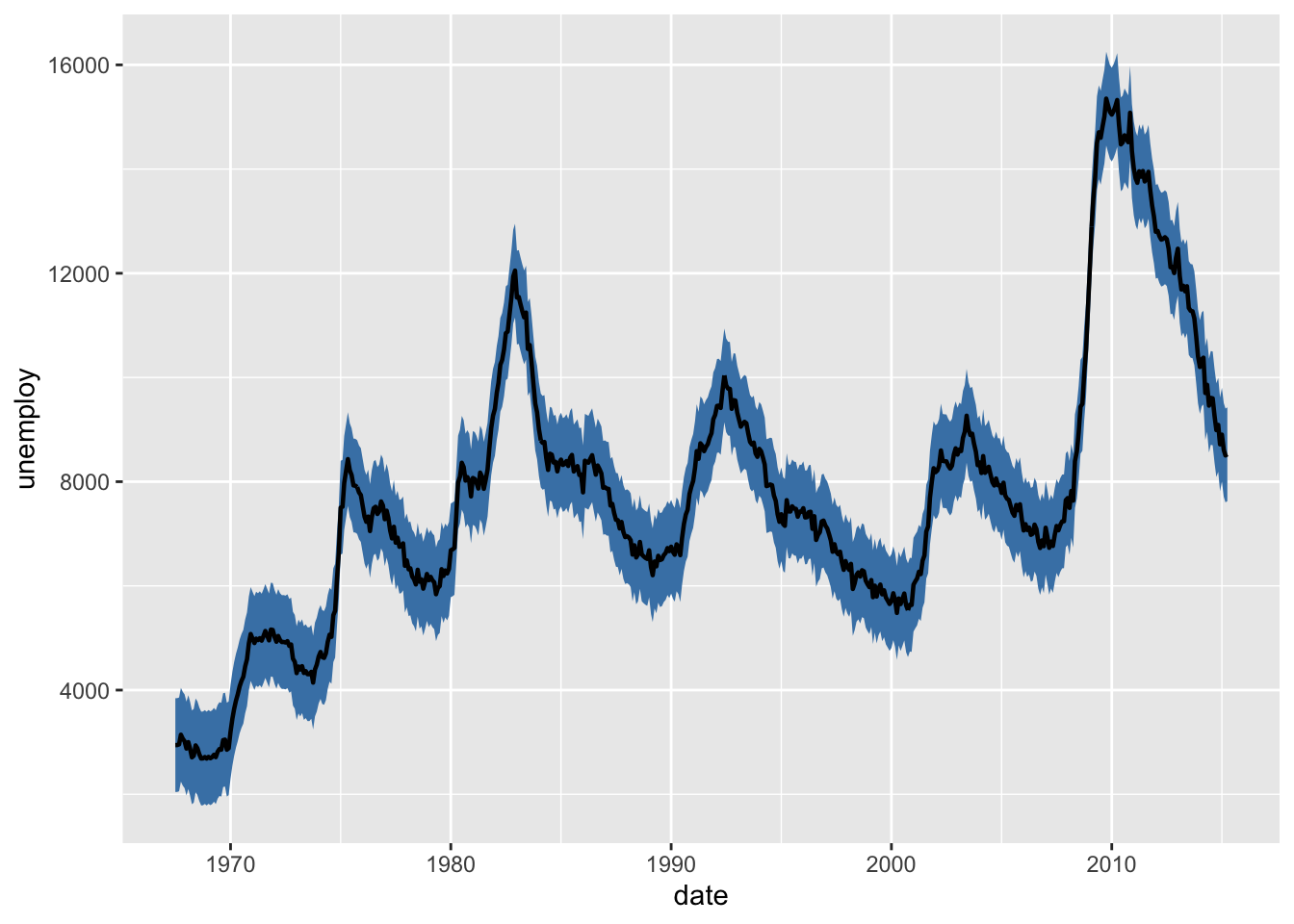# Rectangle
h + geom_rect(aes(xmin = as.Date('1980-01-01'), ymin = -Inf, xmax = as.Date('1985-01-01'), ymax = Inf), fill = "steelblue") + geom_path(size = 0.8)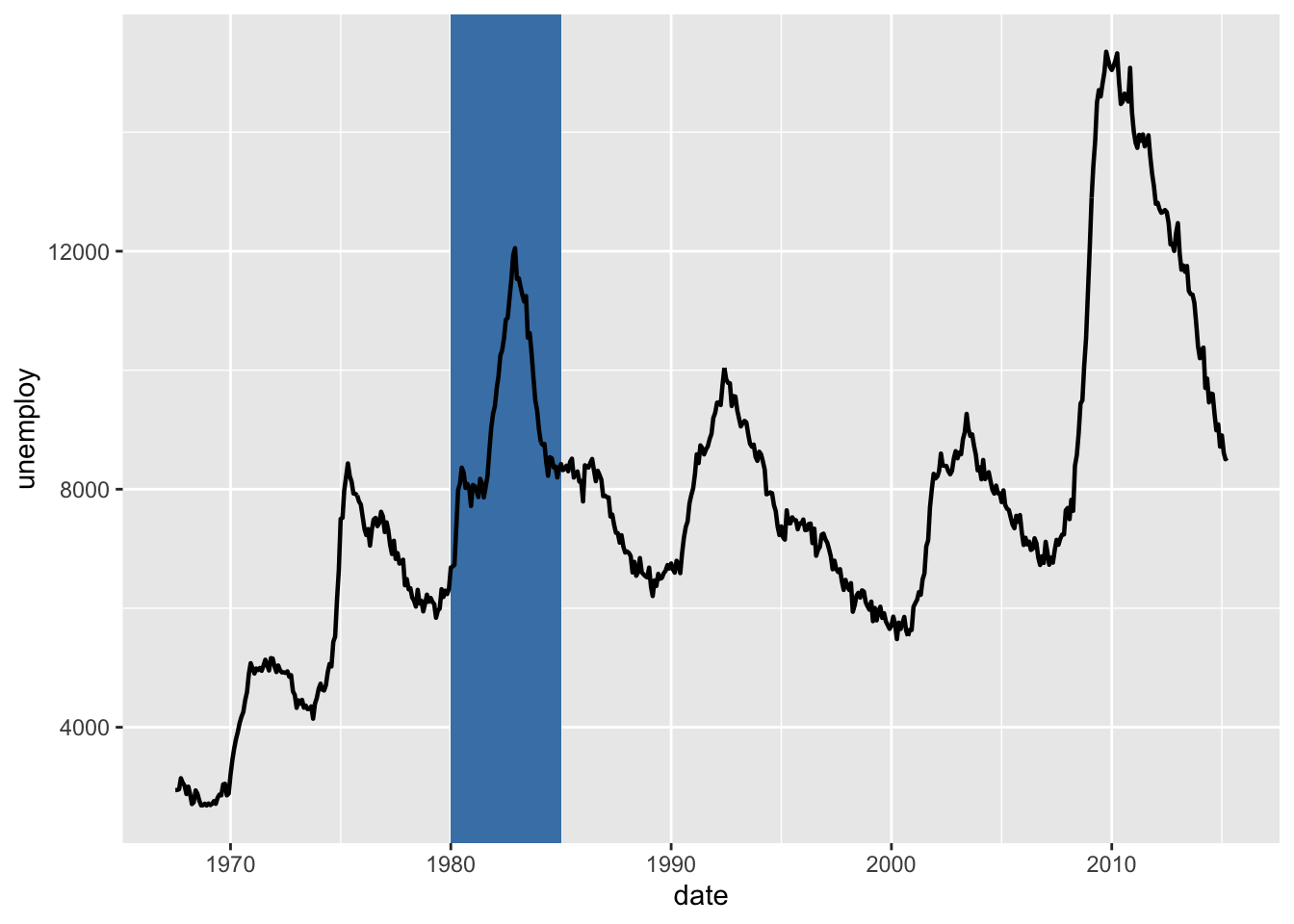### 2.2.1 Add line segments between points (x1, y1) and (x2, y2):

#### 2.2.1.1 Create a scatter plot

i <- ggplot(mtcars, aes(wt, mpg)) + geom_point()
# Add segment
i + geom_segment(aes(x = 2, y = 15, xend = 3, yend = 15))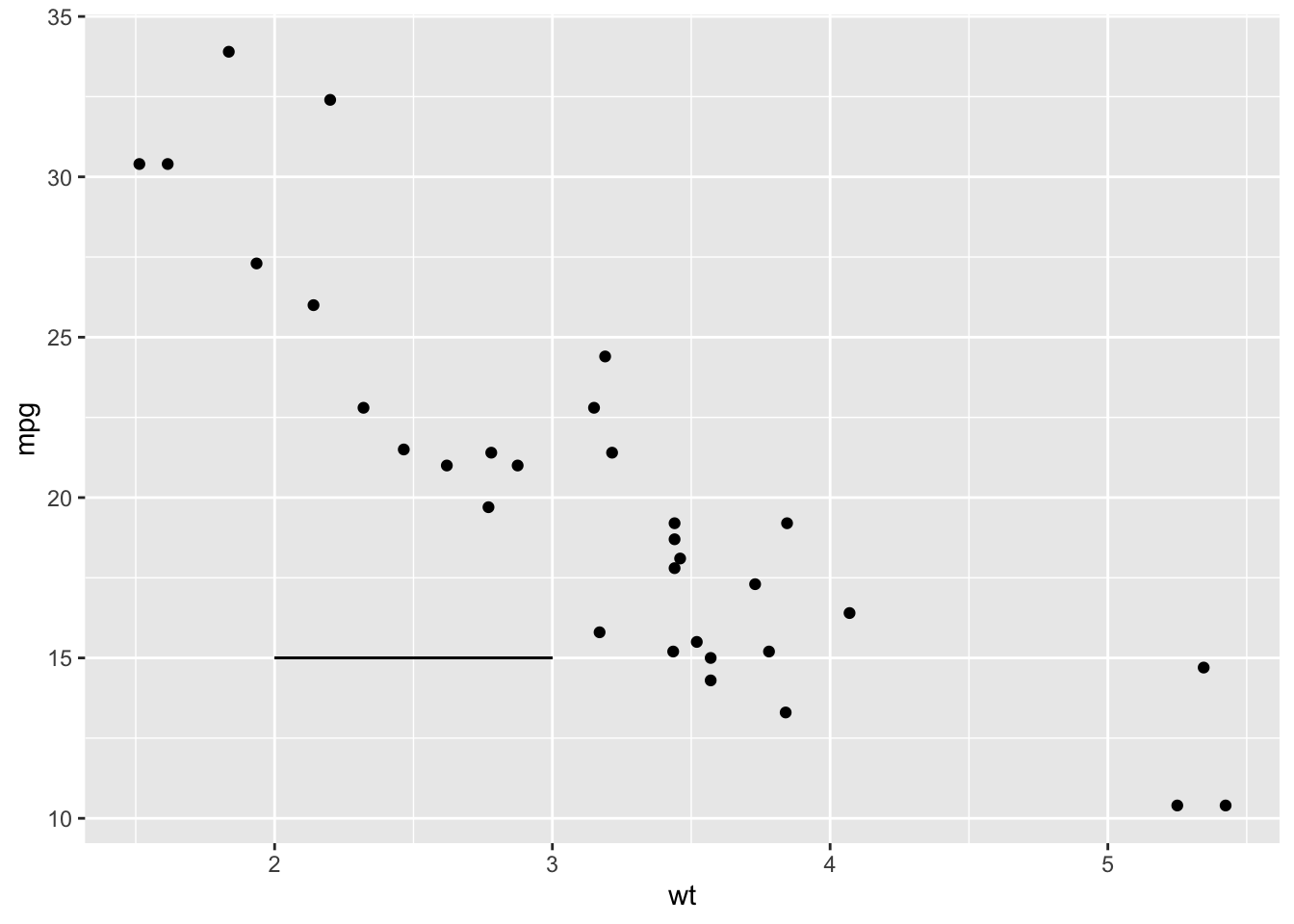# Add arrow require(grid)
i + geom_segment(aes(x = 5, y = 30, xend = 3.5, yend = 25), arrow = arrow(length = unit(0.5, "cm")))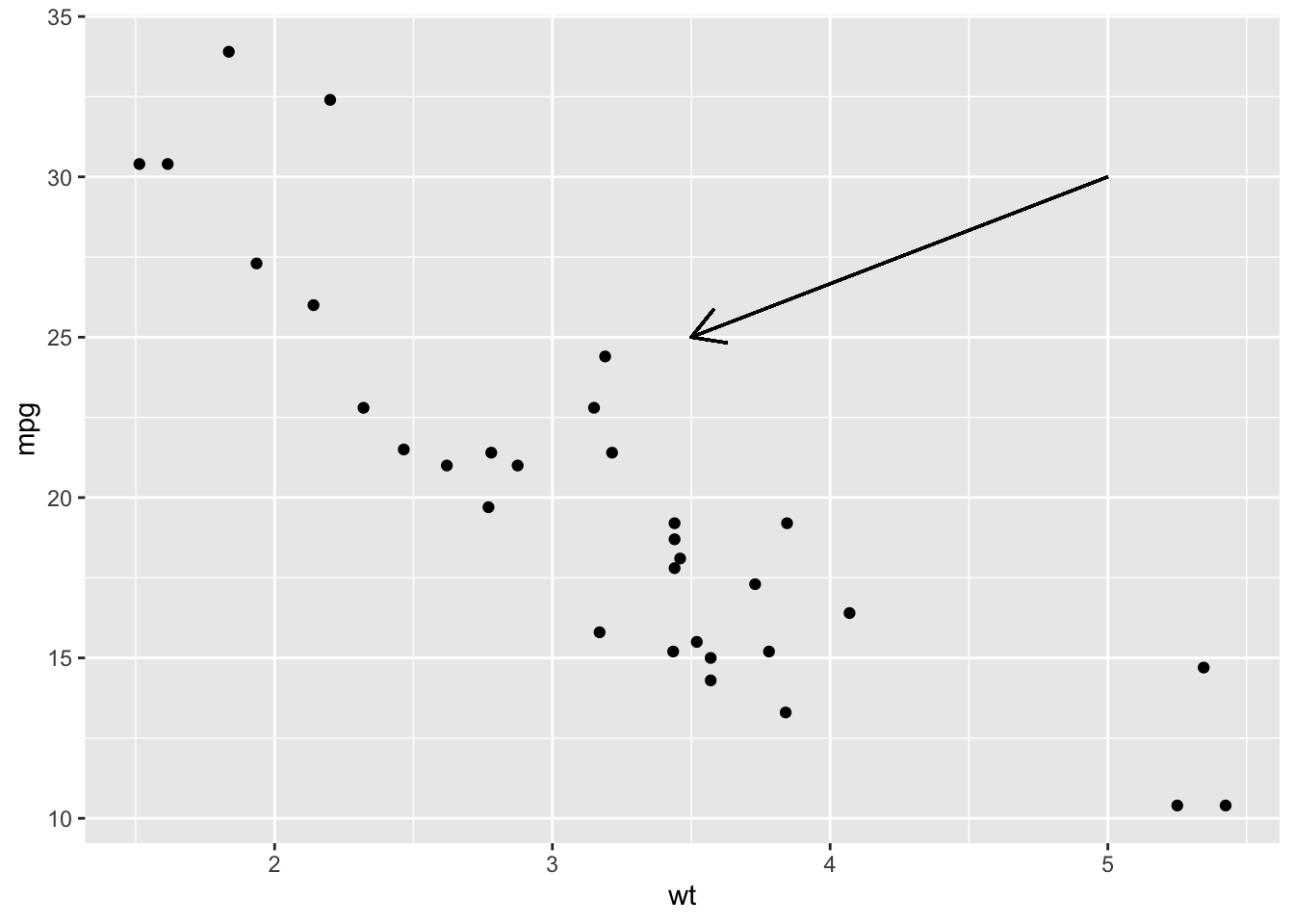#### 2.2.1.2 Add curves between points (x1, y1) and (x2, y2):

i + geom_curve(aes(x = 2, y = 15, xend = 3, yend = 15))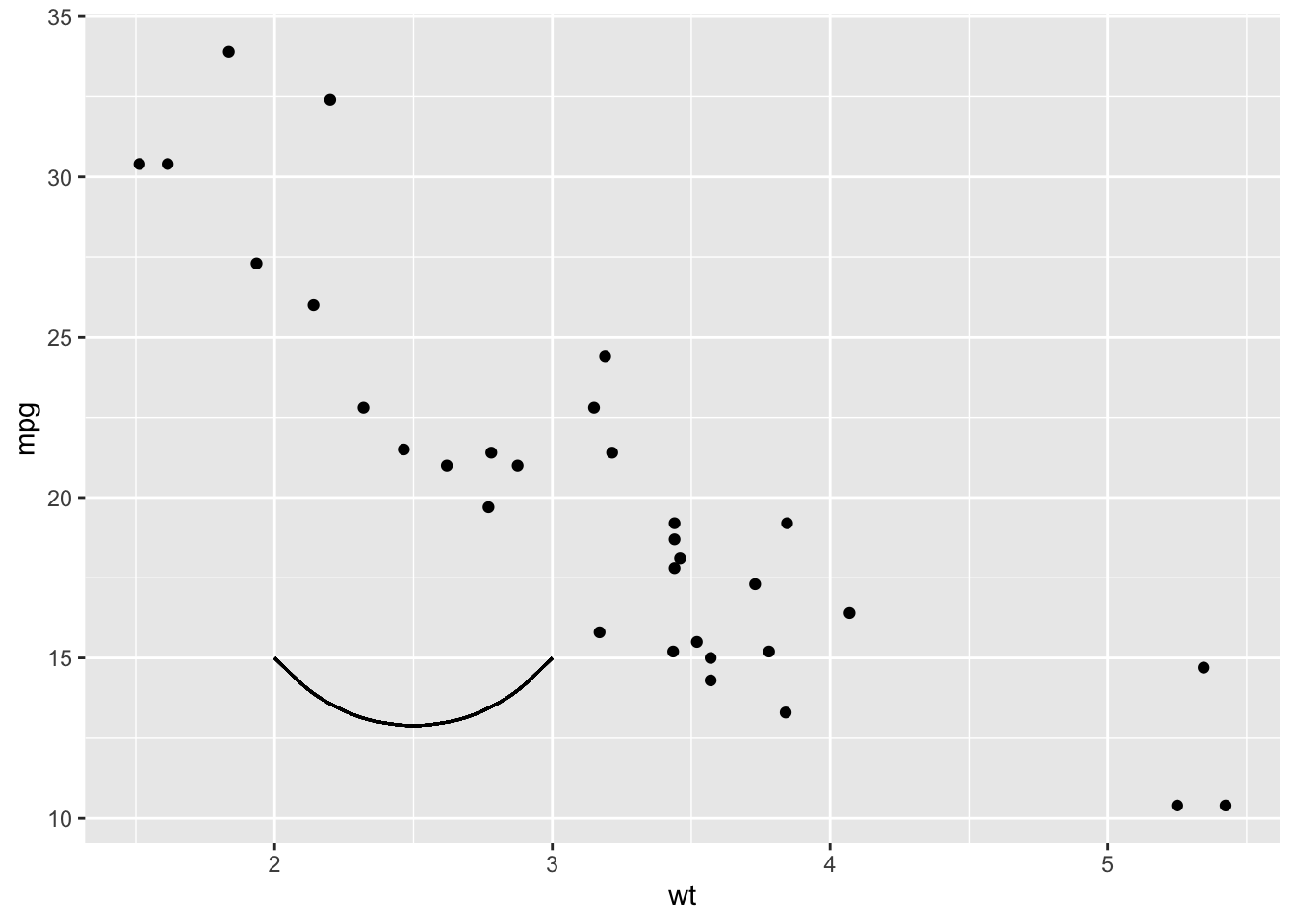## 2.3 Graphical parameters:

### 2.3.1 Convert the variable dose from numeric to factor variable

ToothGrowth$dose <- as.factor(ToothGrowth$dose)
p <- ggplot(ToothGrowth, aes(x=dose, y=len))
p+ geom_boxplot()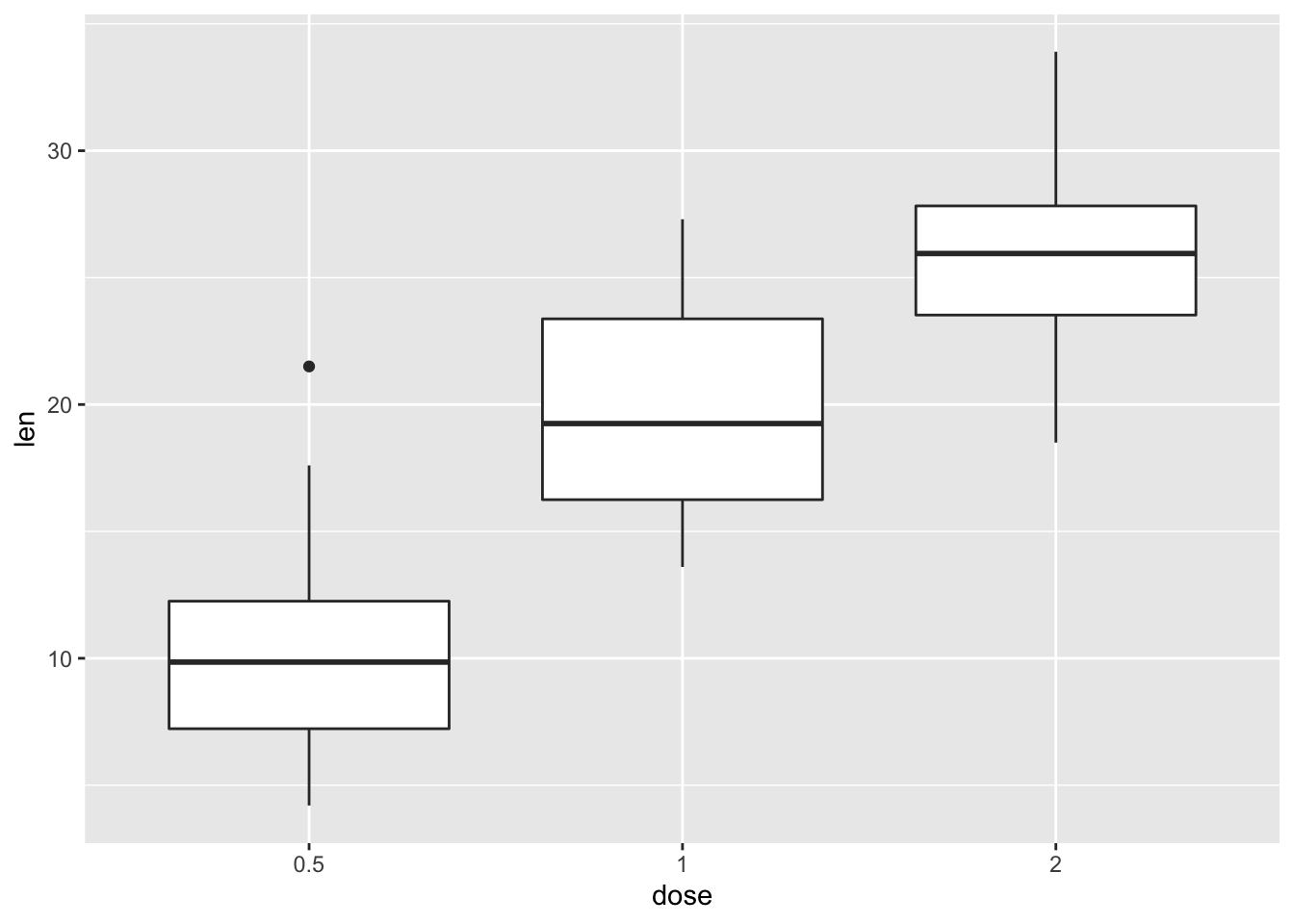### 2.3.2 Default plot

print(p)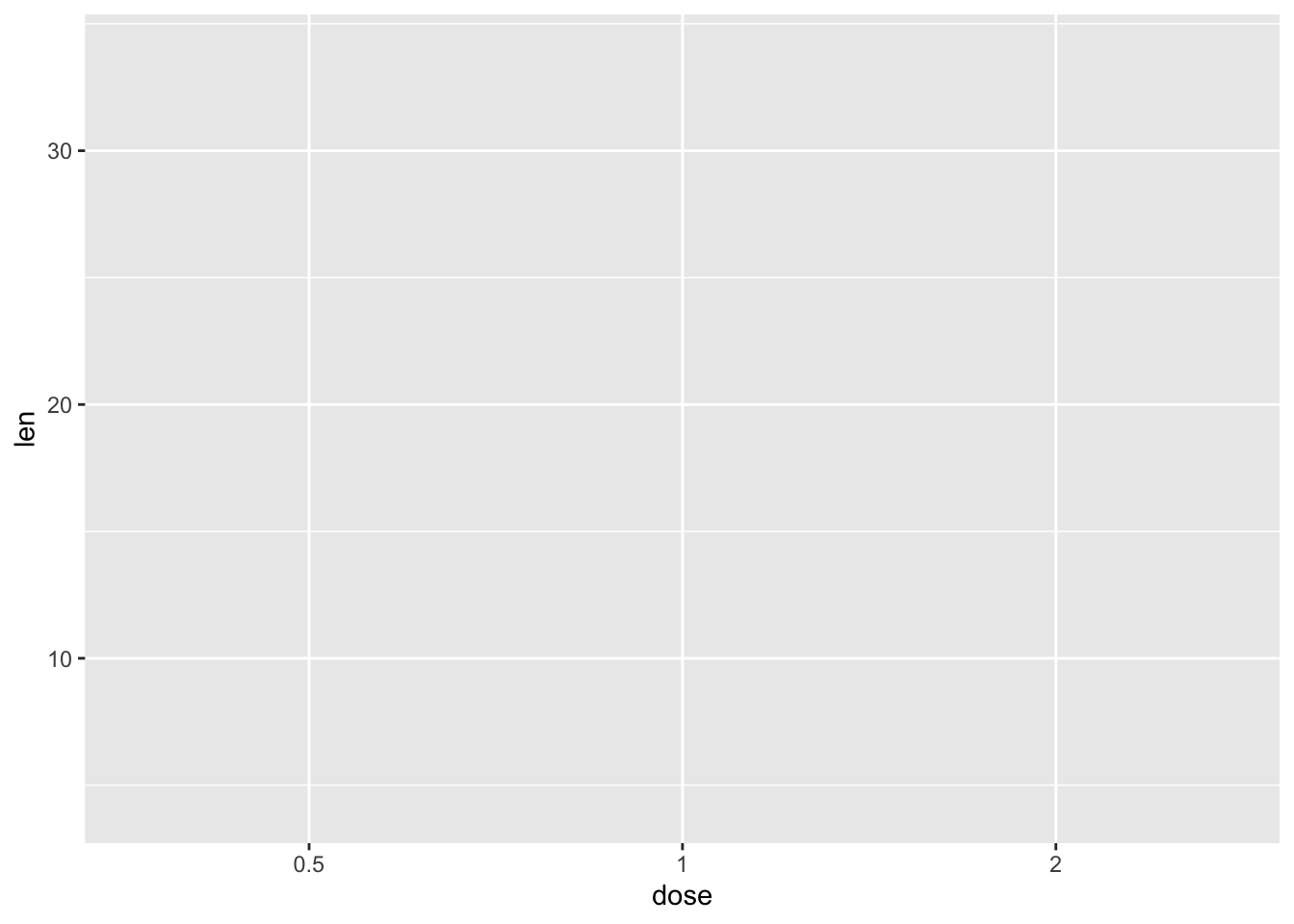### 2.3.3 Change title and axis labels

p1 <- p +labs(title="Plot of length \n by dose", x ="Dose (mg)", y = "Teeth length")
p1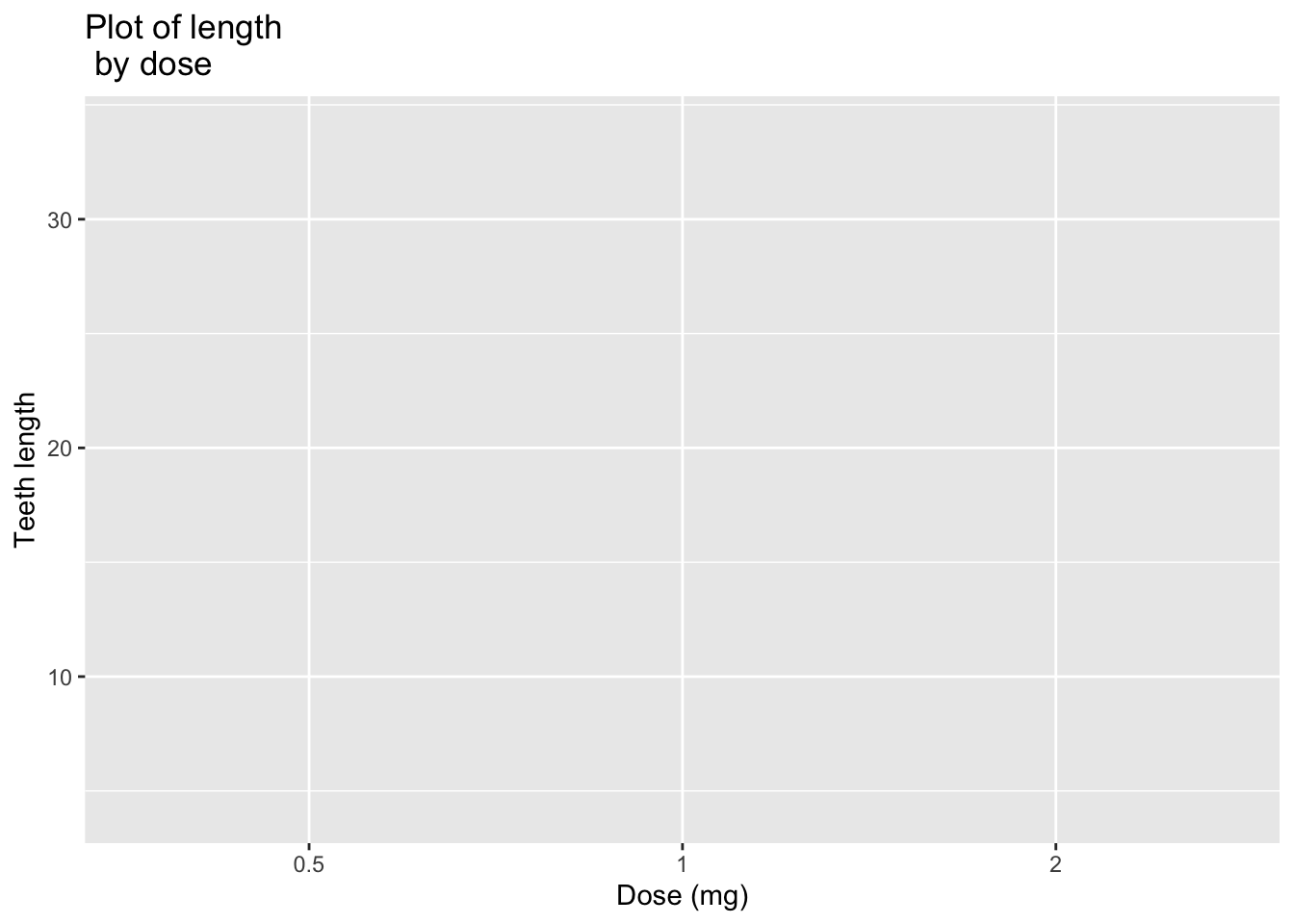### 2.3.4 Change the appearance of labels

p1 + theme( plot.title = element_text(color="violet", size=14, face="bold.italic"), axis.title.x = element_text(color="blue", size=14, face="bold"), axis.title.y = element_text(color="blue", size=14, face="bold") )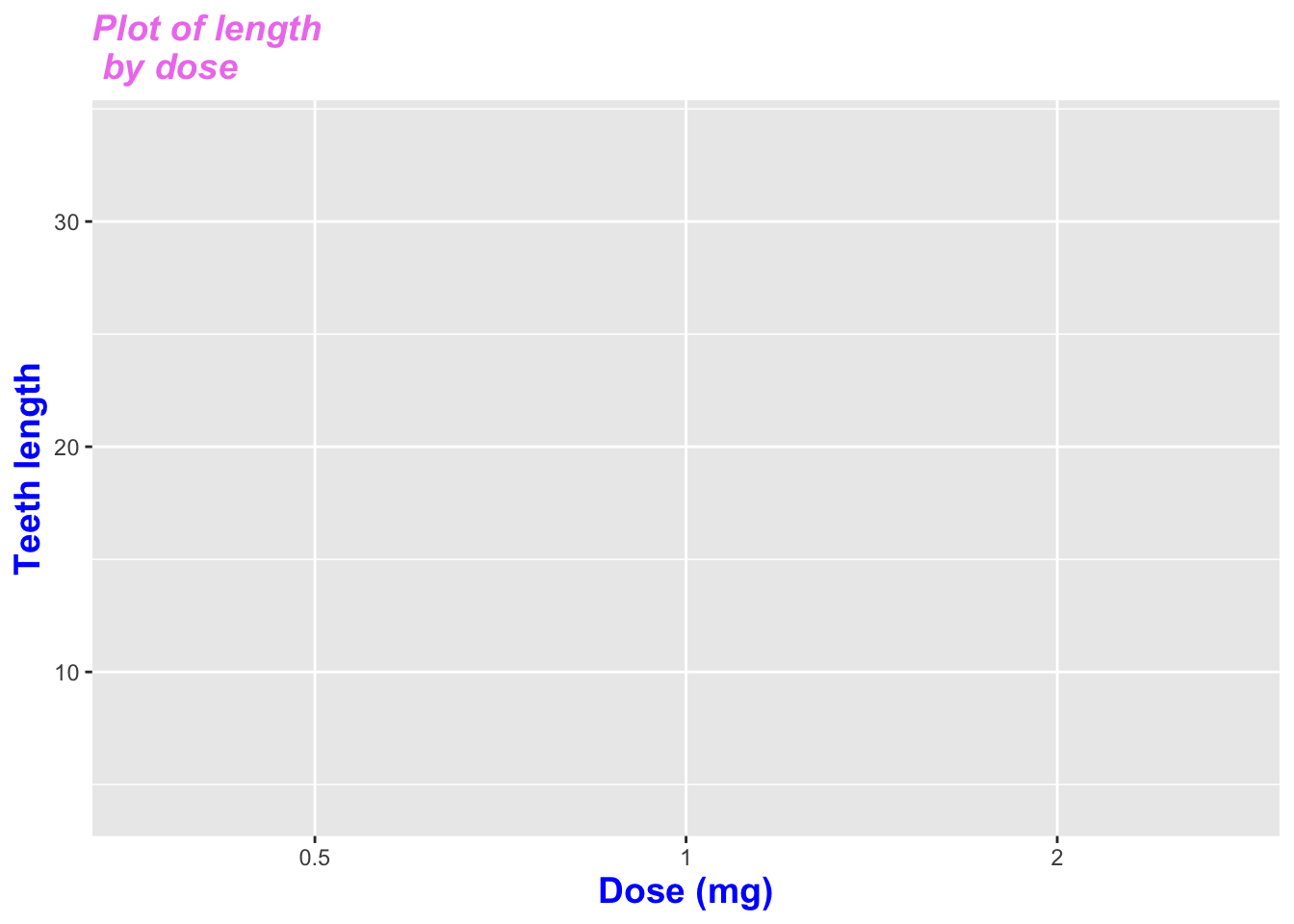# Hide labels
p1 + theme(plot.title = element_blank(), axis.title.x = element_blank(), axis.title.y = element_blank())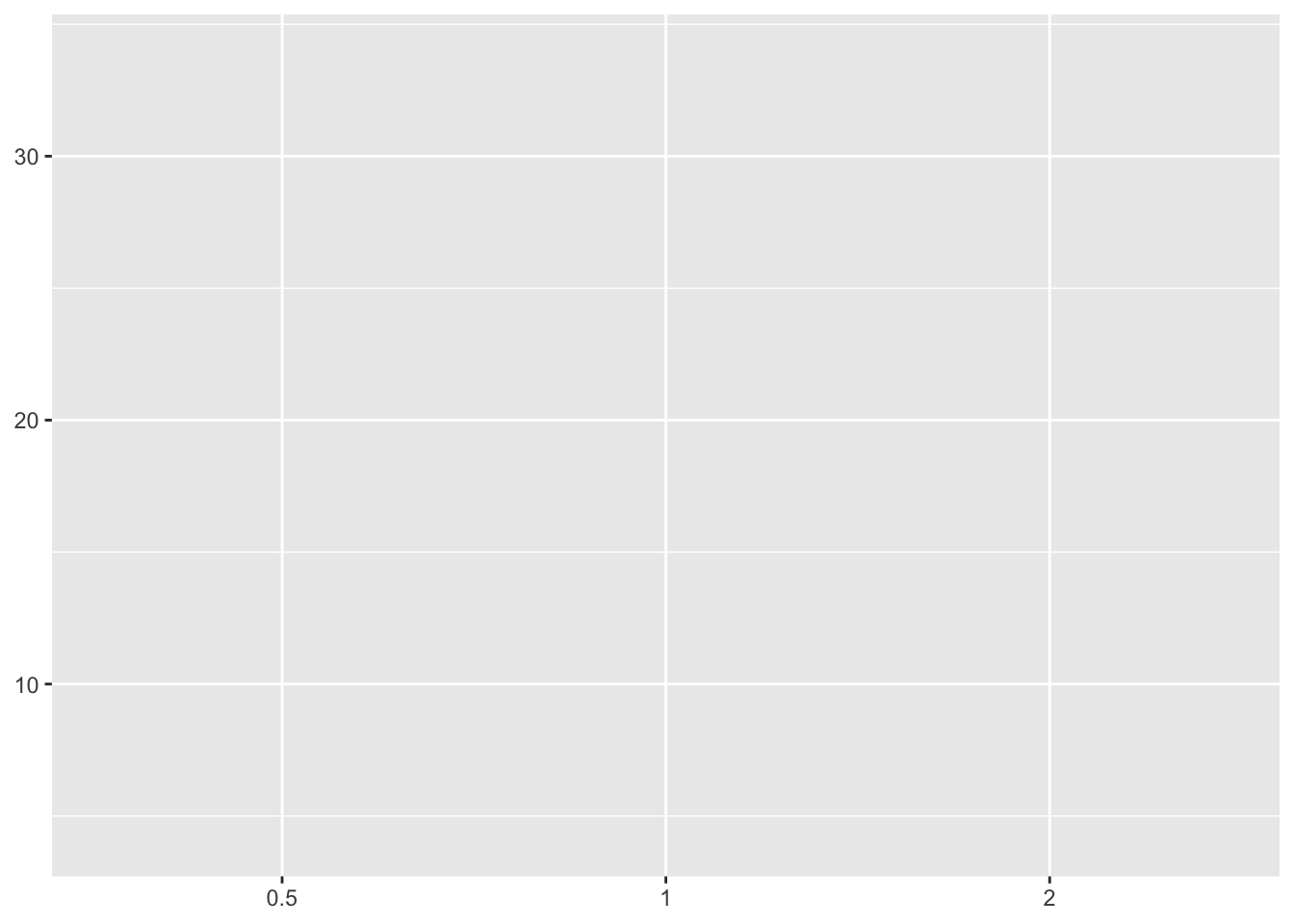### 2.3.5 Default plot

p <- ggplot(ToothGrowth, aes(x=dose, y=len, fill=dose))+ geom_boxplot()
p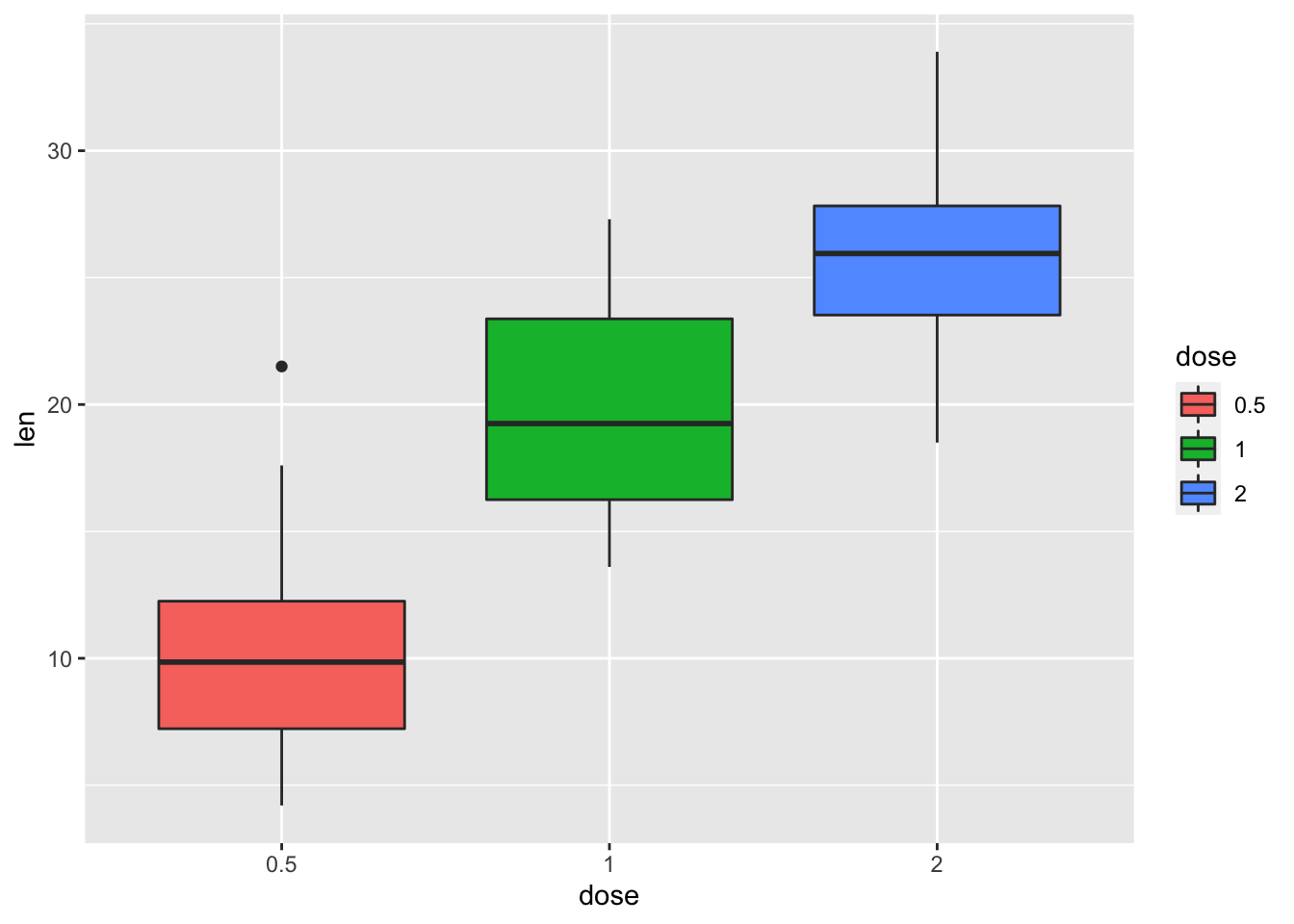# Modify legend titles
p + labs(fill = "Dose (mg)")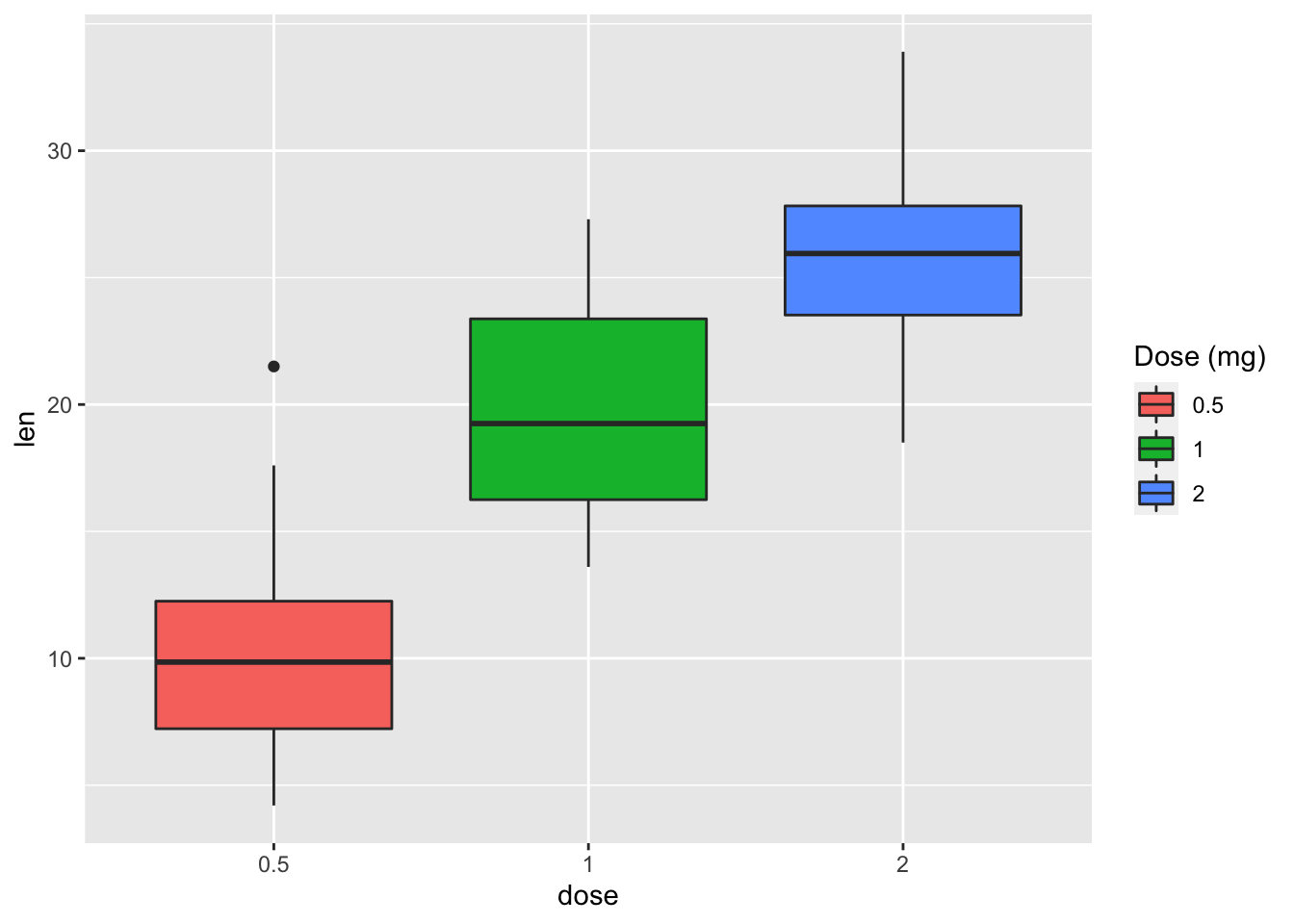## 2.4 Legend position and appearance

### 2.4.1 Convert the variable dose from numeric to factor variable

ToothGrowth$dose <- as.factor(ToothGrowth$dose)
p <- ggplot(ToothGrowth, aes(x=dose, y=len, fill=dose))+
geom_boxplot()

### 2.4.2 Change legend position: "left","top", "right", "bottom", "none"

p + theme(legend.position="top")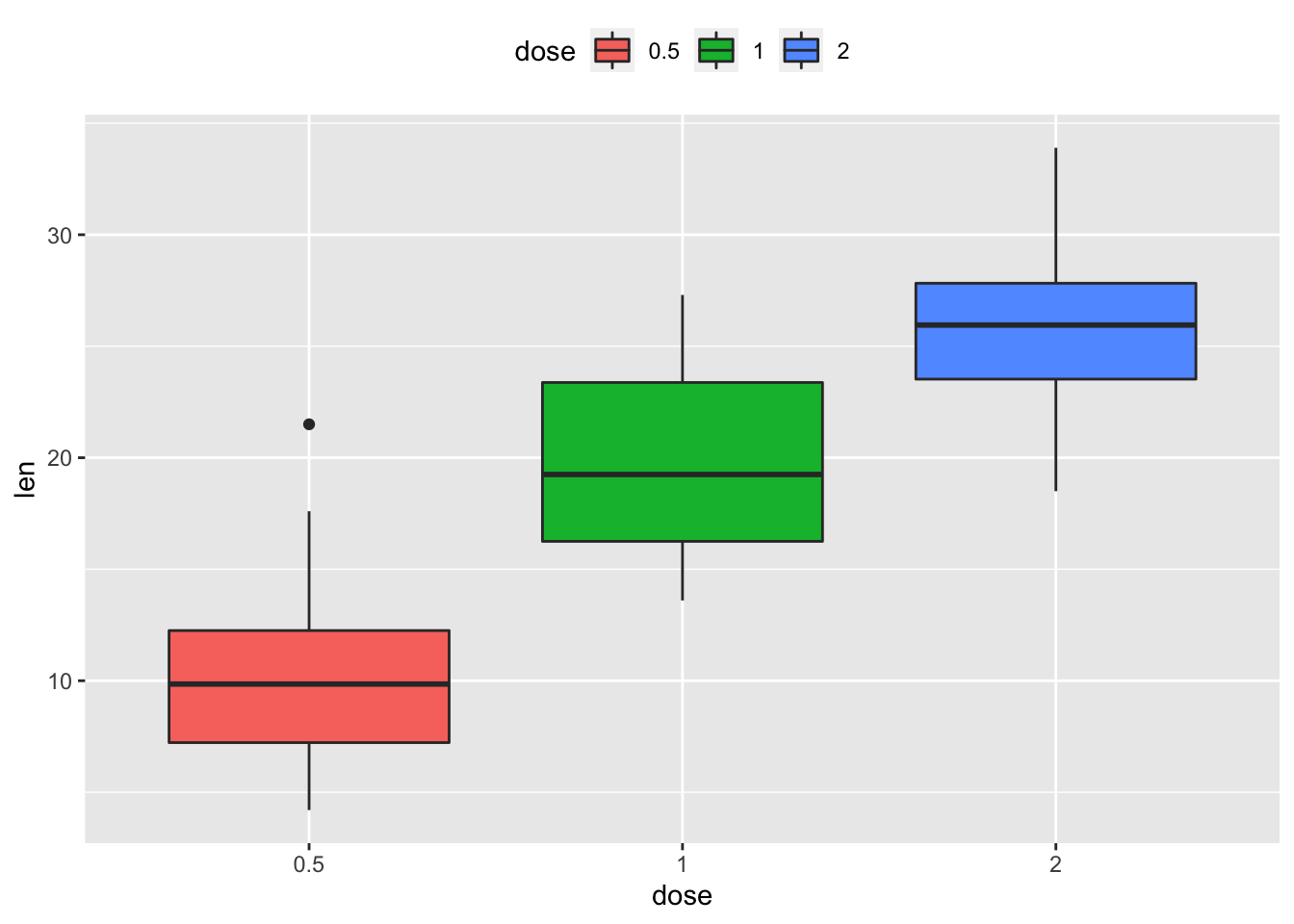### 2.4.3 Remove legends

p + theme(legend.position = "none")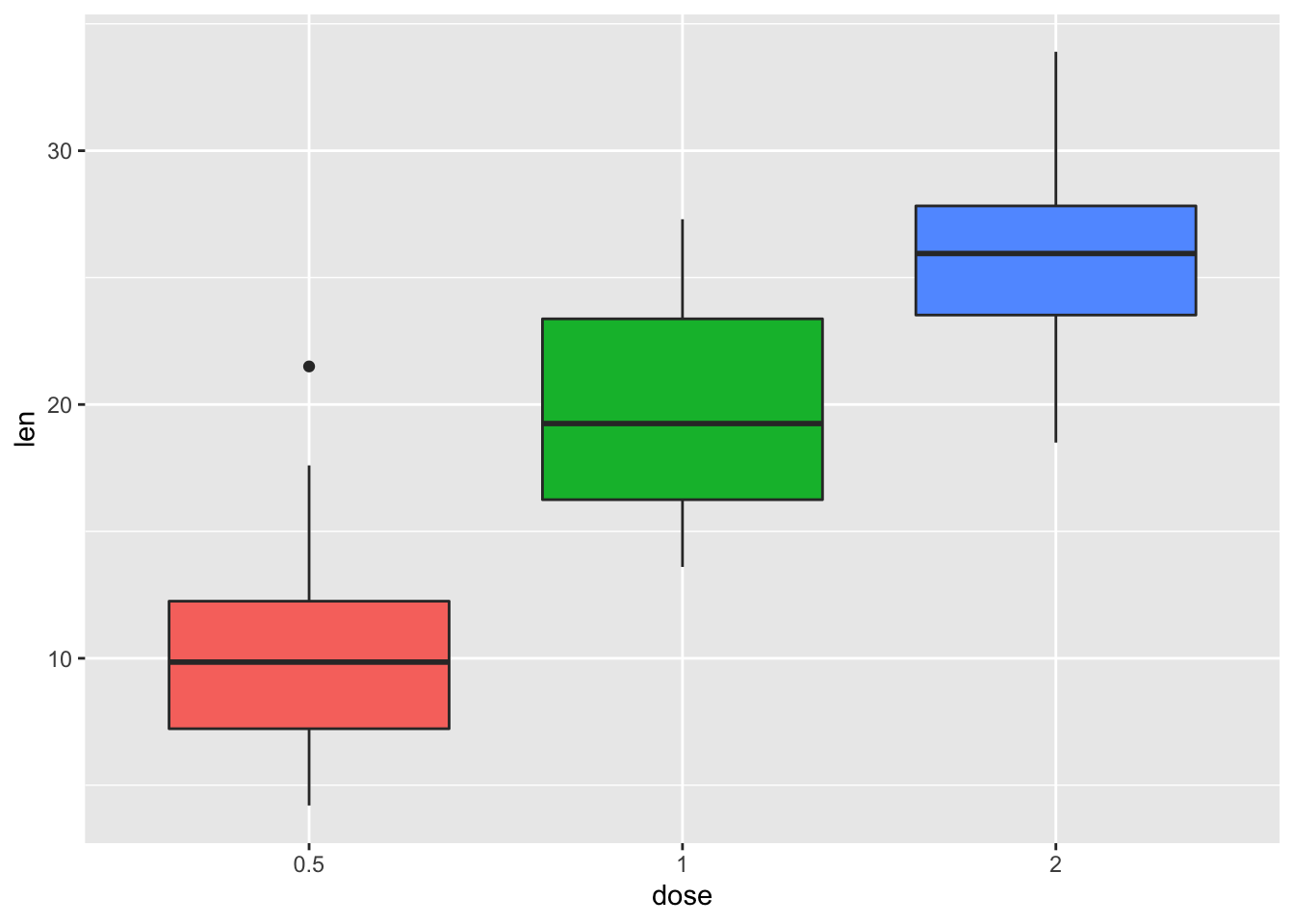# Change the appearance of legend title and labels
p + theme(legend.title = element_text(colour="darkgreen"), legend.text = element_text(colour="red"))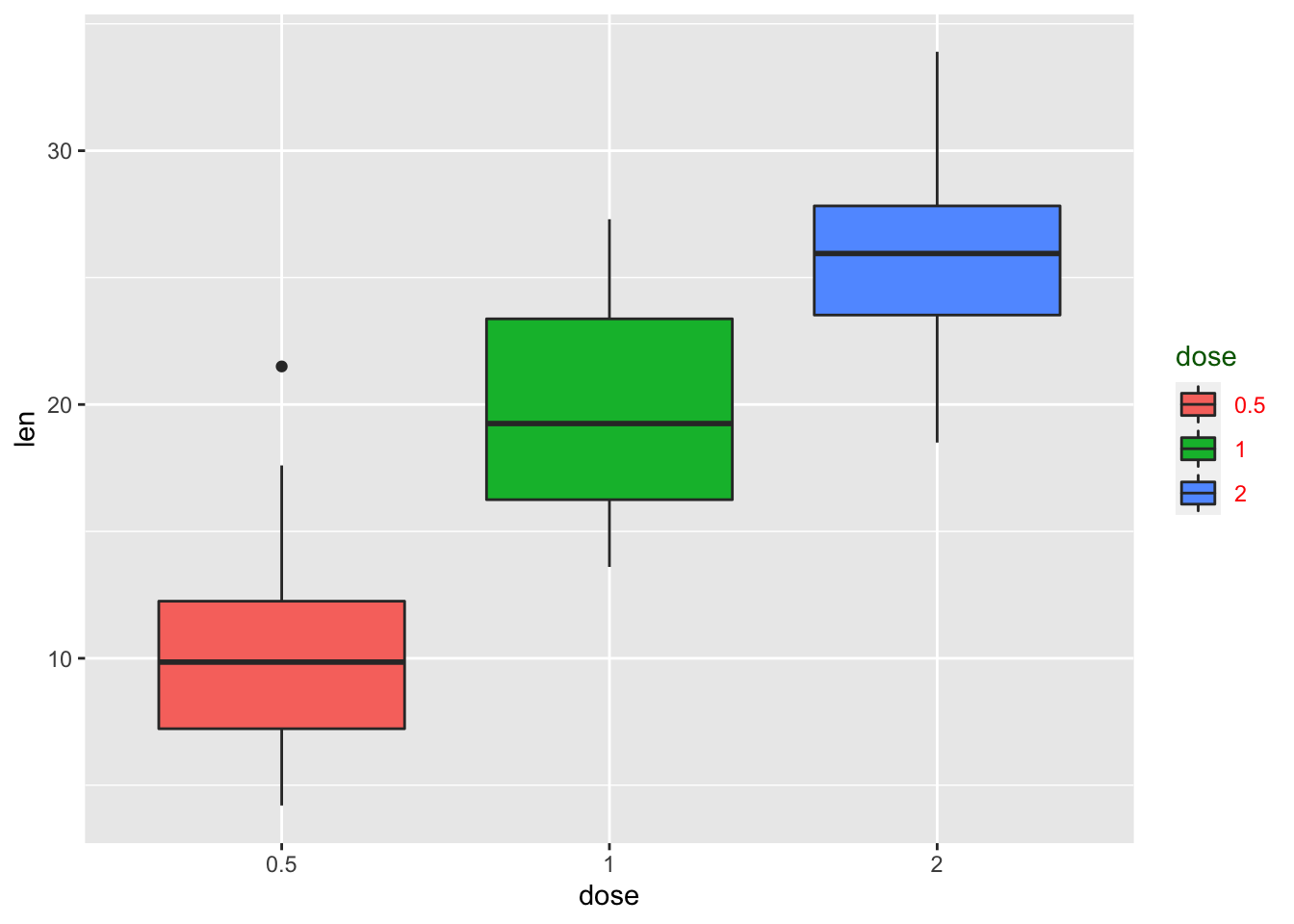# Change legend box background color
p + theme(legend.background = element_rect(fill="violet"))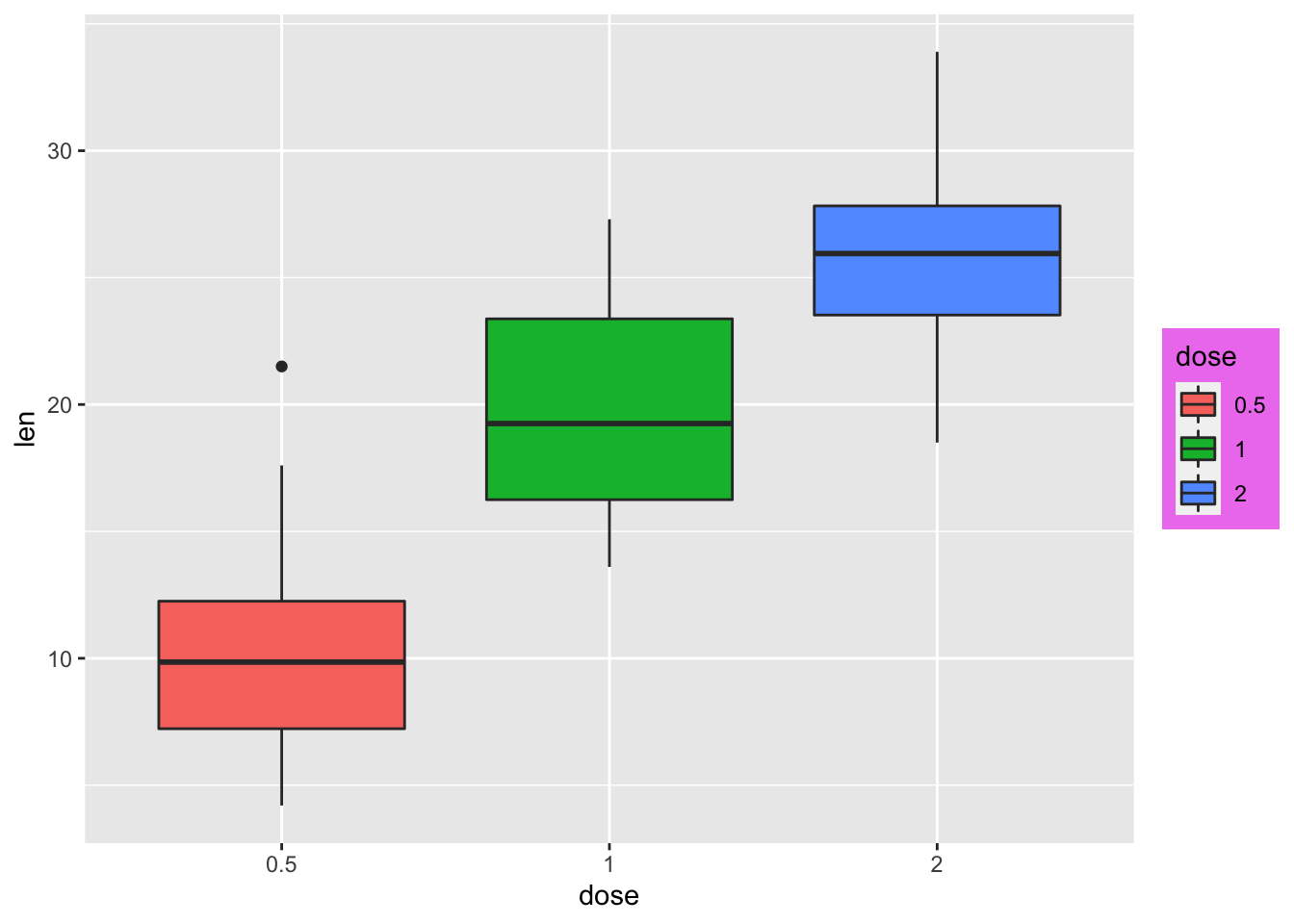### 2.4.4 Change the order of legend items

p + scale_x_discrete(limits=c("2", "0.5", "1"))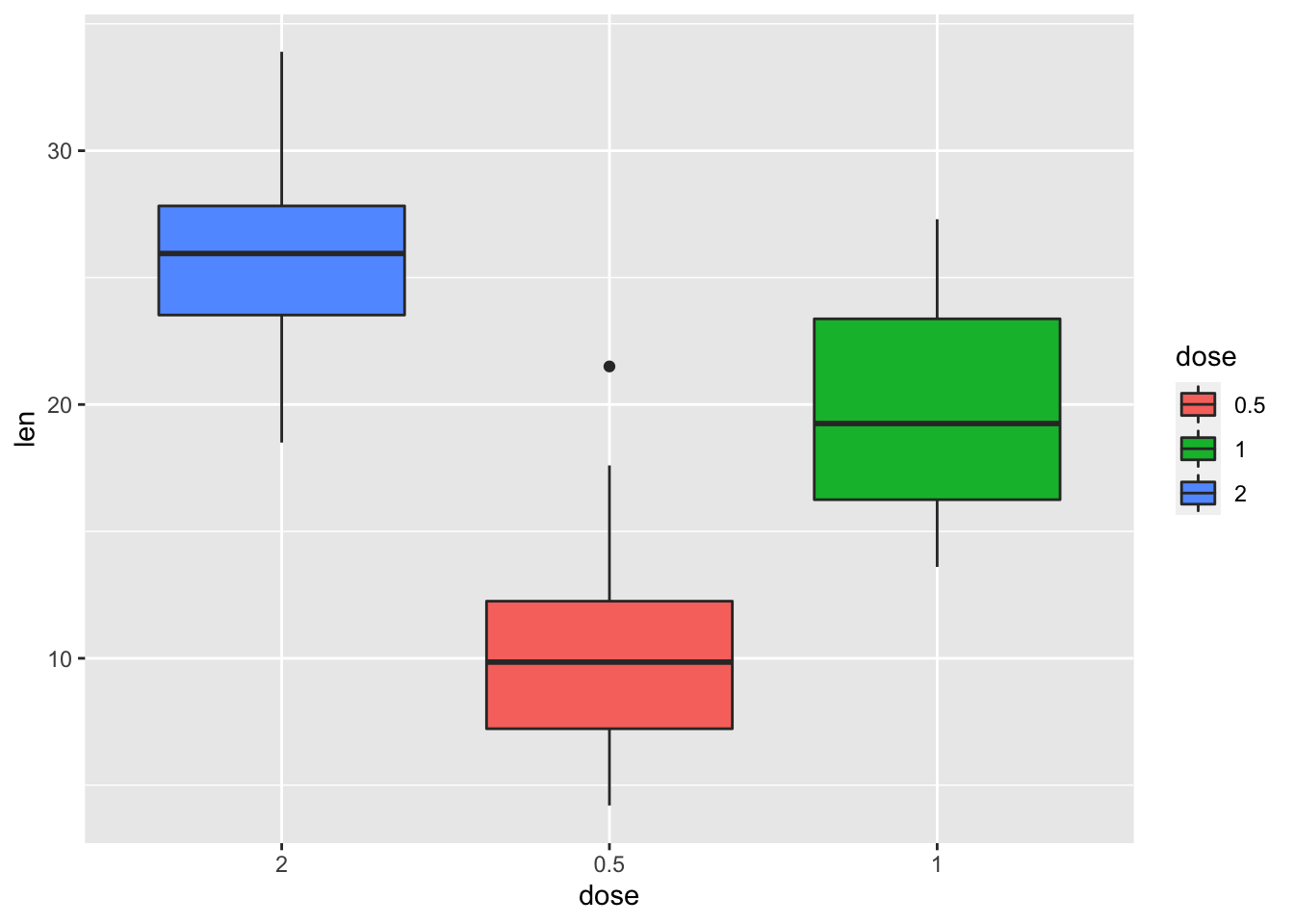# Set legend title and labels
p + scale_fill_discrete(name = "Dose", labels = c("A", "B", "C"))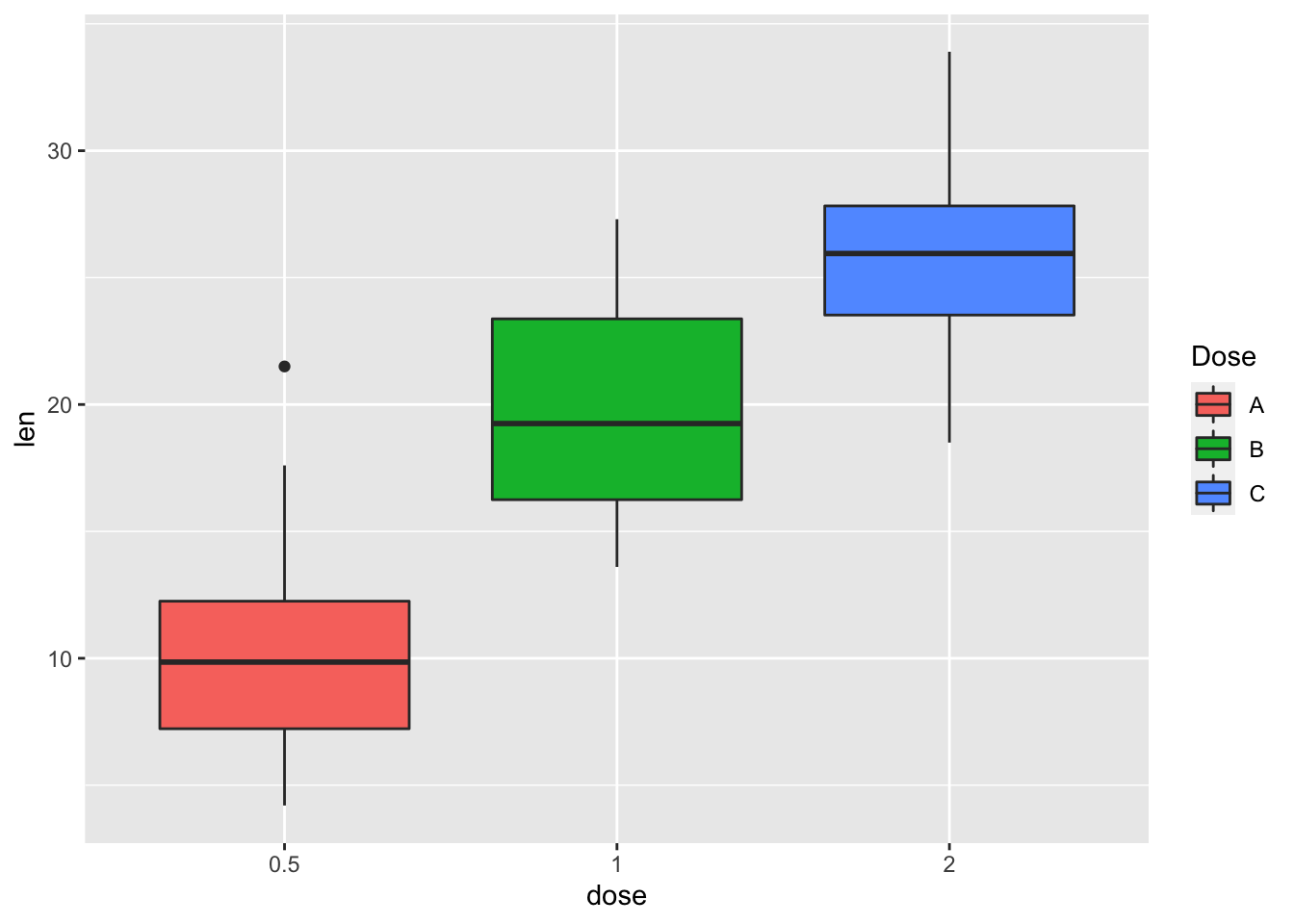### 2.4.5 Convert dose and cyl columns from numeric to factor variables

ToothGrowth$dose <- as.factor(ToothGrowth$dose)
mtcars$cyl <- as.factor(mtcars$cyl)

### 2.4.6 Box plot and scatterplot

bp <- ggplot(ToothGrowth, aes(x=dose, y=len))
# Scatter plot
sp <- ggplot(mtcars, aes(x=wt, y=mpg))
bp + geom_boxplot(fill='steelblue', color="violet")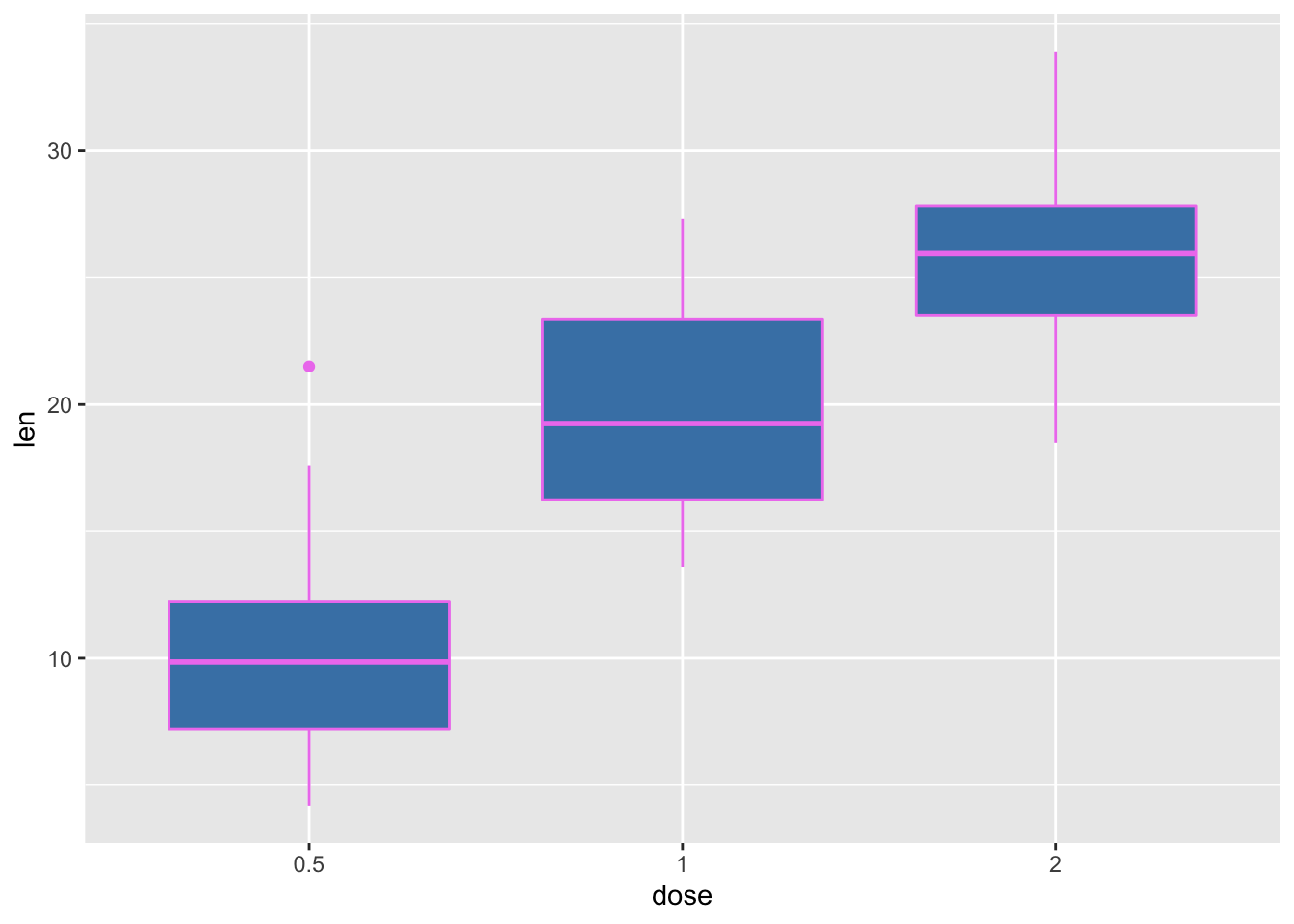# scatter plot
sp + geom_point(color='darkblue')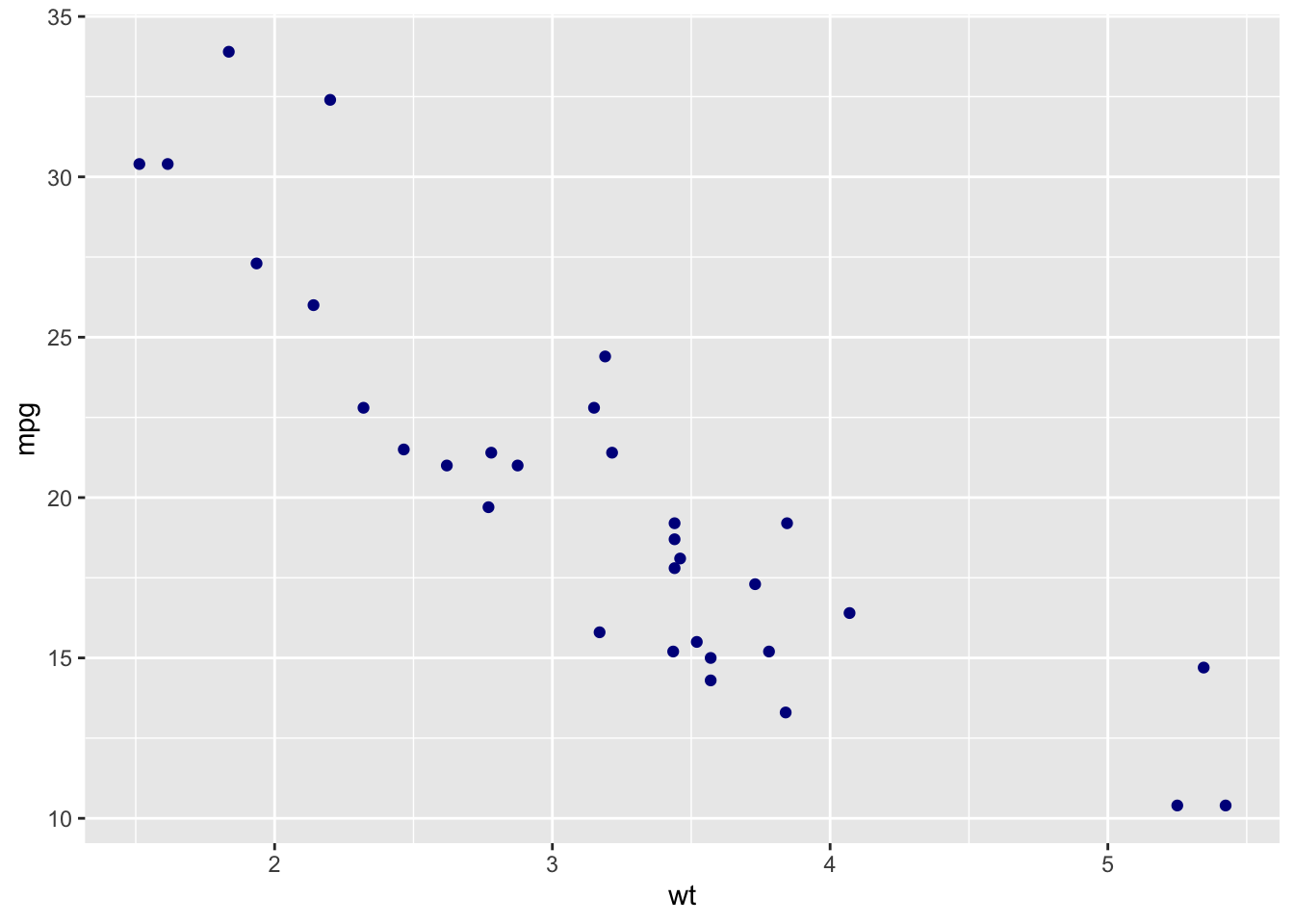### 2.4.7 Box plot

bp <- bp + geom_boxplot(aes(fill = dose))
bp### 2.4.8 Scatter plot

sp <- sp + geom_point(aes(color = cyl))
sp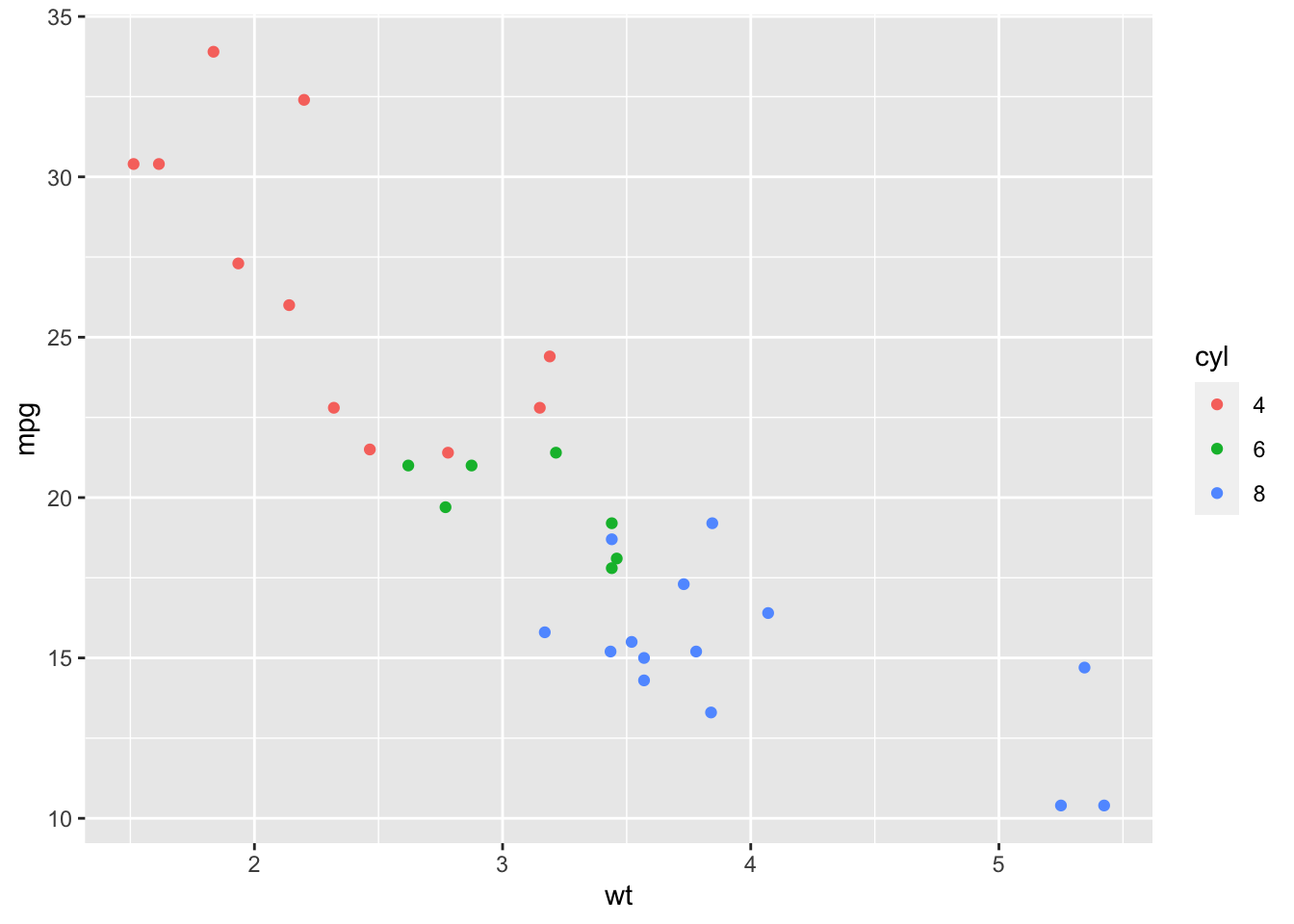## 2.5 Color by qsec values

sp2<-ggplot(mtcars, aes(x=wt, y=mpg)) + geom_point(aes(color = qsec))
sp2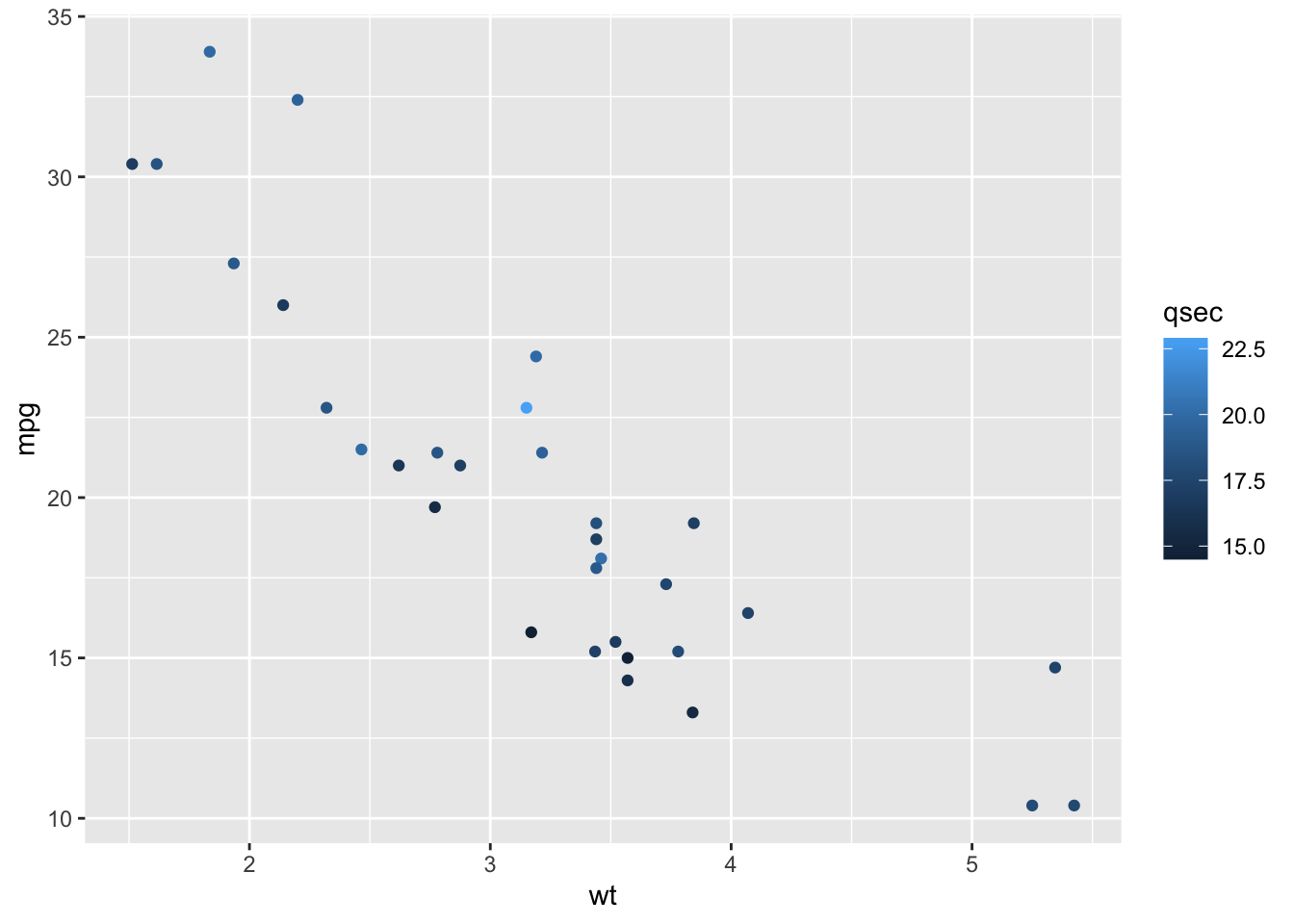## 2.6 Change the low and high colors Sequential color scheme

sp2+scale_color_gradient(low="blue", high="red")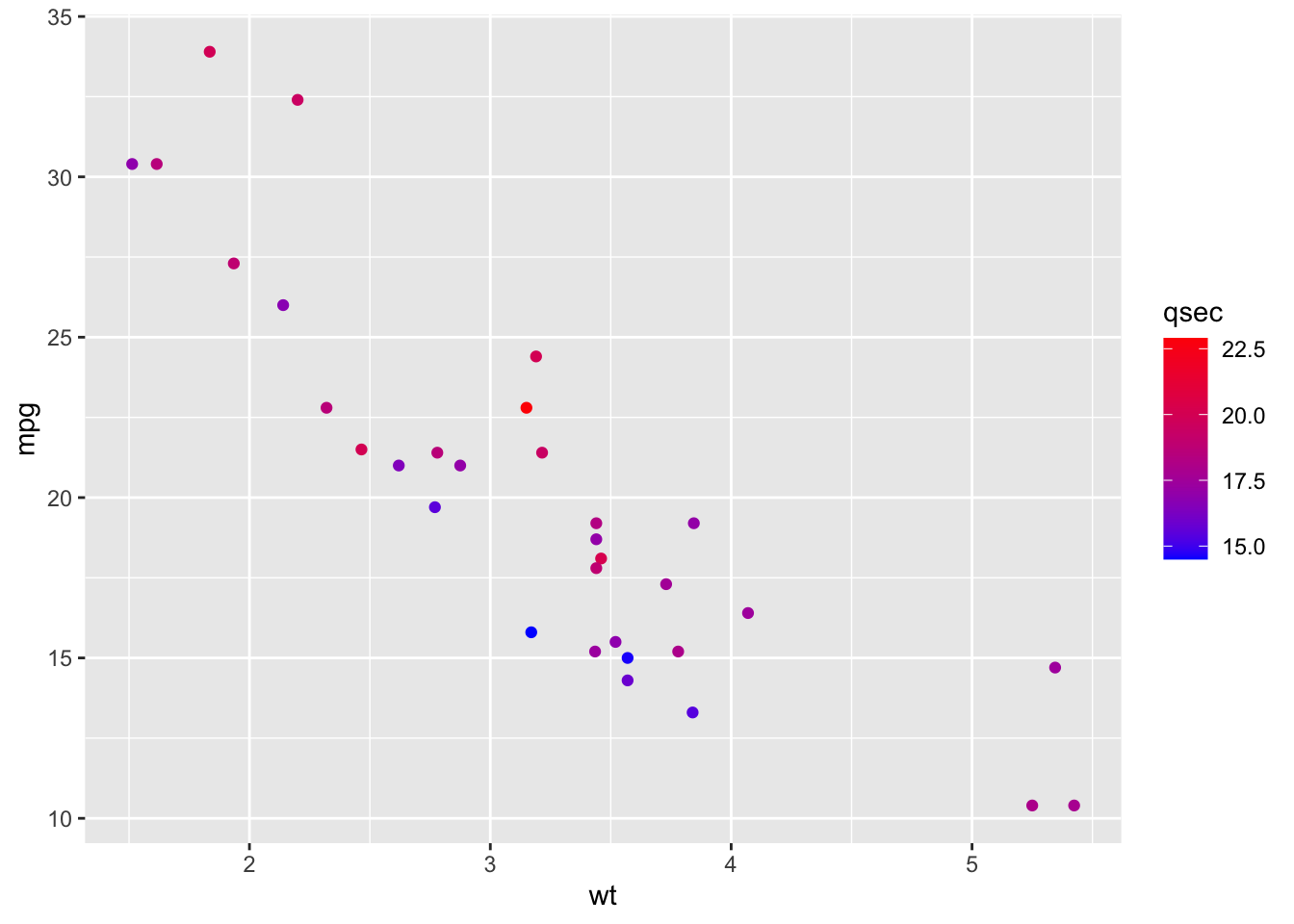## 2.7 Diverging color scheme

mid<-mean(mtcars$qsec) sp2+scale_color_gradient2(midpoint=mid, low="blue", mid="white", high="red", space = "Lab" )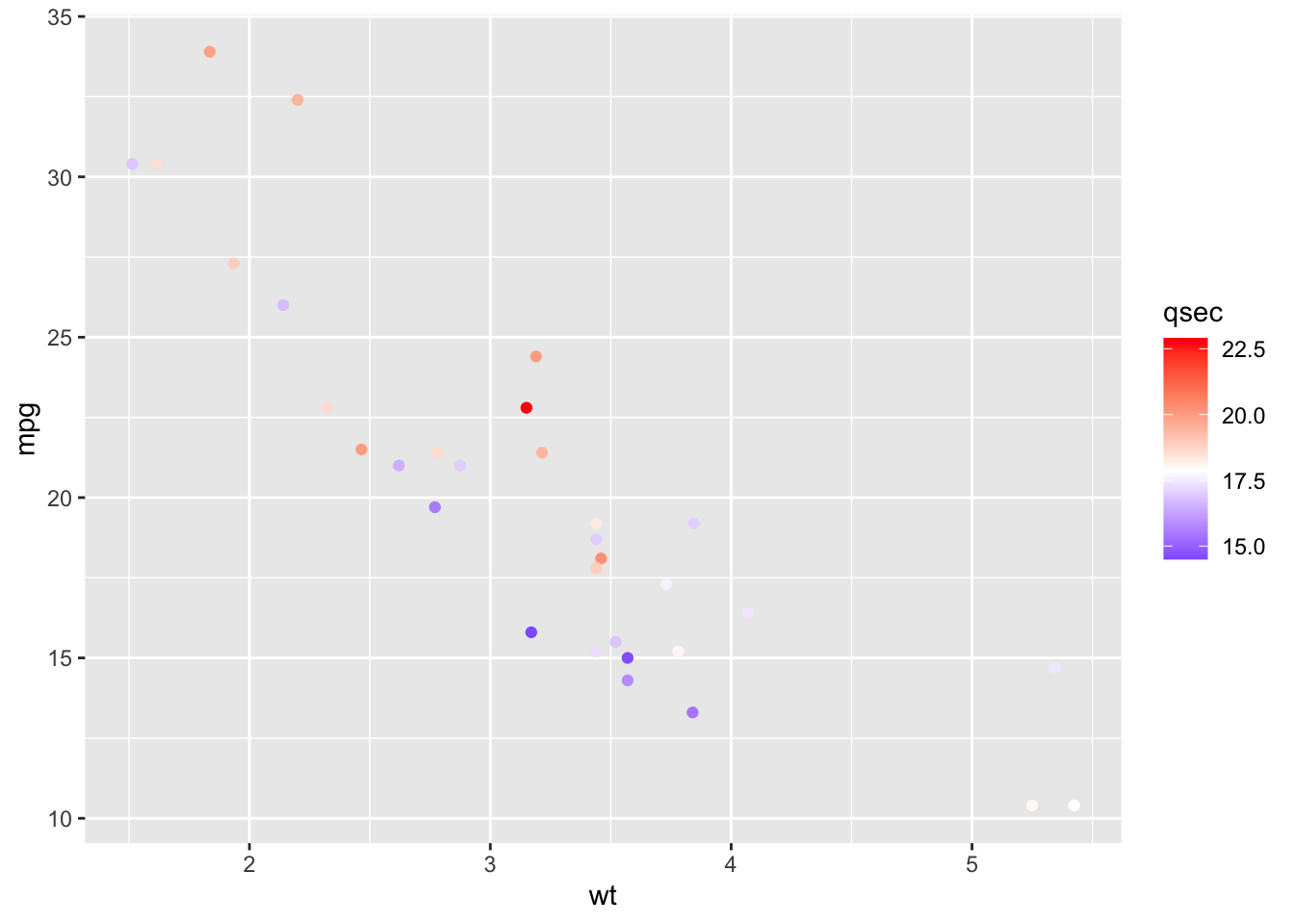## 2.8 Point shapes, colors and size knitr::include_graphics("shapes.png")## 2.9 Convert cyl as factor variable mtcars$cyl <- as.factor(mtcars$cyl) ### 2.9.1 Basic scatter plot ggplot(mtcars, aes(x=wt, y=mpg)) + geom_point(shape = 18, color = "steelblue", size = 4)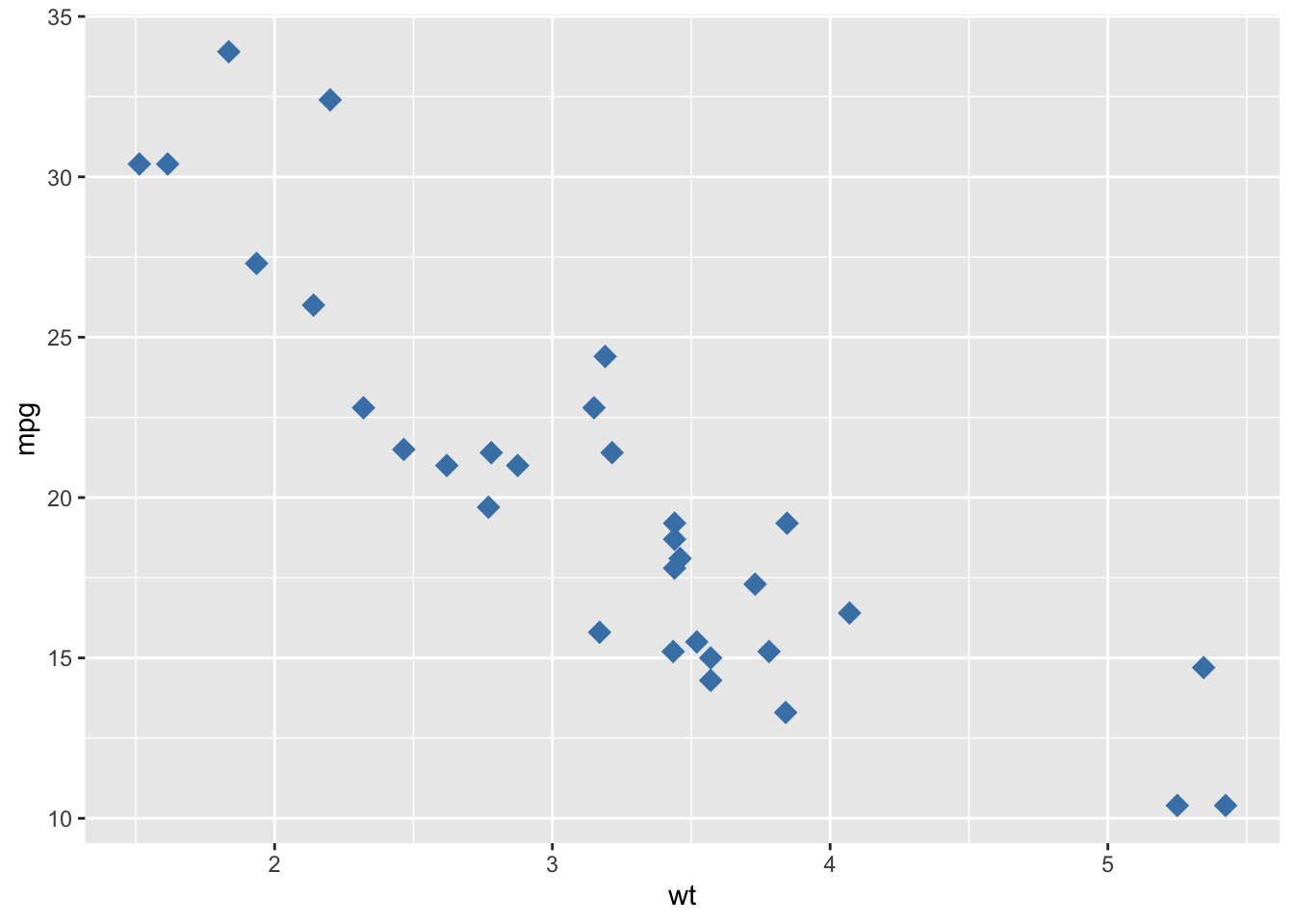### 2.9.2 Change point shapes and colors by groups ggplot(mtcars, aes(x=wt, y=mpg)) + geom_point(aes(shape = cyl, color = cyl))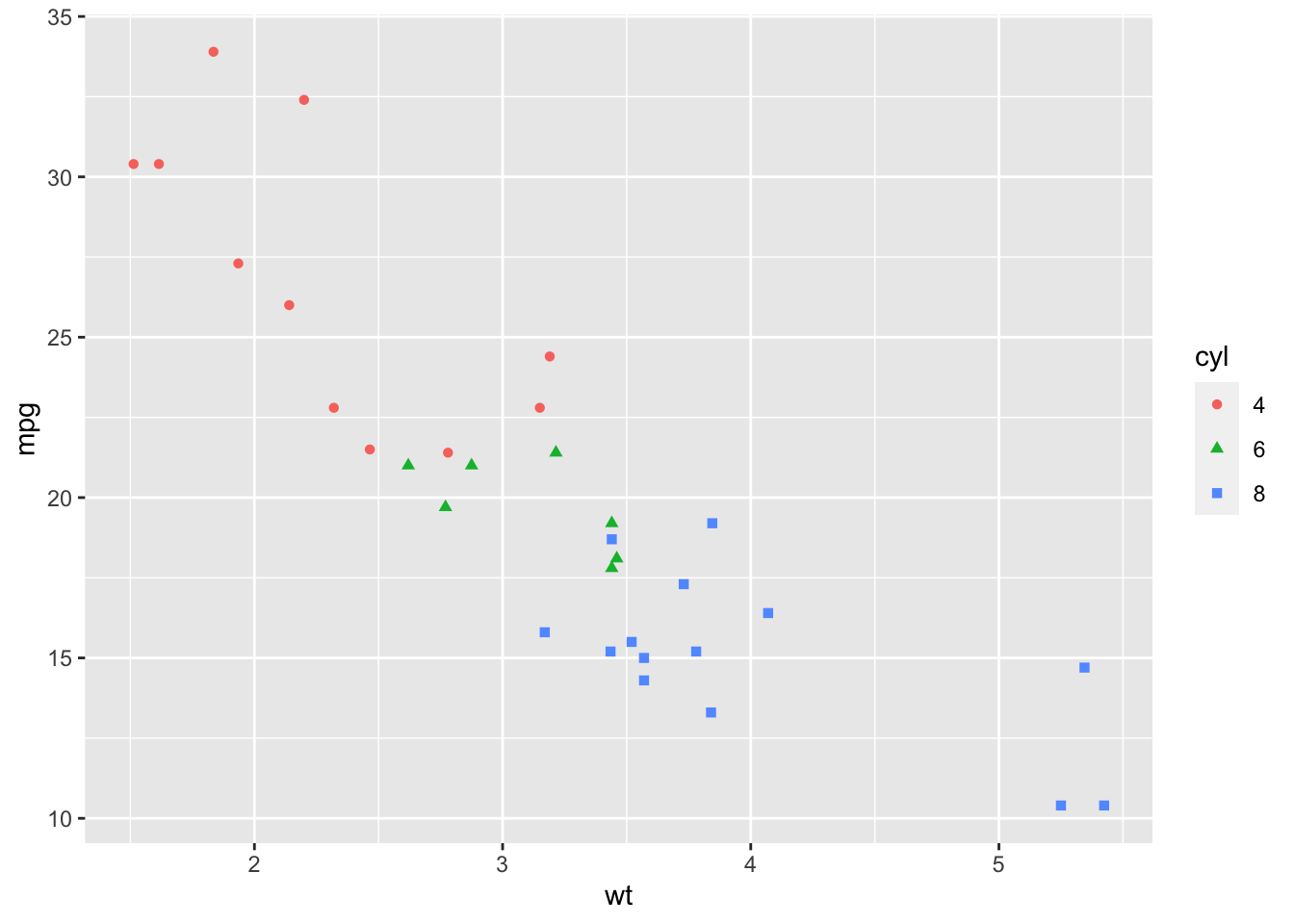### 2.9.3 Change colors and shapes manually ggplot(mtcars, aes(x=wt, y=mpg, group=cyl)) + geom_point(aes(shape=cyl, color=cyl), size=2)+ scale_shape_manual(values=c(3, 16, 17))+ scale_color_manual(values=c('#999999','#E69F00', '#56B4E9'))+ theme(legend.position="top")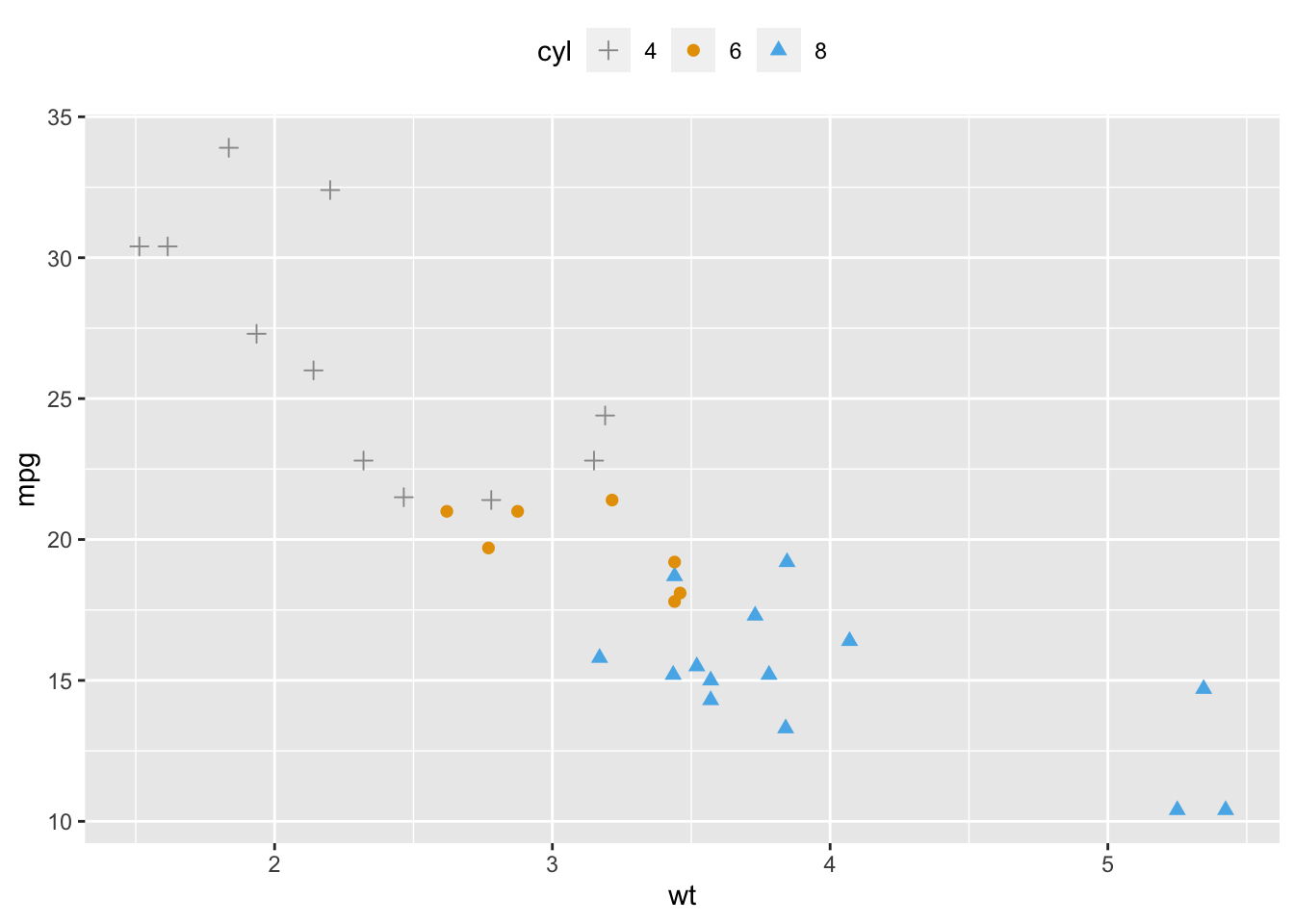### 2.9.4 Add text annotations to a graph set.seed(1234) df <- mtcars[sample(1:nrow(mtcars), 10), ] df$cyl <- as.factor(df$cyl) ### 2.9.5 Line types knitr::include_graphics("lines.png")## 2.10 Basic line plot ### 2.10.1 Create some data df <- data.frame(time=c("breakfeast", "Lunch", "Dinner"), bill=c(10, 30, 15)) head(df) ## time bill ## 1 breakfeast 10 ## 2 Lunch 30 ## 3 Dinner 15 ### 2.10.2 Basic line plot with points Change the line type ggplot(data=df, aes(x=time, y=bill, group=1)) + geom_line(linetype = "dashed")+ geom_point()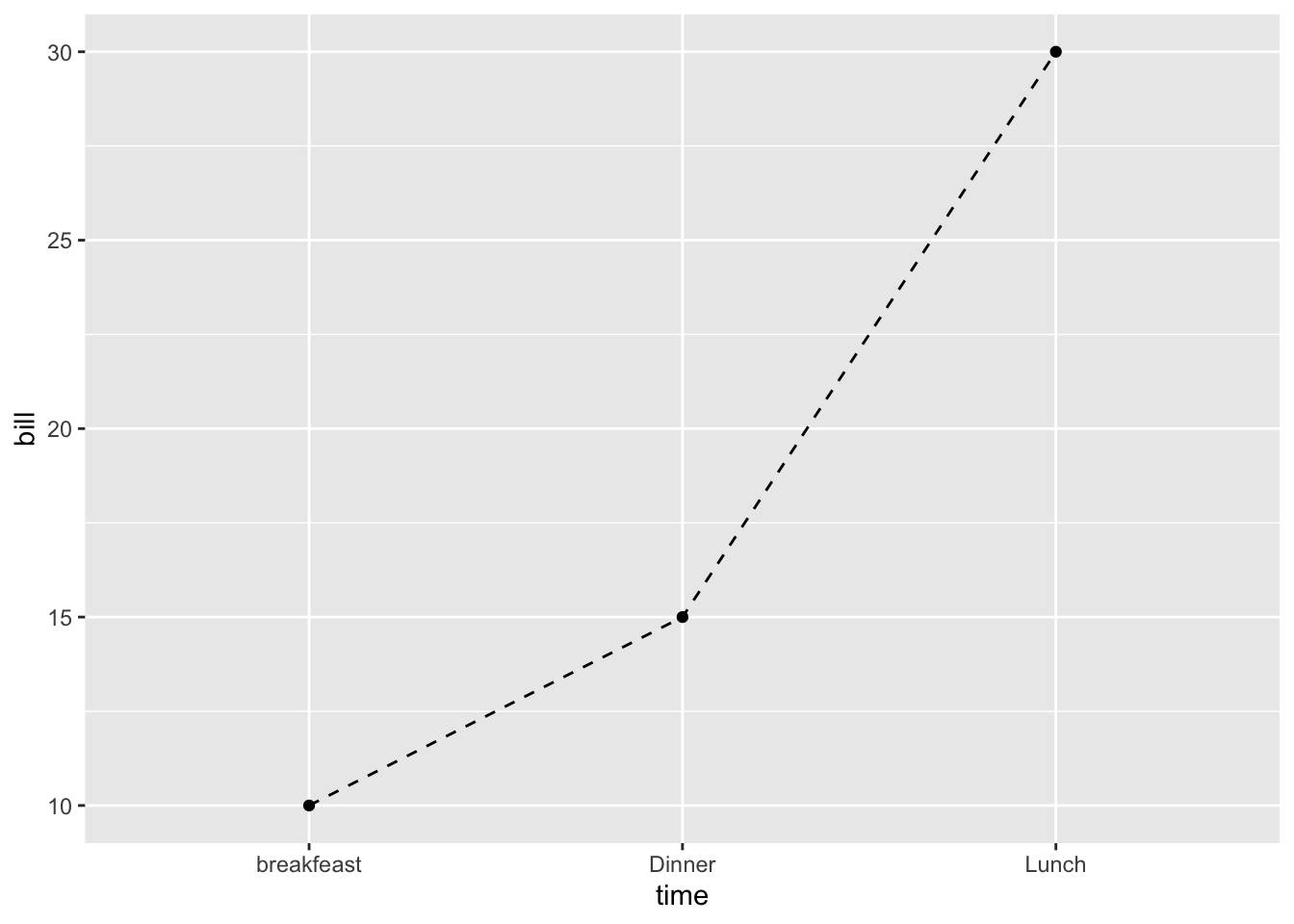## 2.11 Line plots with multiple groups ### 2.11.1 Create some data df2 <- data.frame(sex = rep(c("Female", "Male"), each=3), time=c("breakfeast", "Lunch", "Dinner"), bill=c(10, 30, 15, 13, 40, 17) ) head(df2, n=3) ## sex time bill ## 1 Female breakfeast 10 ## 2 Female Lunch 30 ## 3 Female Dinner 15 ### 2.11.2 Change line types and colors by groups (sex) ggplot(df2, aes(x=time, y=bill, group=sex)) + geom_line(aes(linetype = sex, color = sex))+ geom_point(aes(color=sex))+ theme(legend.position="top")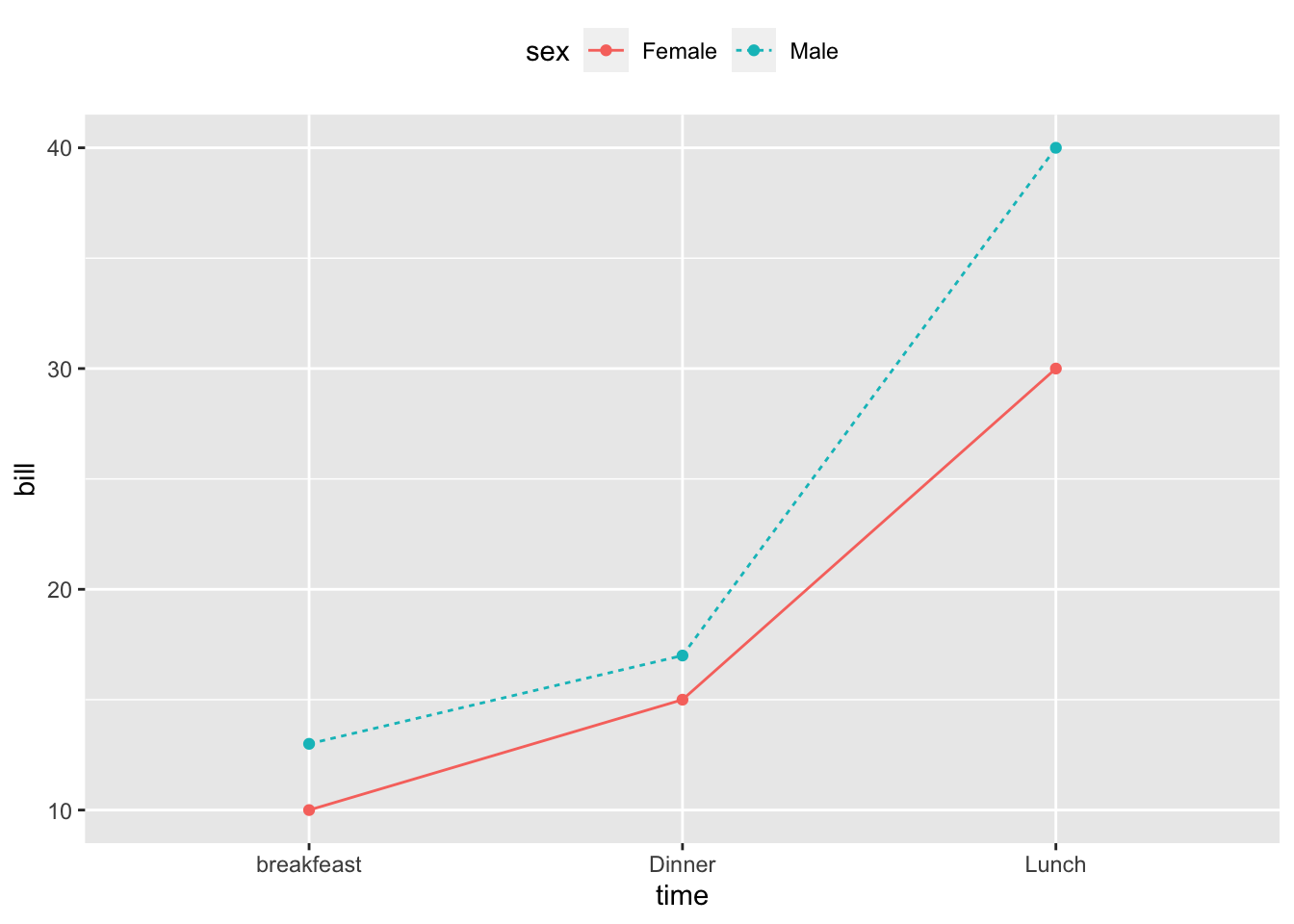## 2.12 Change line types, colors and sizes ggplot(df2, aes(x=time, y=bill, group=sex)) + geom_line(aes(linetype=sex, color=sex, size=sex))+ geom_point()+ scale_linetype_manual(values=c("twodash", "dotted"))+ scale_color_manual(values=c('#999999','#E69F00'))+ scale_size_manual(values=c(1, 1.5))+ theme(legend.position="top")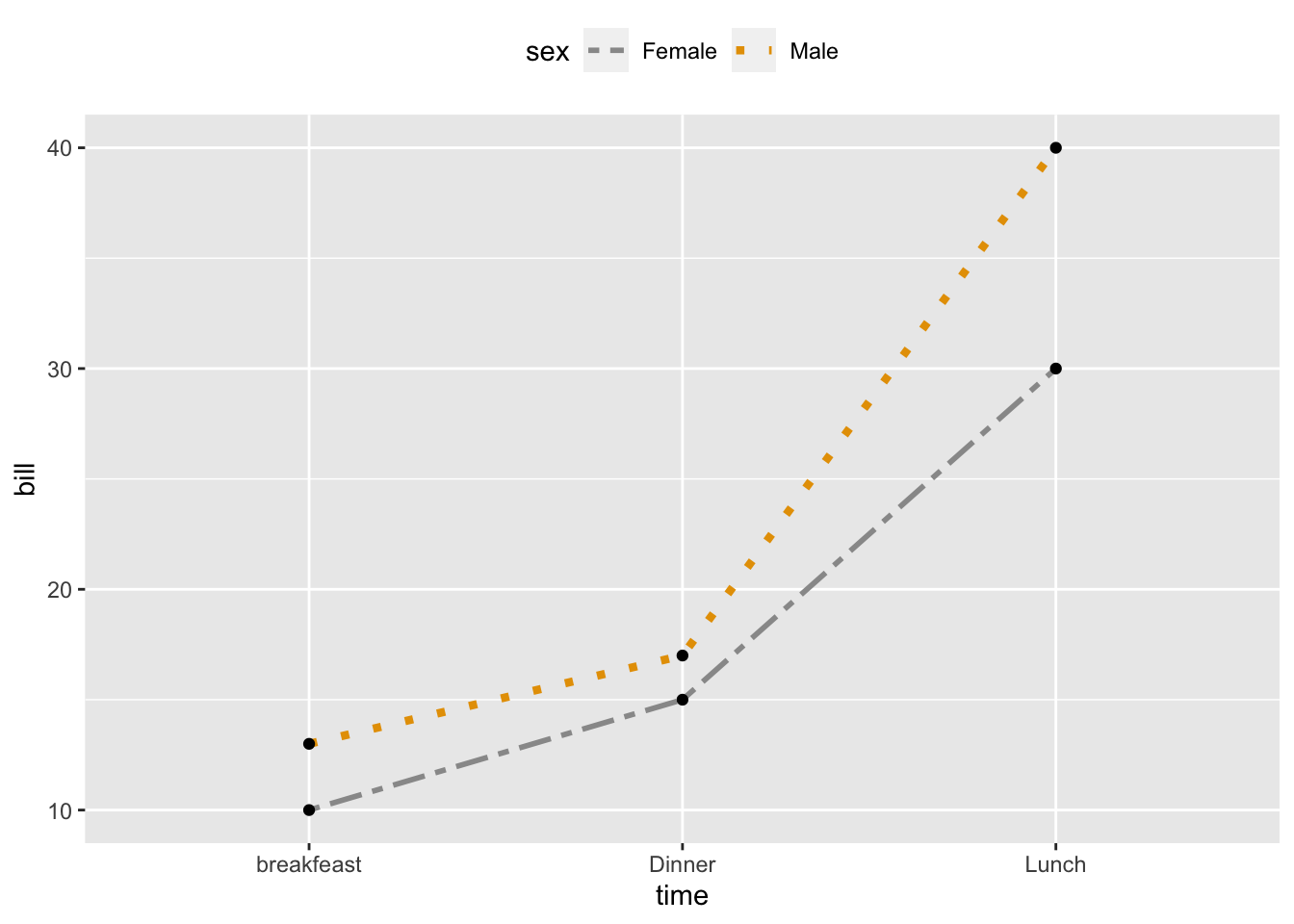## 2.13 Themes and background colors ### 2.13.1 Convert the column dose from numeric to factor variable ToothGrowth$dose <- as.factor(ToothGrowth$dose) #Create a box plot p <- ggplot(ToothGrowth, aes(x=dose, y=len)) + geom_boxplot() ## 2.14 Change plot themes p + theme_gray(base_size = 14)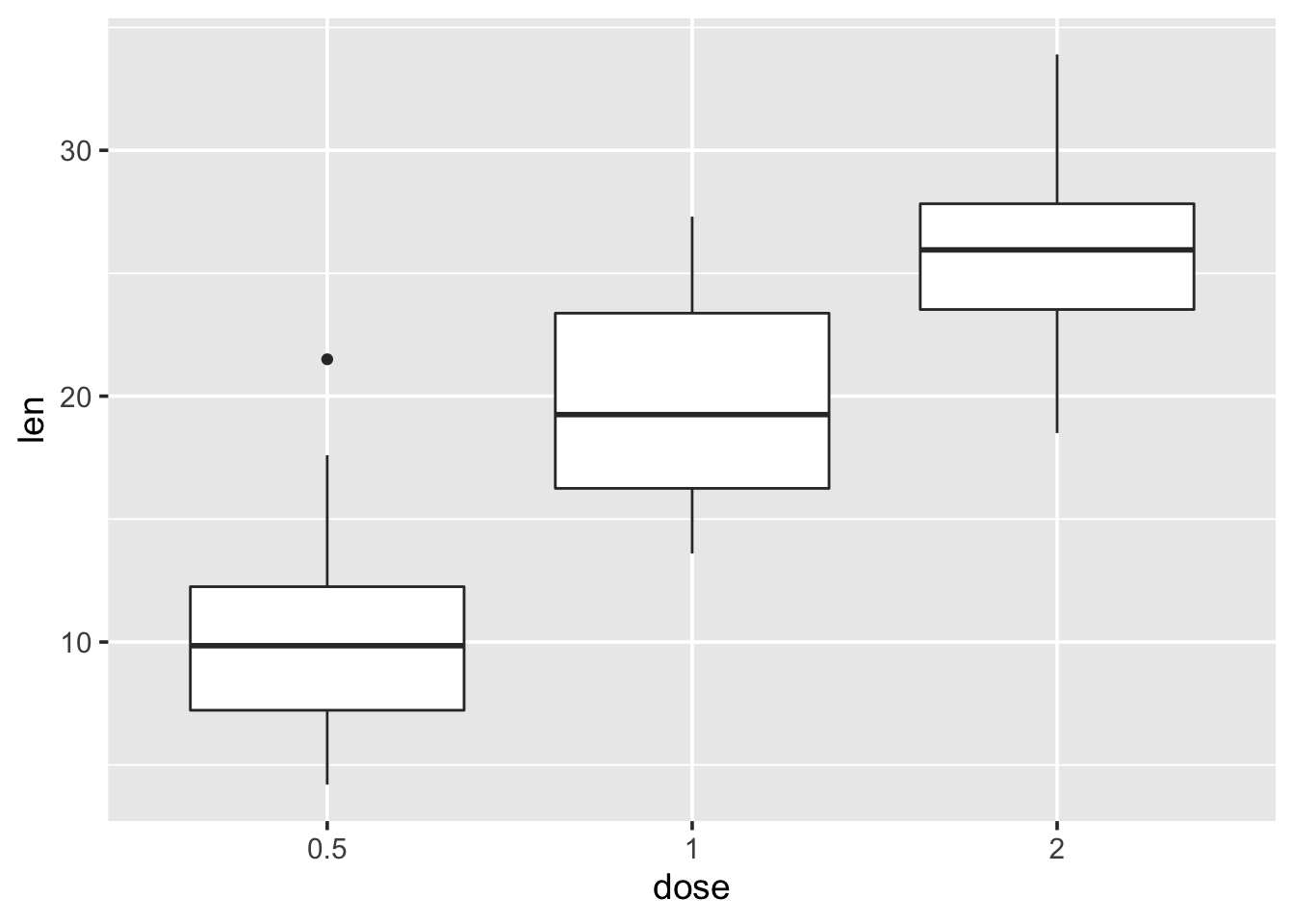p + theme_bw()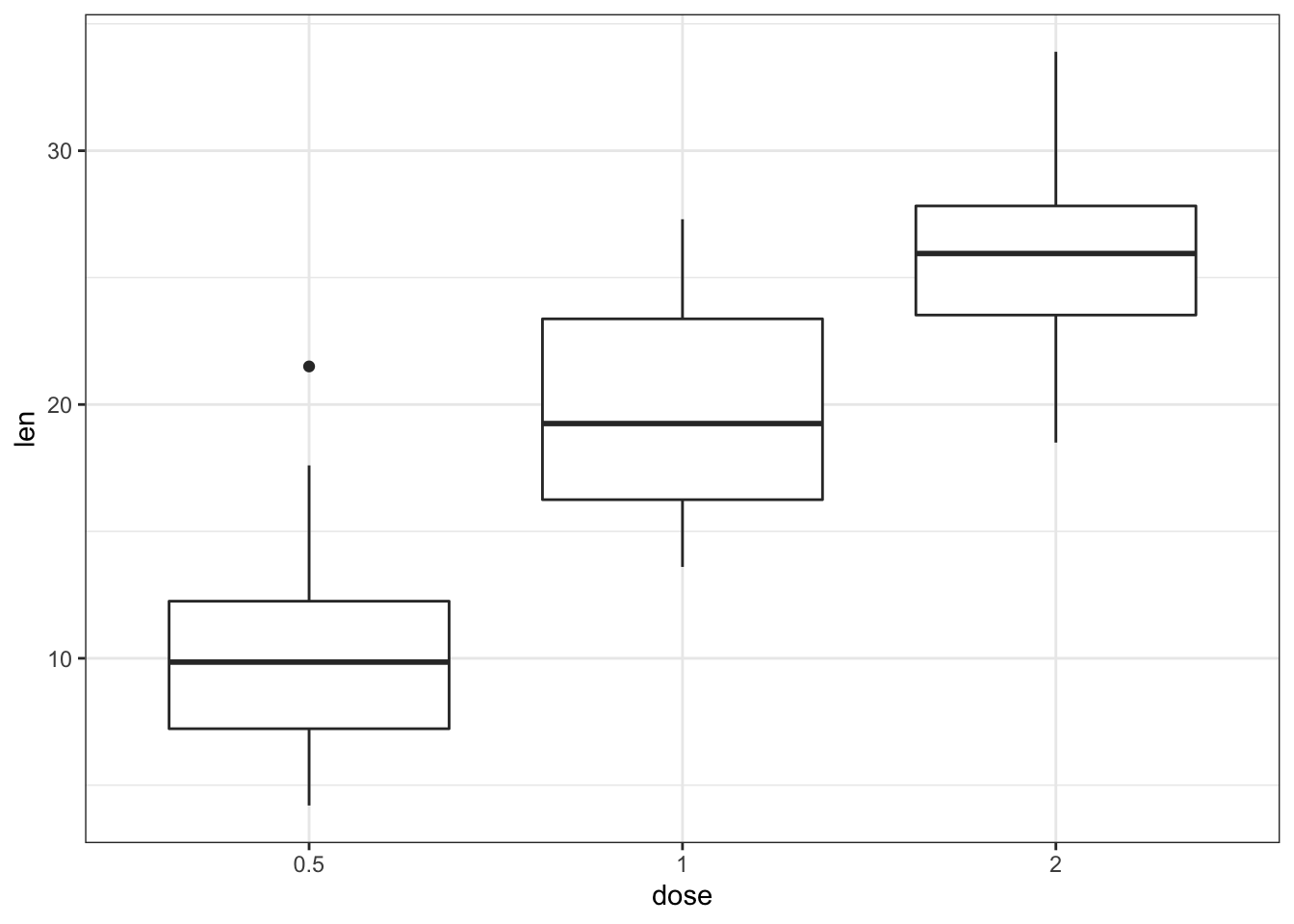p + theme_linedraw()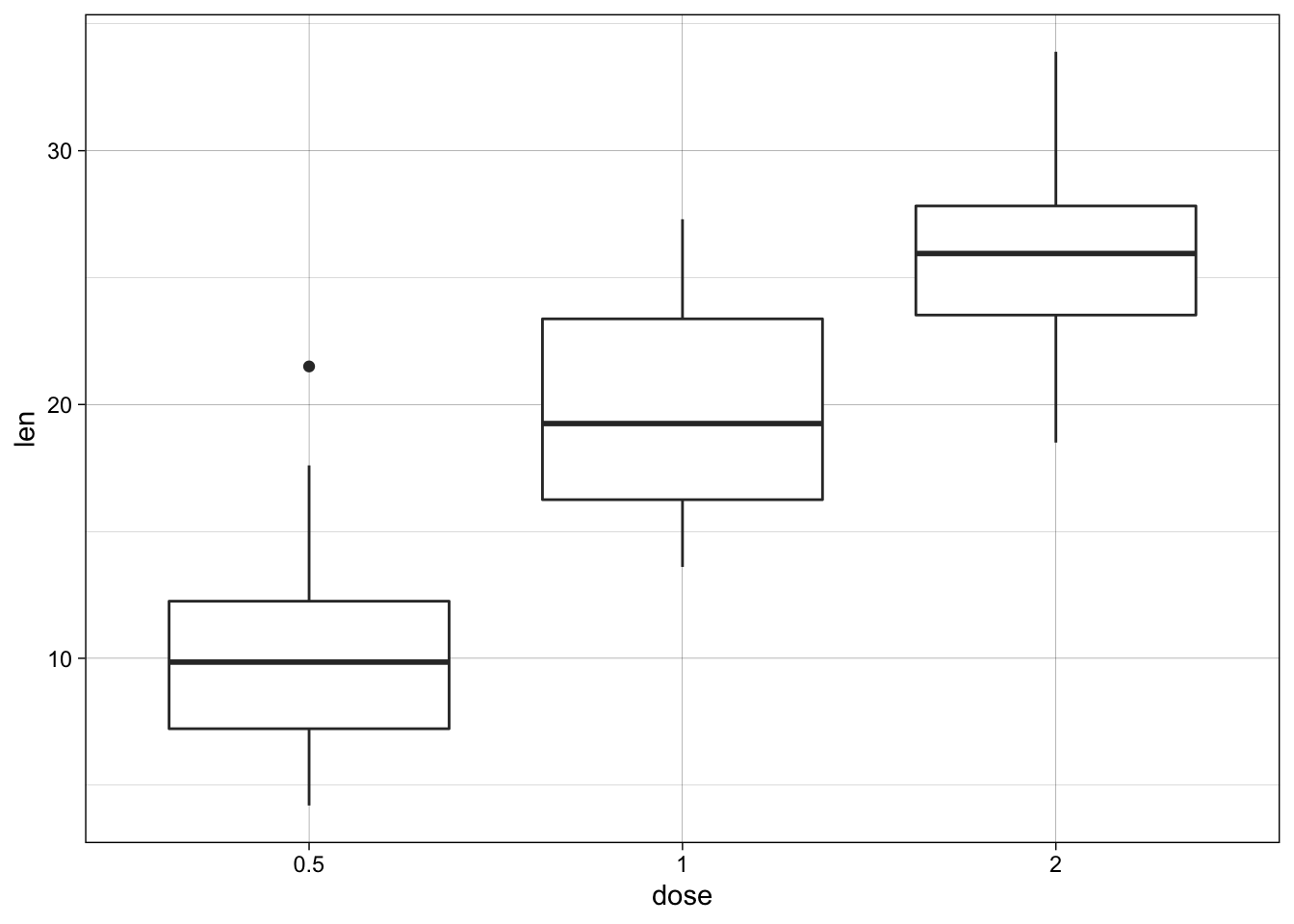p + theme_light()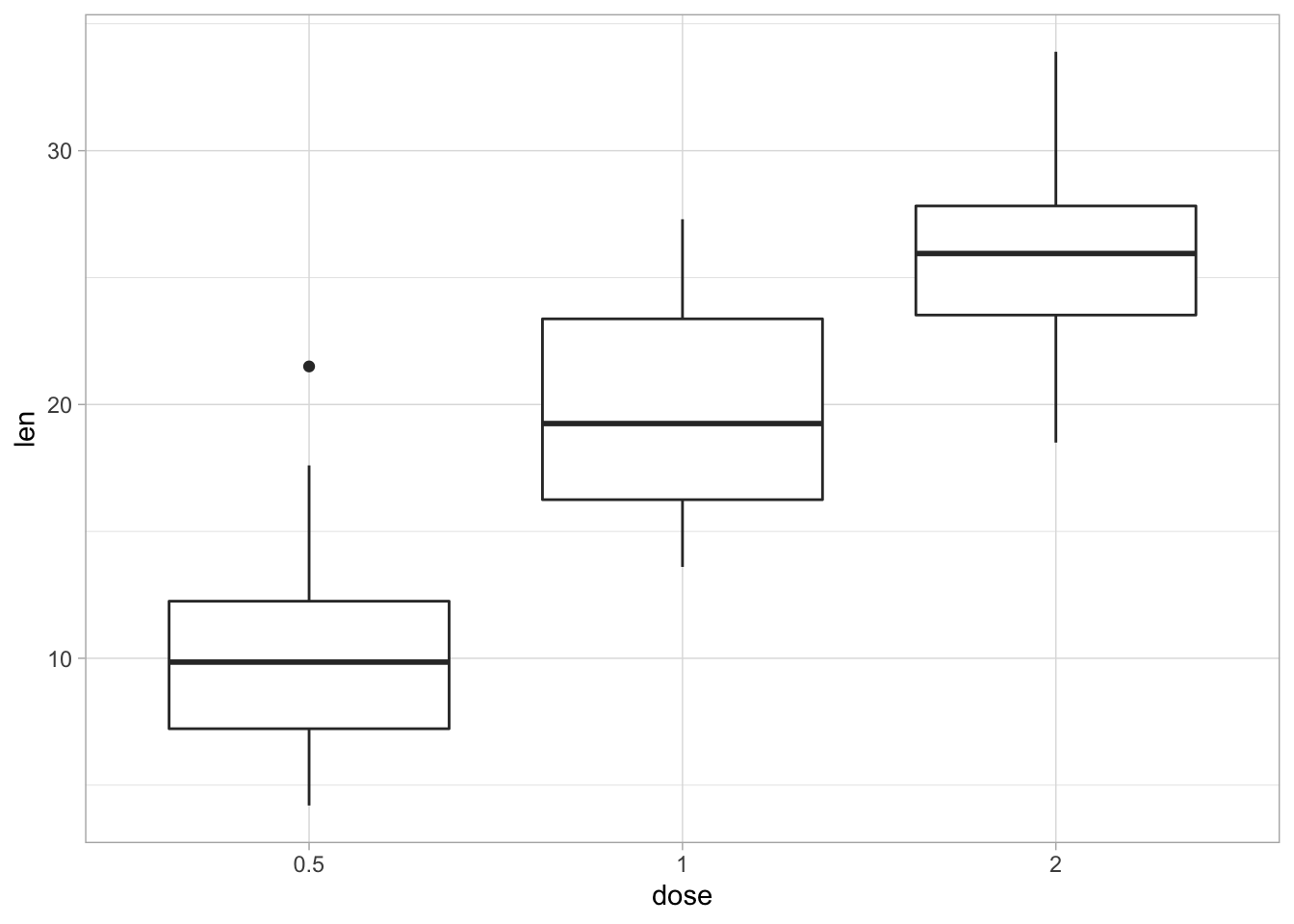p + theme_minimal()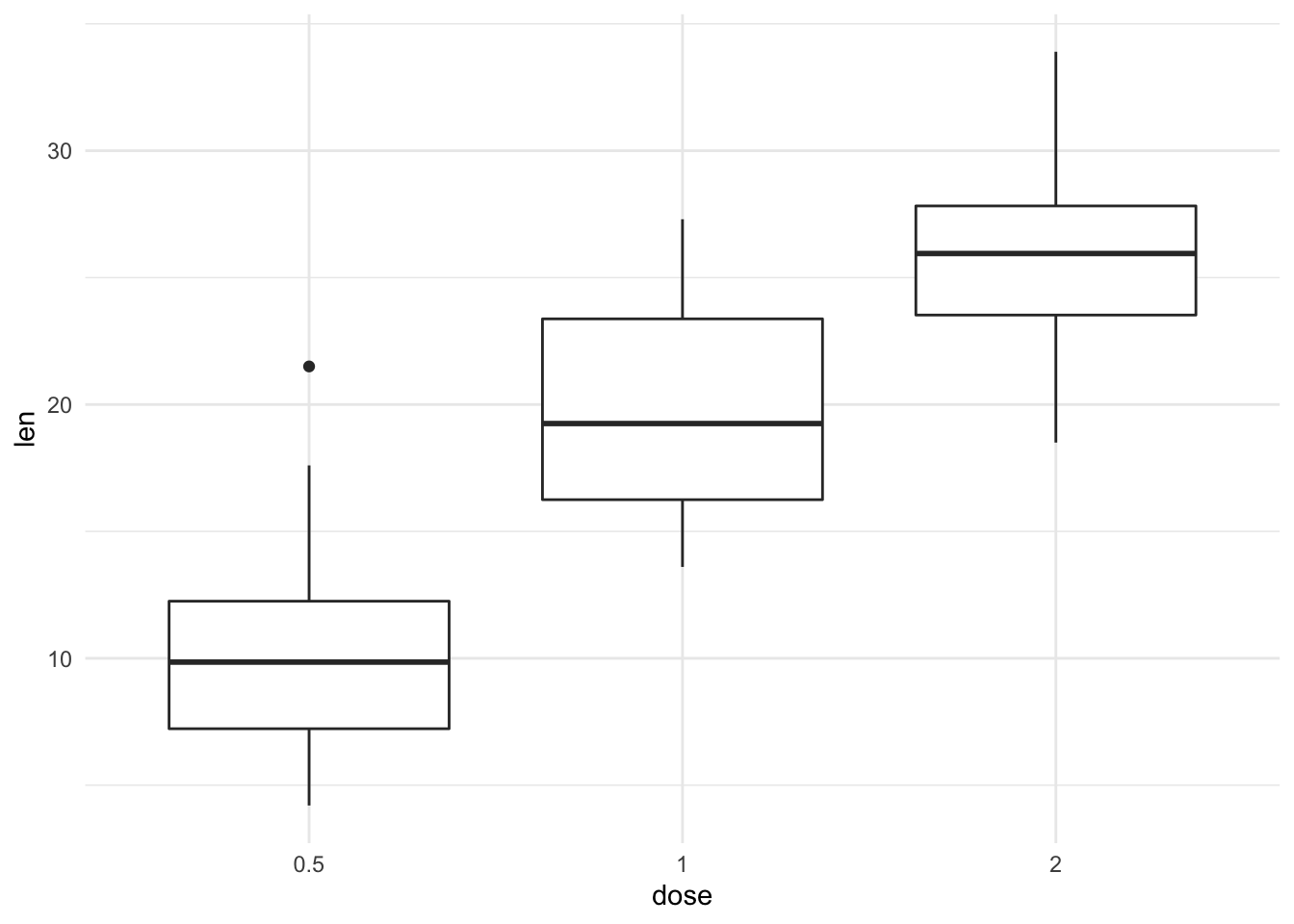p + theme_classic()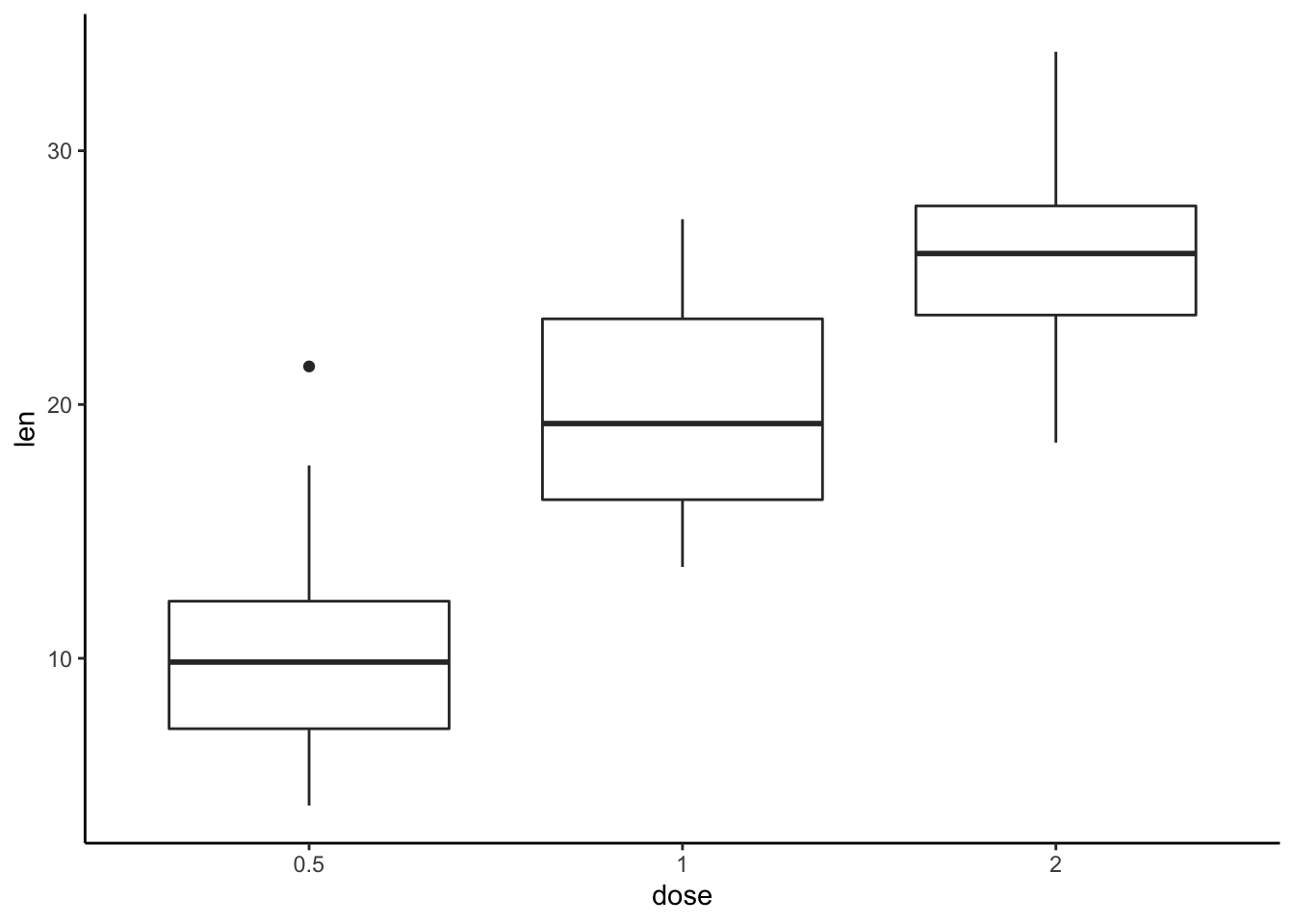## 2.15 Axis limits: Minimum and Maximum values p <- ggplot(cars, aes(x = speed, y = dist)) + geom_point() ### 2.15.1 Different functions are available for setting axis limits: ## 2.16 Default plot print(p) ## Change axis limits using coord_cartesian() p + coord_cartesian(xlim =c(5, 20), ylim = c(0, 50))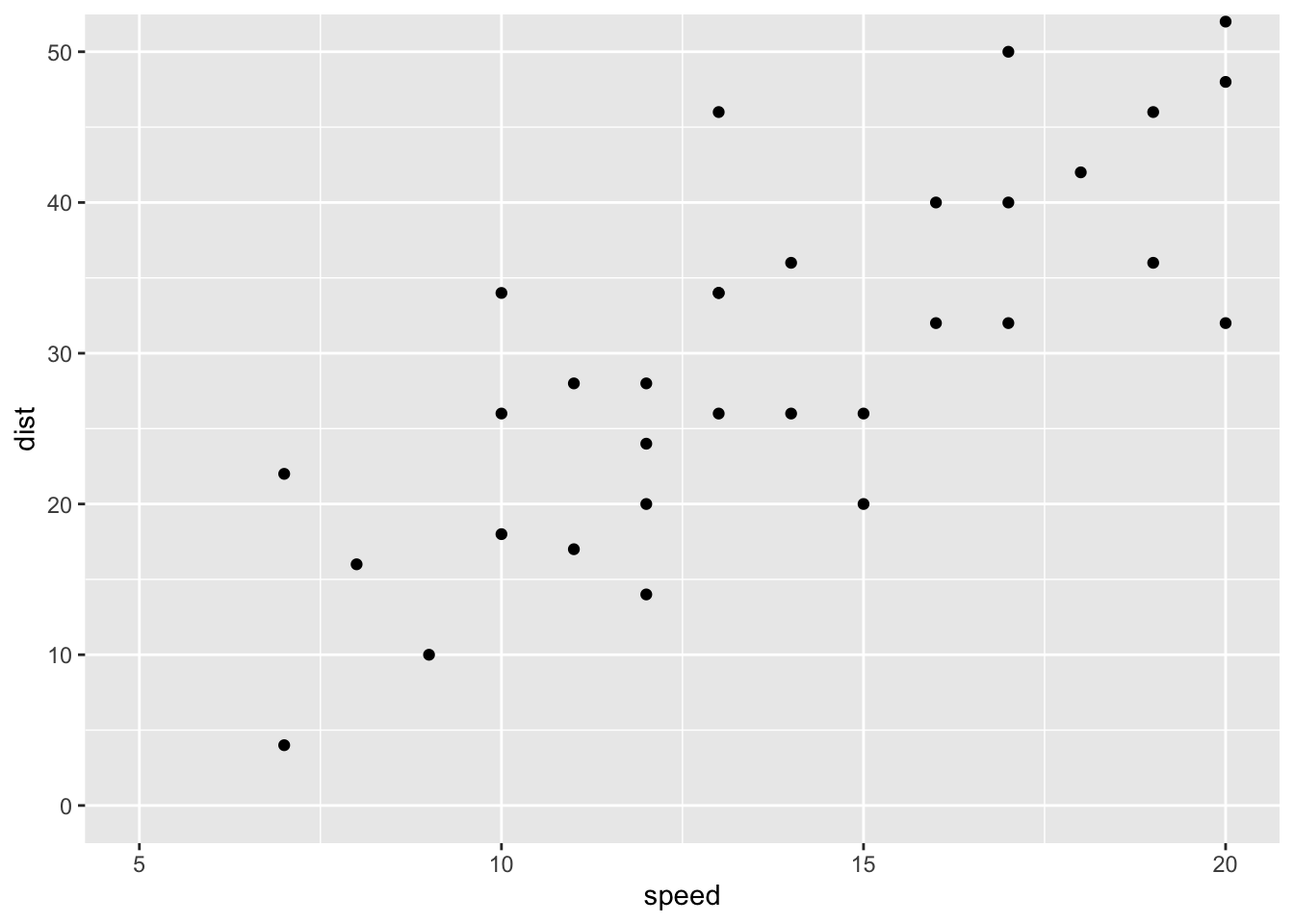# Use xlim() and ylim() p + xlim(5, 20) + ylim(0, 50)  ## Warning: Removed 19 rows containing missing values (geom_point).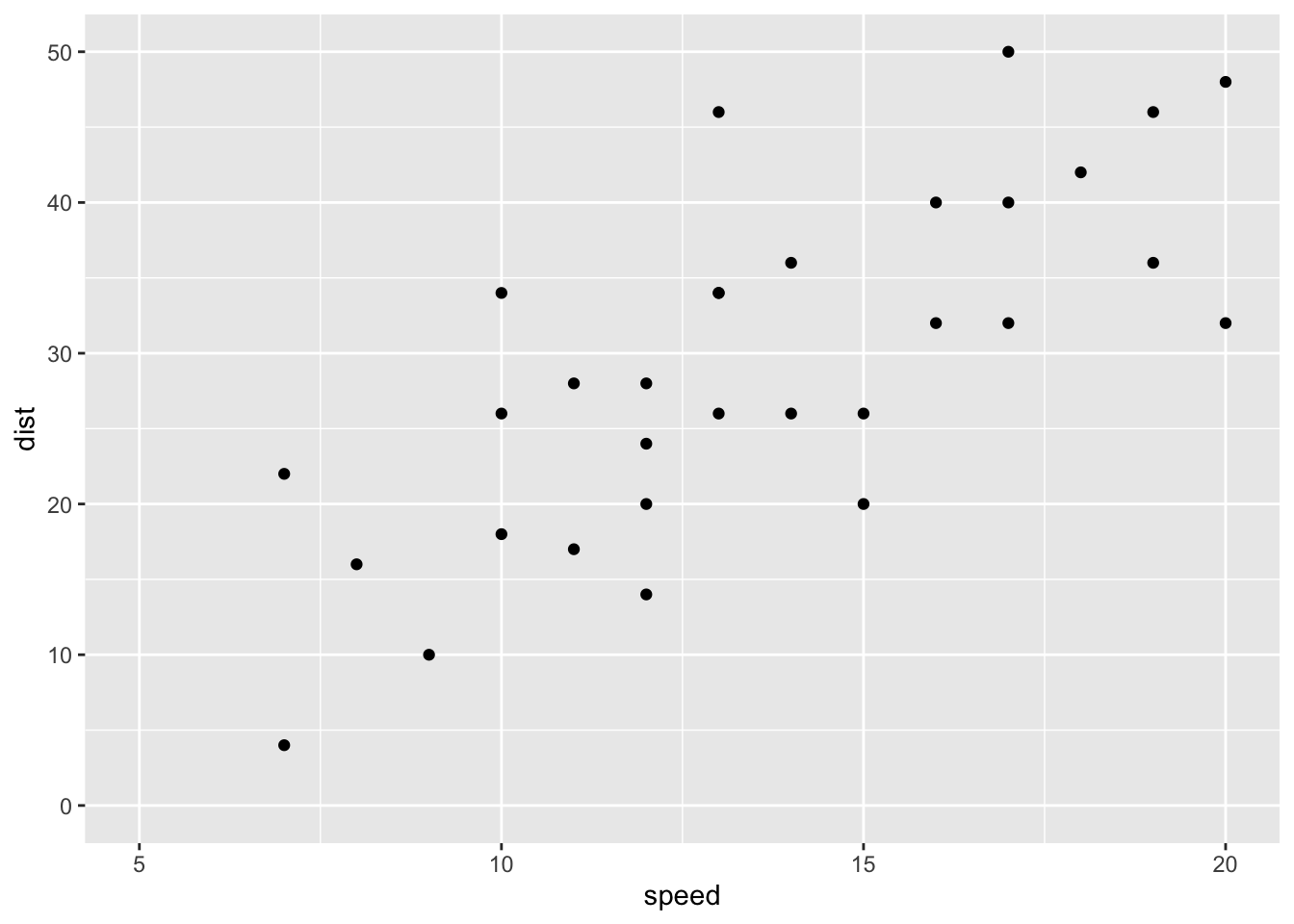# Expand limits p + expand_limits(x = c(5, 50), y = c(0, 150))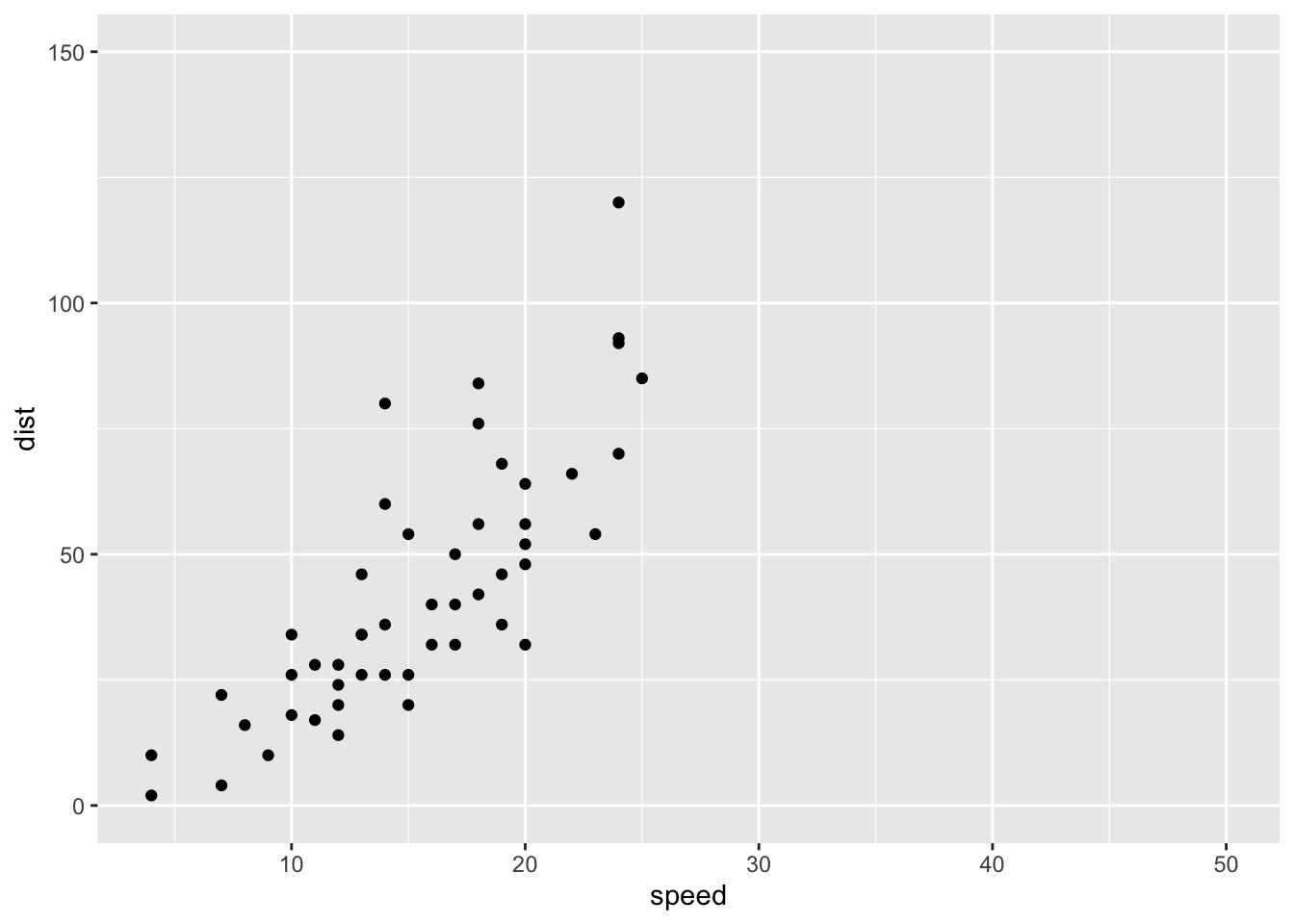## 2.17 Axis transformations: log and sqrt scales ### 2.17.1 Create a scatter plot: p <- ggplot(cars, aes(x = speed, y = dist)) + geom_point() ## 2.18 Log transformation using scale_xx() # possible values for trans : 'log2', 'log10','sqrt' p + scale_x_continuous(trans='log2') + scale_y_continuous(trans='log2')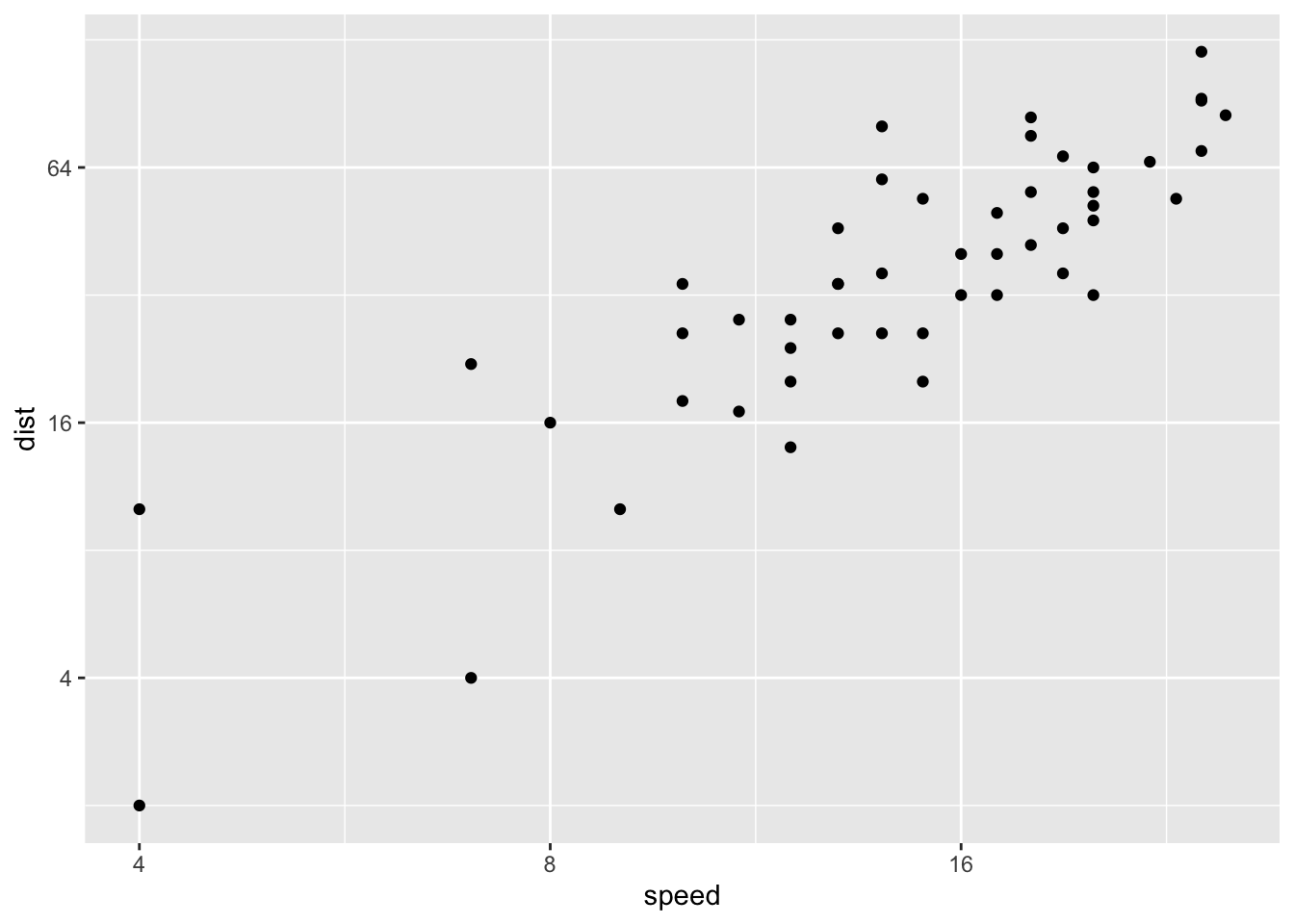## 2.19 Format axis tick mark labels require(scales) ## Loading required package: scales ## ## Attaching package: 'scales' ## The following object is masked from 'package:readr': ## ## col_factor p + scale_y_continuous(trans = log2_trans(), breaks = trans_breaks("log2", function(x) 2^x), labels = trans_format("log2", math_format(2^.x)))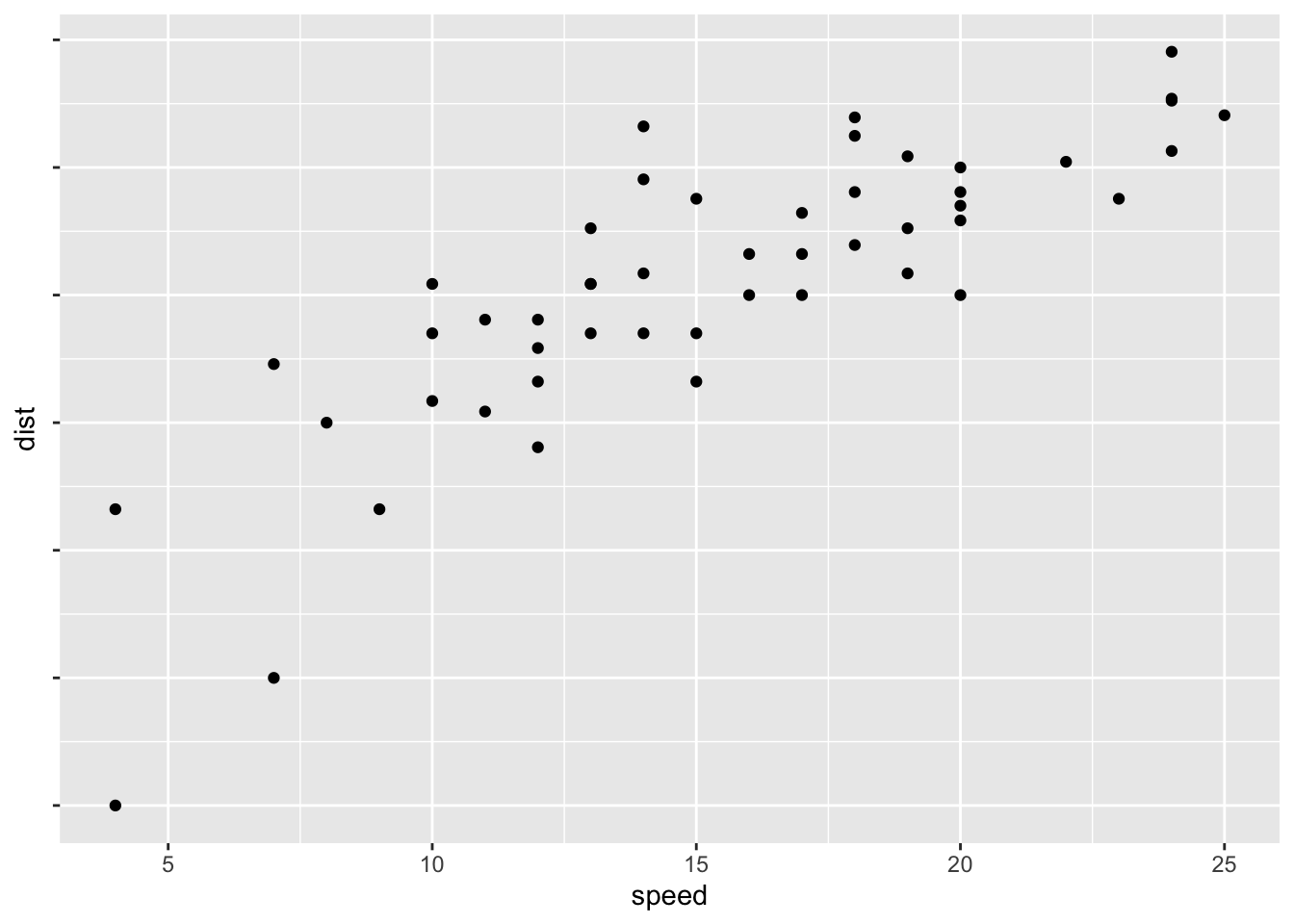# Reverse coordinates p + scale_y_reverse()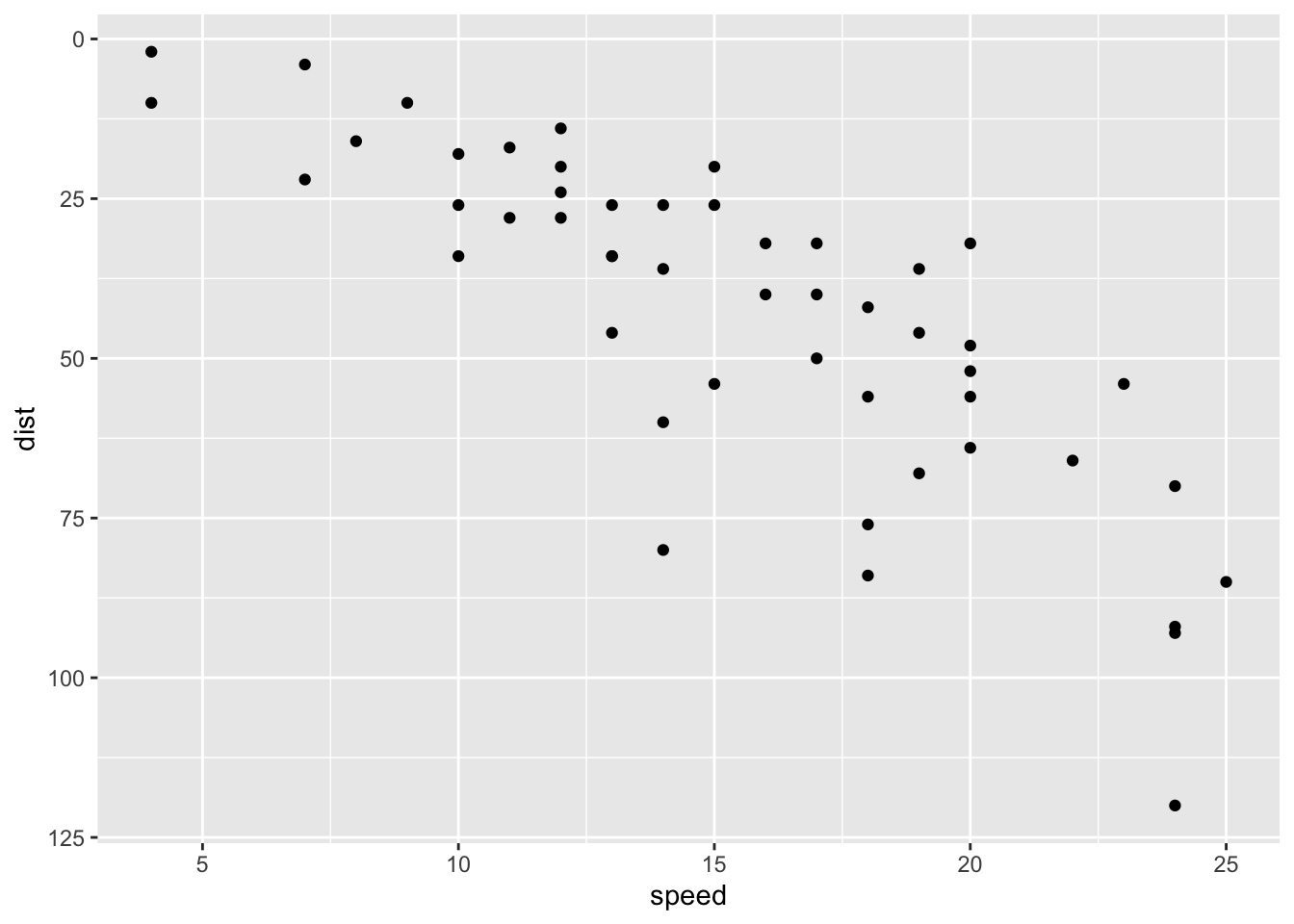## 2.20 Axis ticks: customize tick marks and labels, reorder and select items ### 2.20.1 Create a box plot: p <- ggplot(ToothGrowth, aes(x=dose, y=len)) + geom_boxplot()  ## 2.21 Change the style and the orientation angle of axis tick labels face can be "plain", "italic", "bold" or "bold.italic" p + theme(axis.text.x = element_text(face="bold", color="#993333", size=14, angle=45), axis.text.y = element_text(face="bold", color="blue", size=14, angle=45))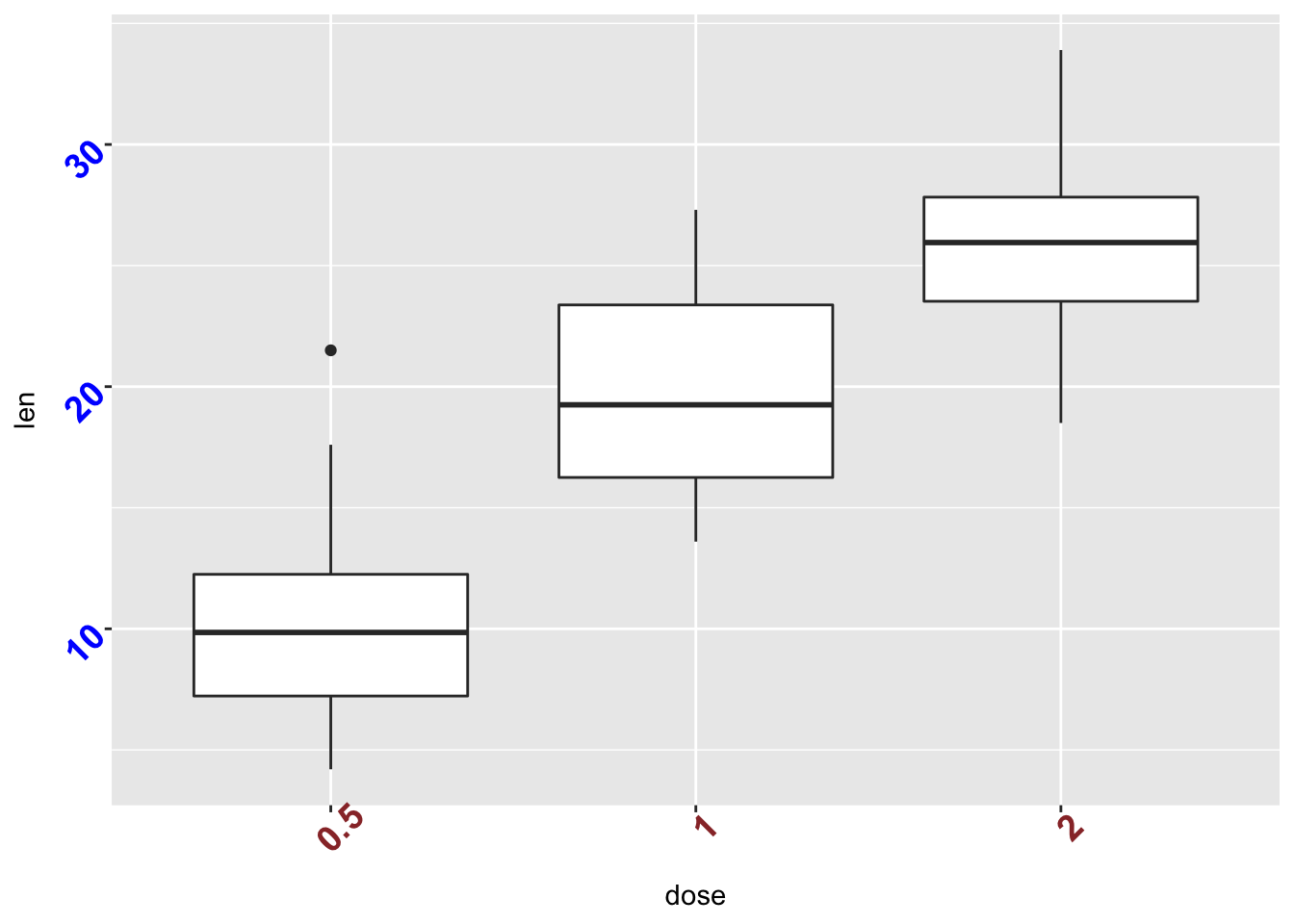# Remove axis ticks and tick mark labels p + theme( axis.text.x = element_blank(), # Remove x axis tick labels axis.text.y = element_blank(), # Remove y axis tick labels axis.ticks = element_blank()) # Remove ticks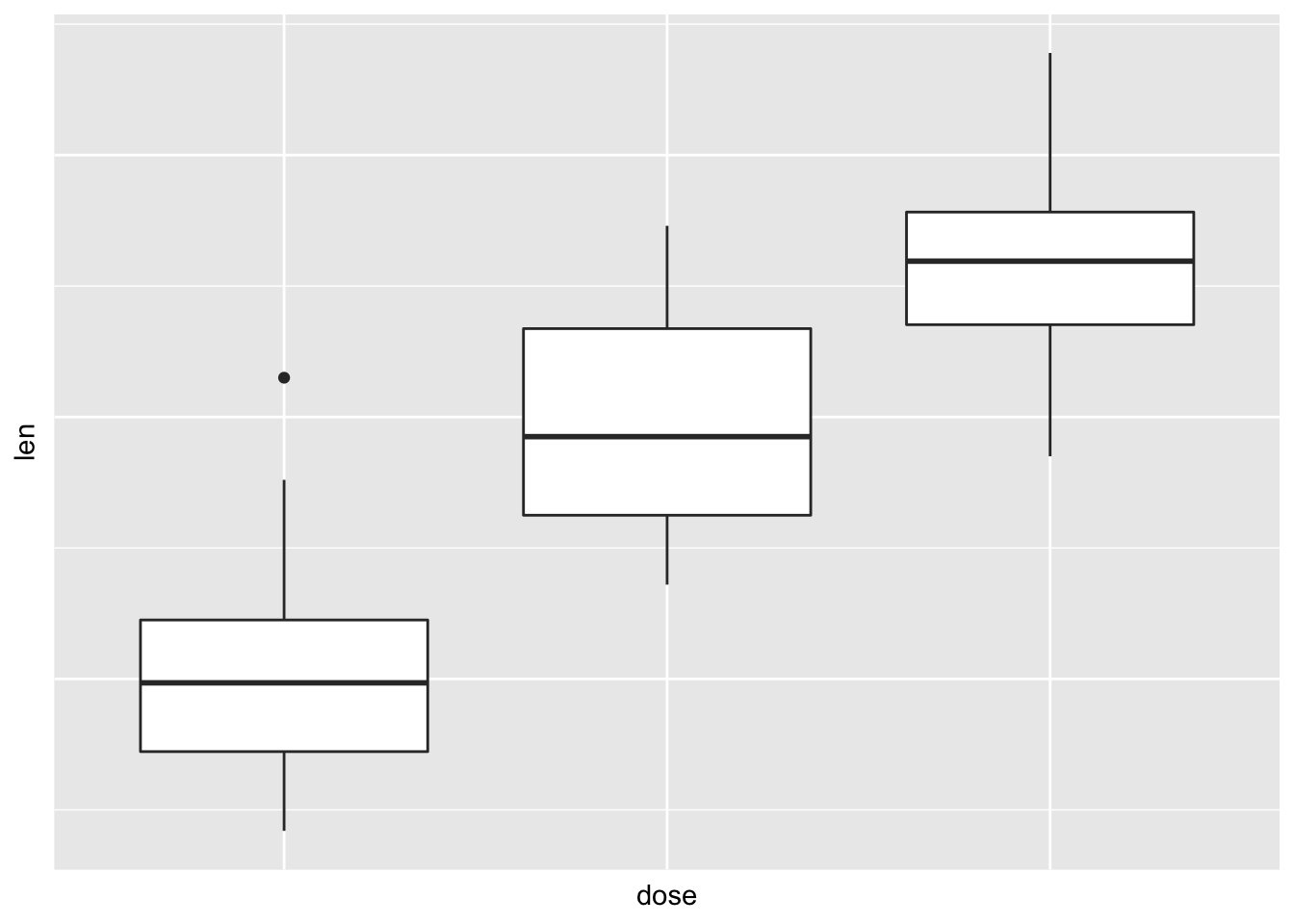## Customize continuous and discrete axes: ### Discrete axes: ### 2.21.1 Change x axis label and the order of items p + scale_x_discrete(name ="Dose (mg)", limits=c("2","1","0.5"))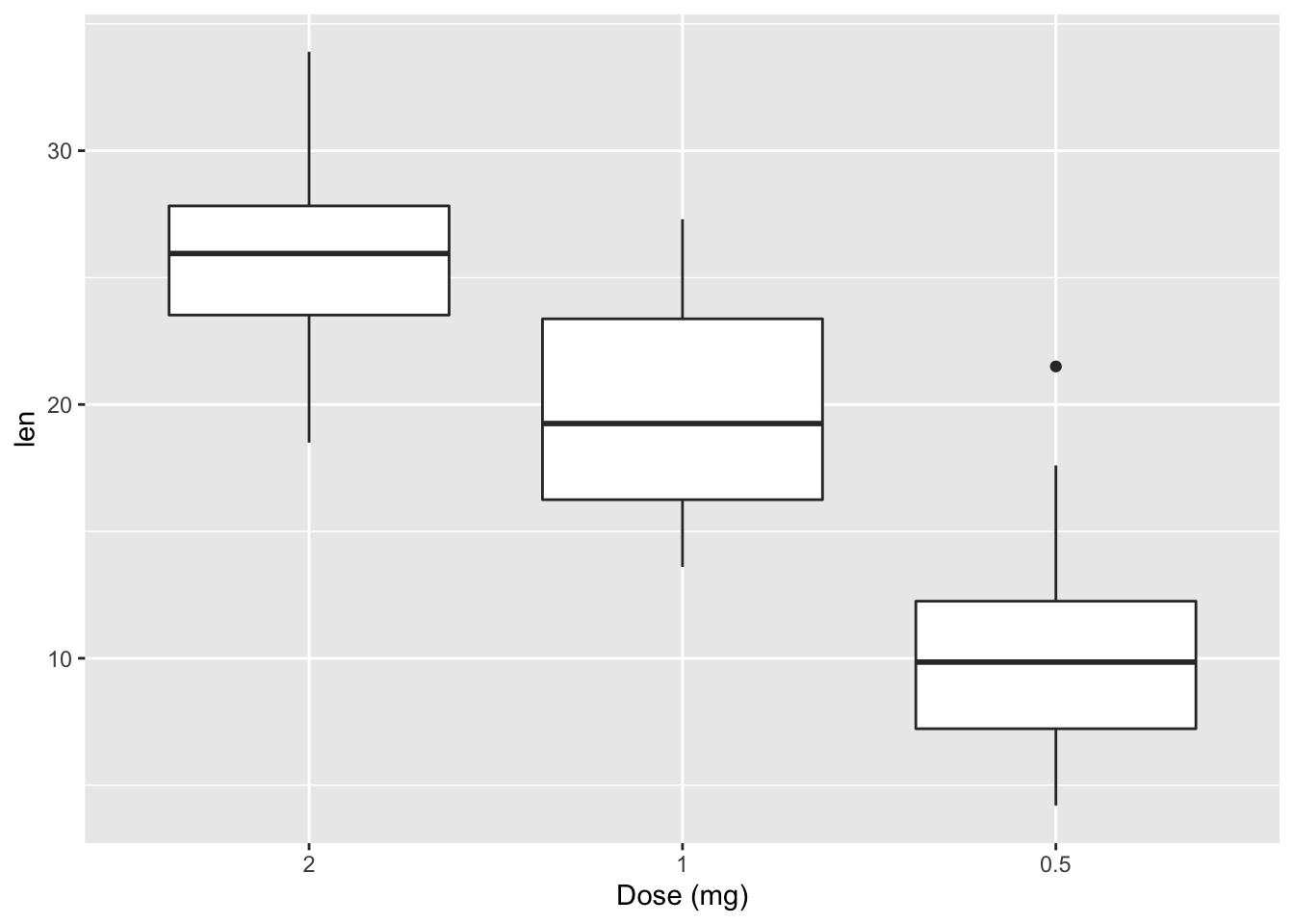### 2.21.2 Change tick mark labels p + scale_x_discrete(breaks=c("0.5","1","2"), labels=c("Dose 0.5", "Dose 1", "Dose 2"))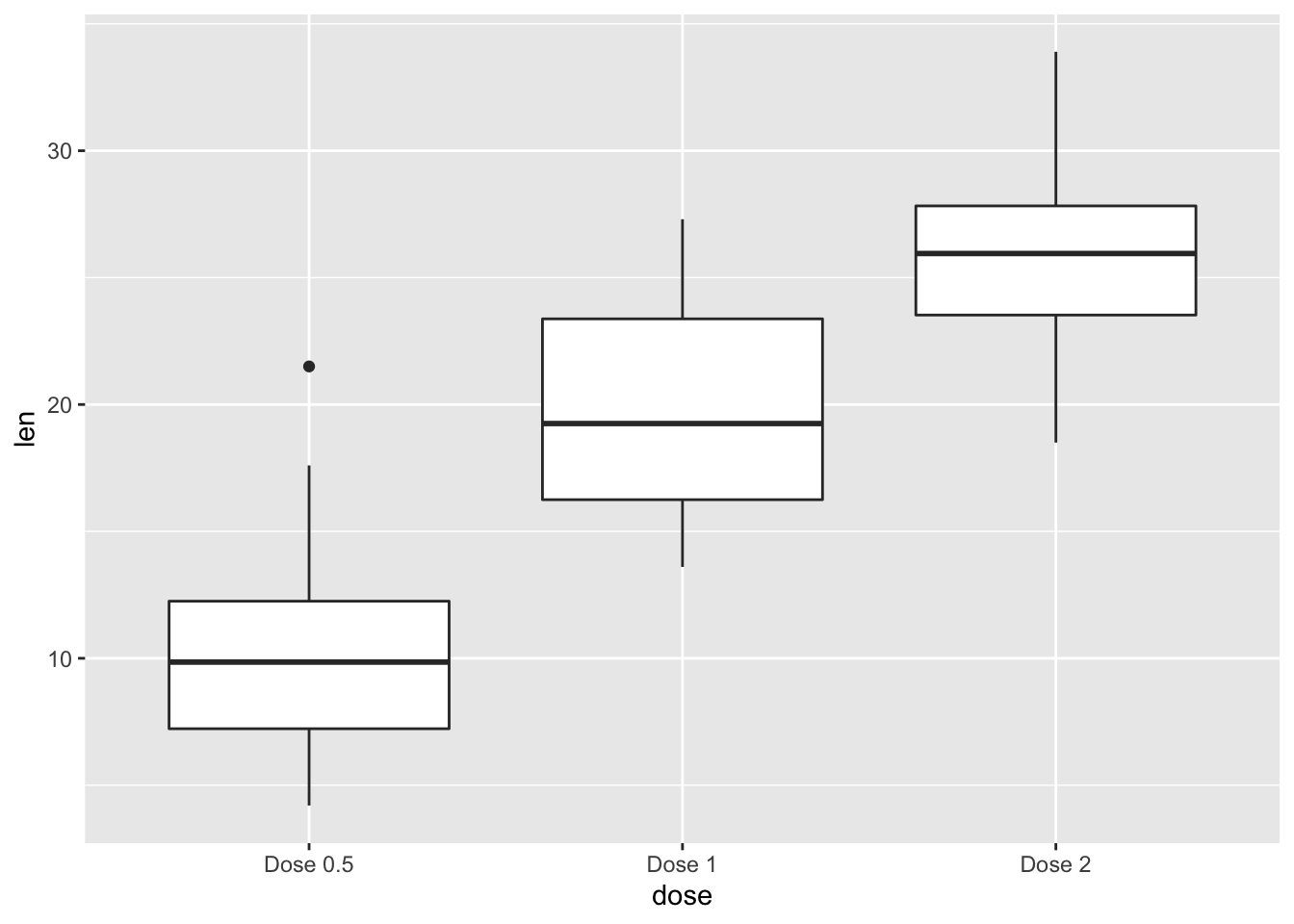### 2.21.3 Choose which items to display p + scale_x_discrete(limits=c("0.5", "2"))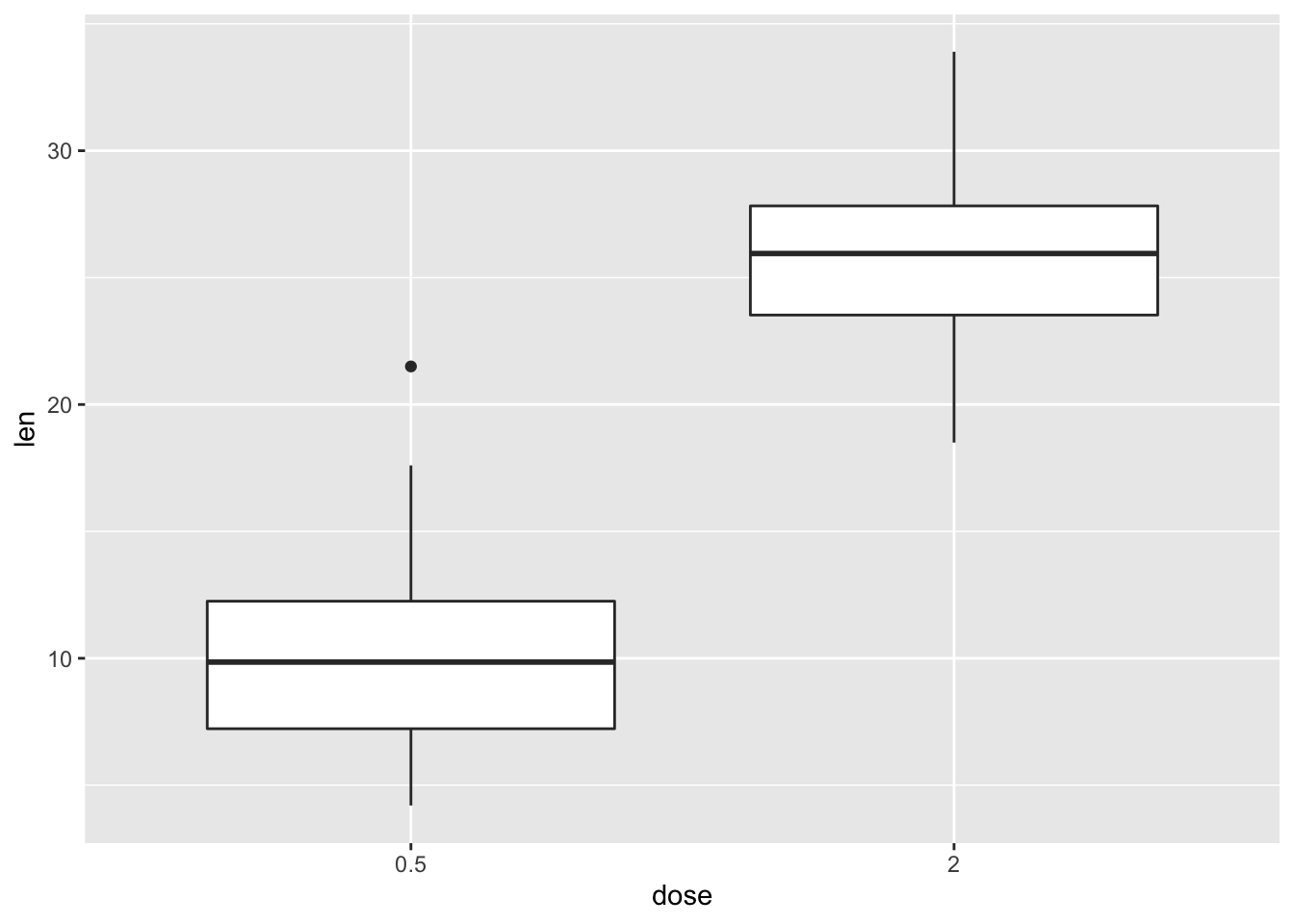### 2.21.4 Continuous axes: ### 2.21.5 Default scatter plot sp <- ggplot(cars, aes(x = speed, y = dist)) + geom_point() sp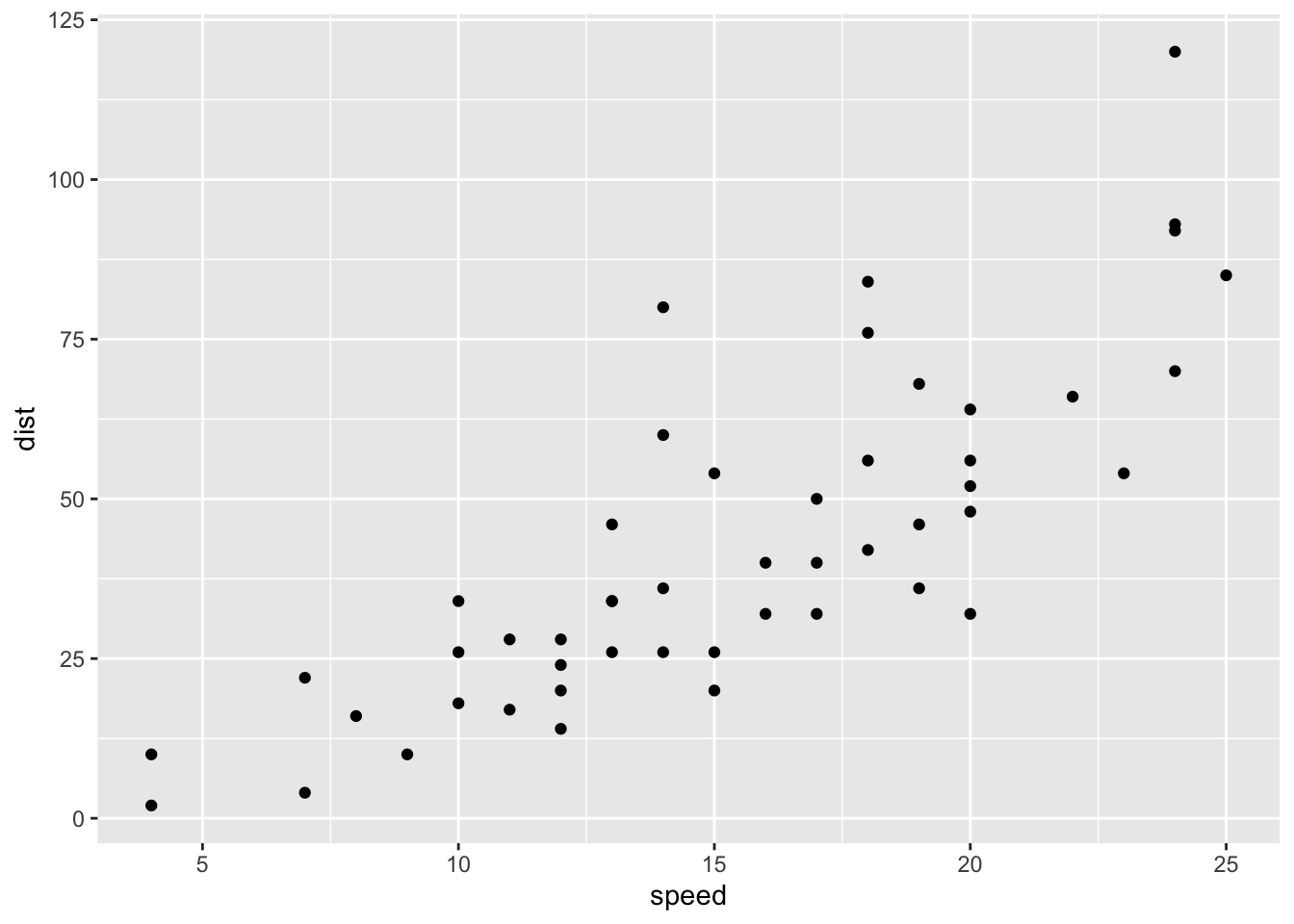## 2.22 Customize the plot ### 2.22.1 1. Change x and y axis labels, and limits sp <- sp + scale_x_continuous(name="Speed of cars", limits=c(0, 30)) + scale_y_continuous(name="Stopping distance", limits=c(0, 150))  ### 2.22.2 2. Set tick marks on y axis: a tick mark is shown on every 50 sp + scale_y_continuous(breaks=seq(0, 150, 50))  ## Scale for 'y' is already present. Adding another scale for 'y', which ## will replace the existing scale.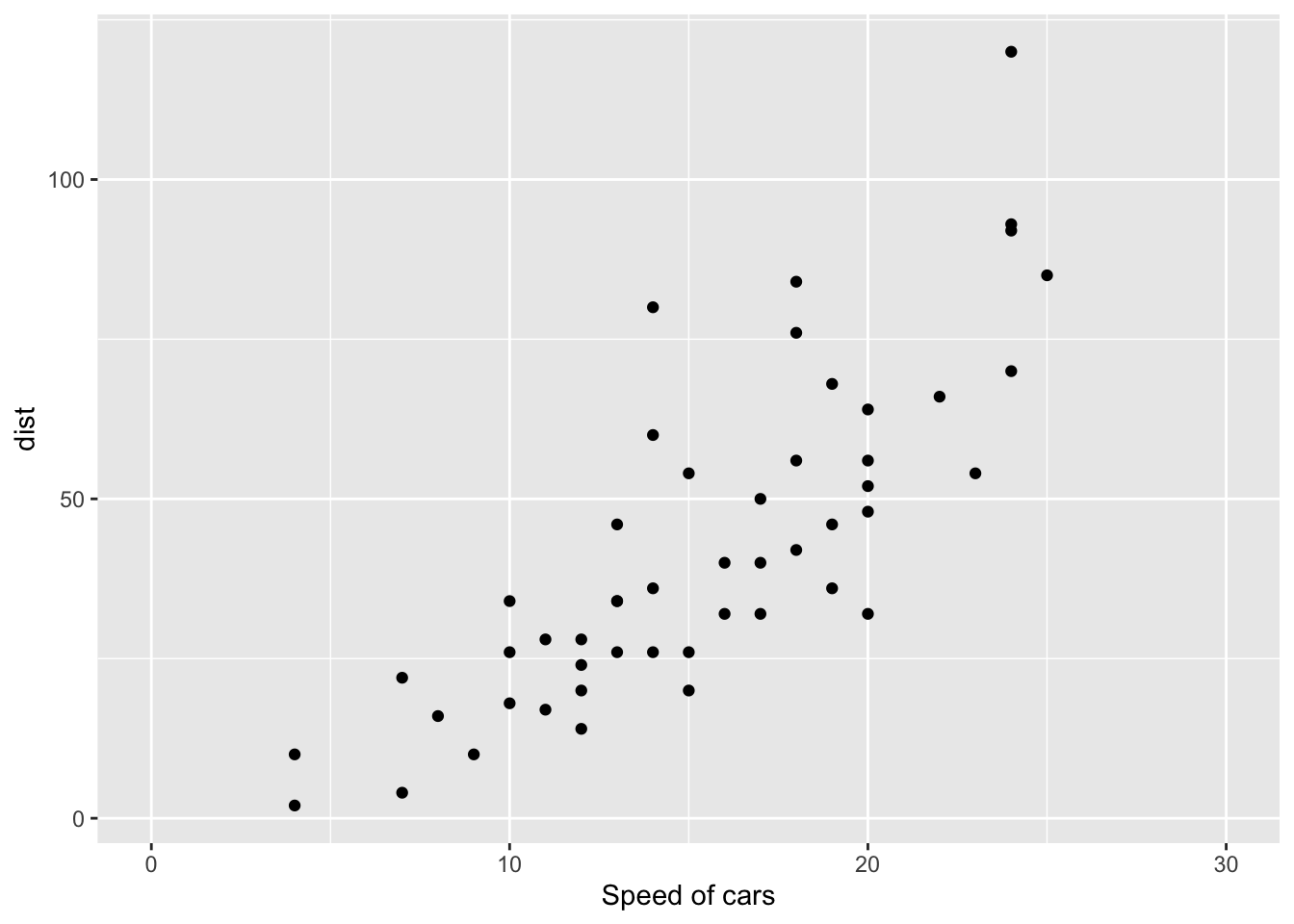### 2.22.3 Format the labels require(scales) sp + scale_y_continuous(labels = percent) # labels as percents ## Scale for 'y' is already present. Adding another scale for 'y', which ## will replace the existing scale.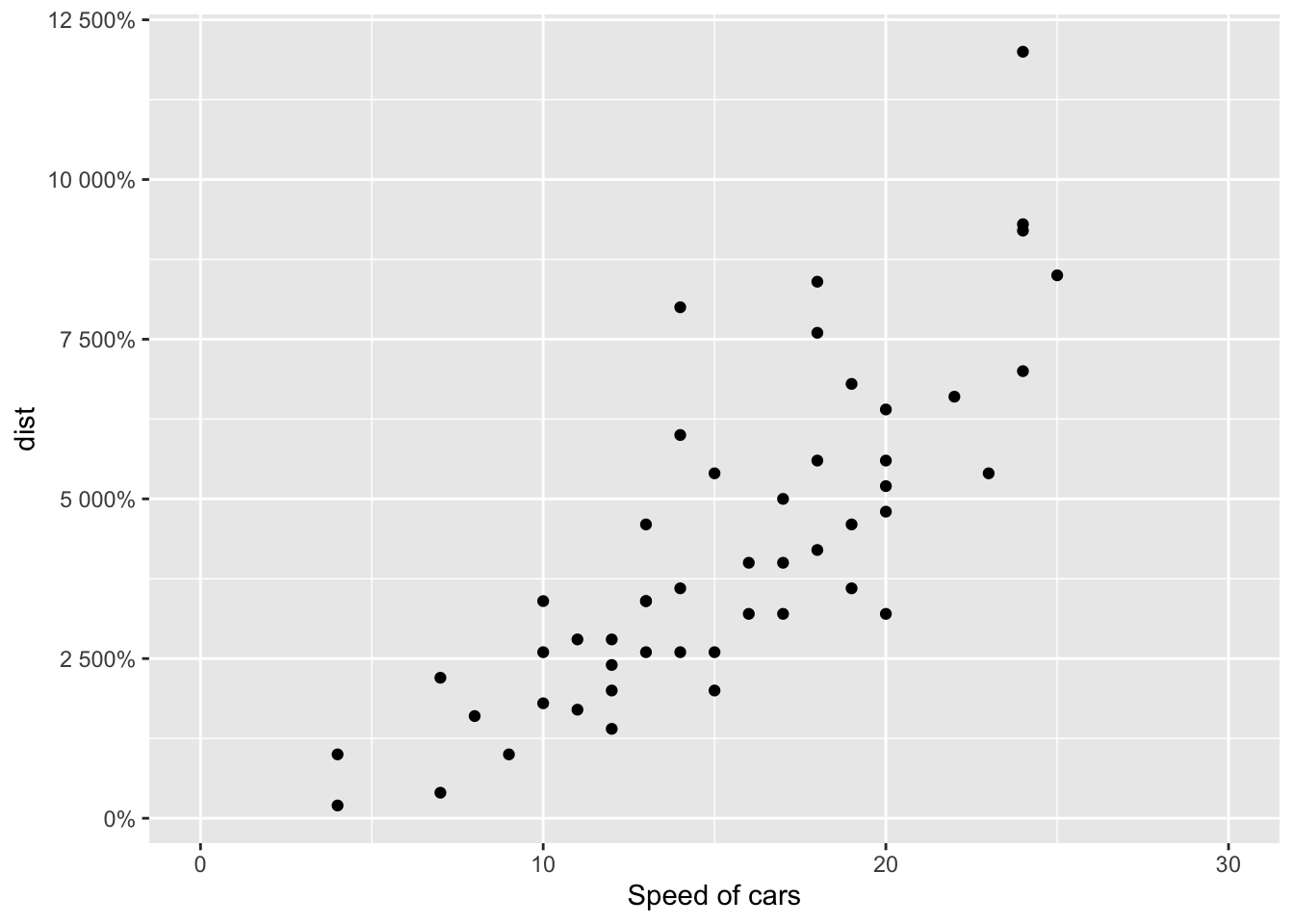## 2.23 Add straight lines to a plot: horizontal, vertical and regression lines ### 2.23.1 Simple scatter plot sp <- ggplot(data=mtcars, aes(x=wt, y=mpg)) + geom_point() ### 2.23.2 Add straight lines ### 2.23.3 Add horizontal line at y = 2O; change line type and color sp + geom_hline(yintercept=20, linetype="dashed", color = "red")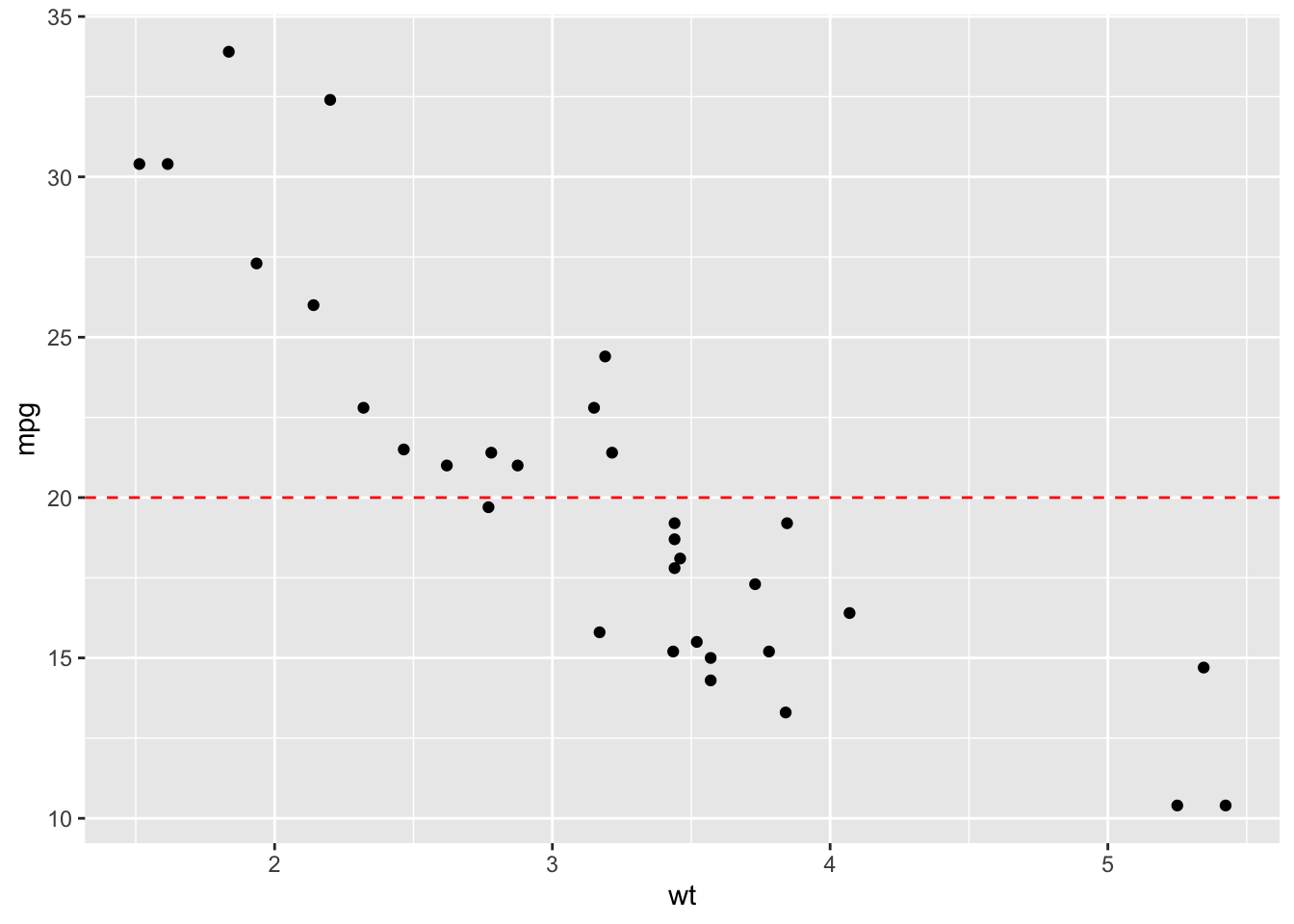## 2.24 Add vertical line at x = 3; change line type, color and size sp + geom_vline(xintercept = 3, color = "blue", size=1.5)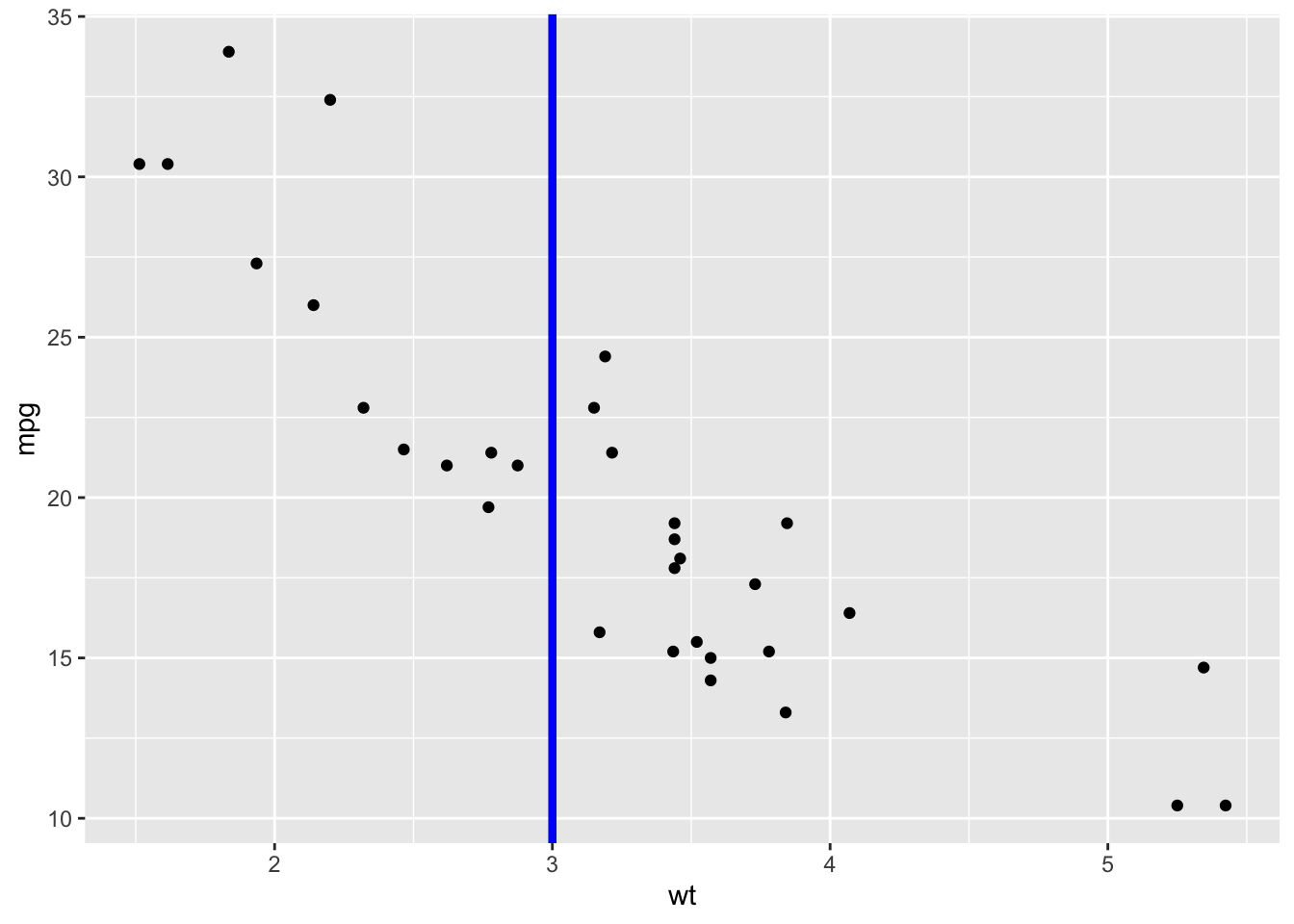## 2.25 Add regression line sp + geom_abline(intercept = 37, slope = -5, color="blue")+ ggtitle("y = -5X + 37")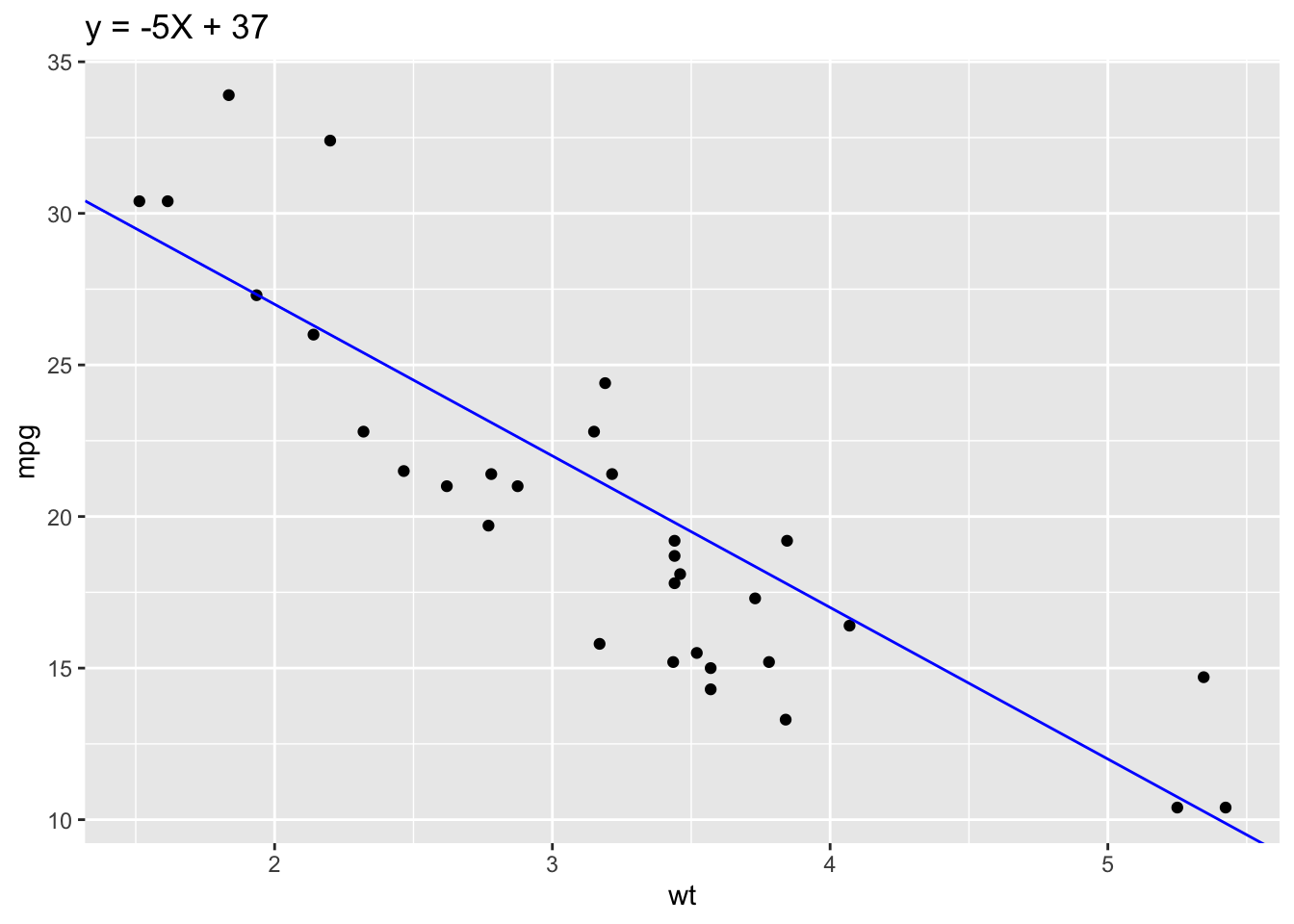## 2.26 Add horizontal line segment sp + geom_segment(aes(x = 2, y = 15, xend = 3, yend = 15))## 2.27 My Data Example Water samples were collected on 6/6/18, 9/24/18, 12/17/18, 1/26/19 and ran on an autoanalyzer to determine NO3-N, NH4-N, PO4, and Chl-a concentrations across 8 water tanks identified by treatments (control "A" or nutrient enriched "N"). Moreover this data was used in my master's thesis. Research was conducted at LSU wetland observation greenhouses in Baton Rouge, LA. ### 2.27.1 Location map LA_counties <- map_data("county", "louisiana") %>% select(lon = long, lat, group, id = subregion) head(LA_counties, n=3) ## lon lat group id ## 1 -92.61863 30.48136 1 acadia ## 2 -92.48685 30.48709 1 acadia ## 3 -92.48685 30.48709 1 acadia ggplot(LA_counties, aes(lon, lat)) + geom_point(size = .25, show.legend = FALSE) + coord_quickmap()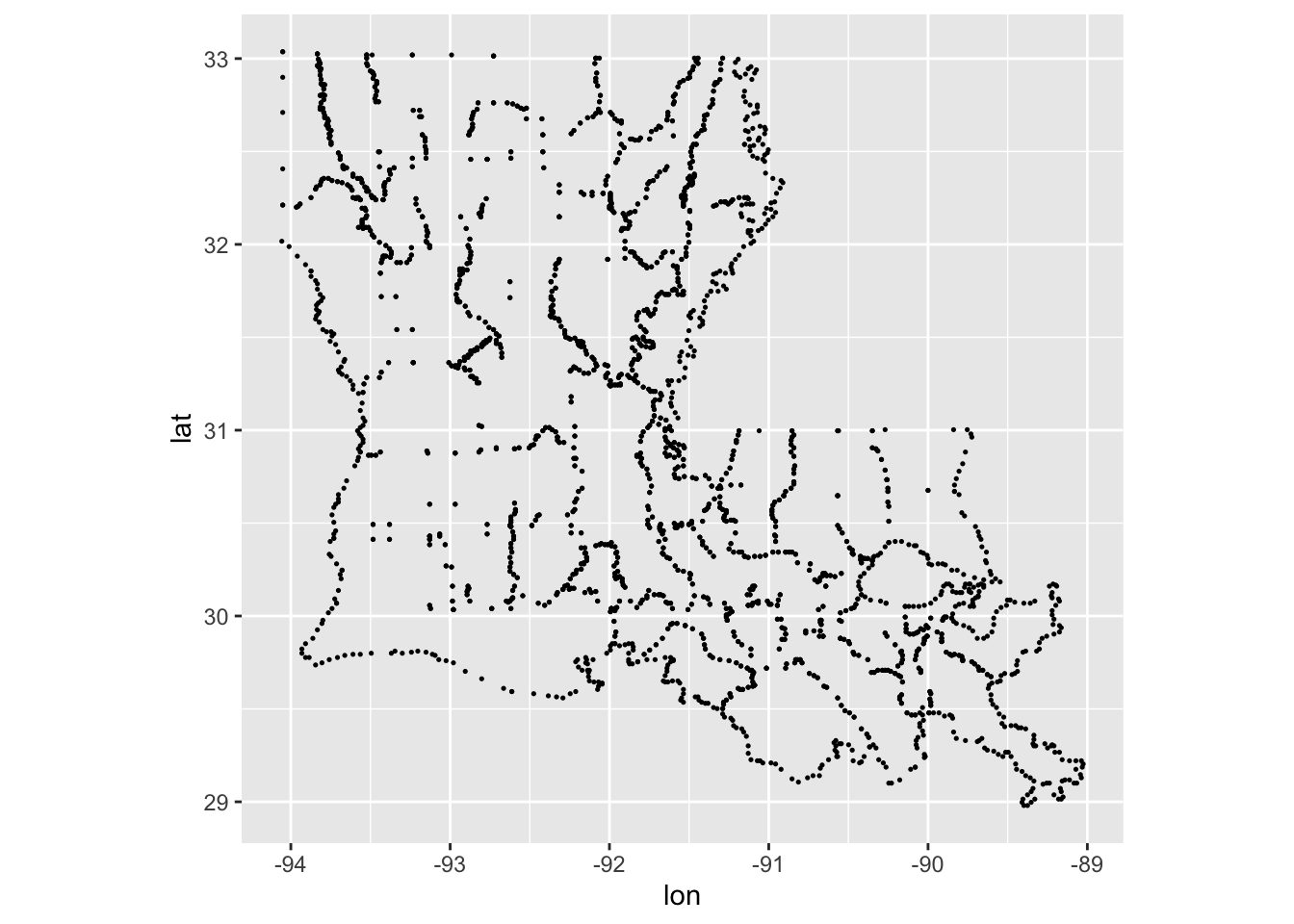ggplot(LA_counties, aes(lon, lat, group = group )) + geom_polygon(fill = "white", colour = "grey50") + geom_point(data = LA_counties, mapping = aes(x = -91.1828669024795, y = 30.4114813833709), colour = "red") + coord_sf()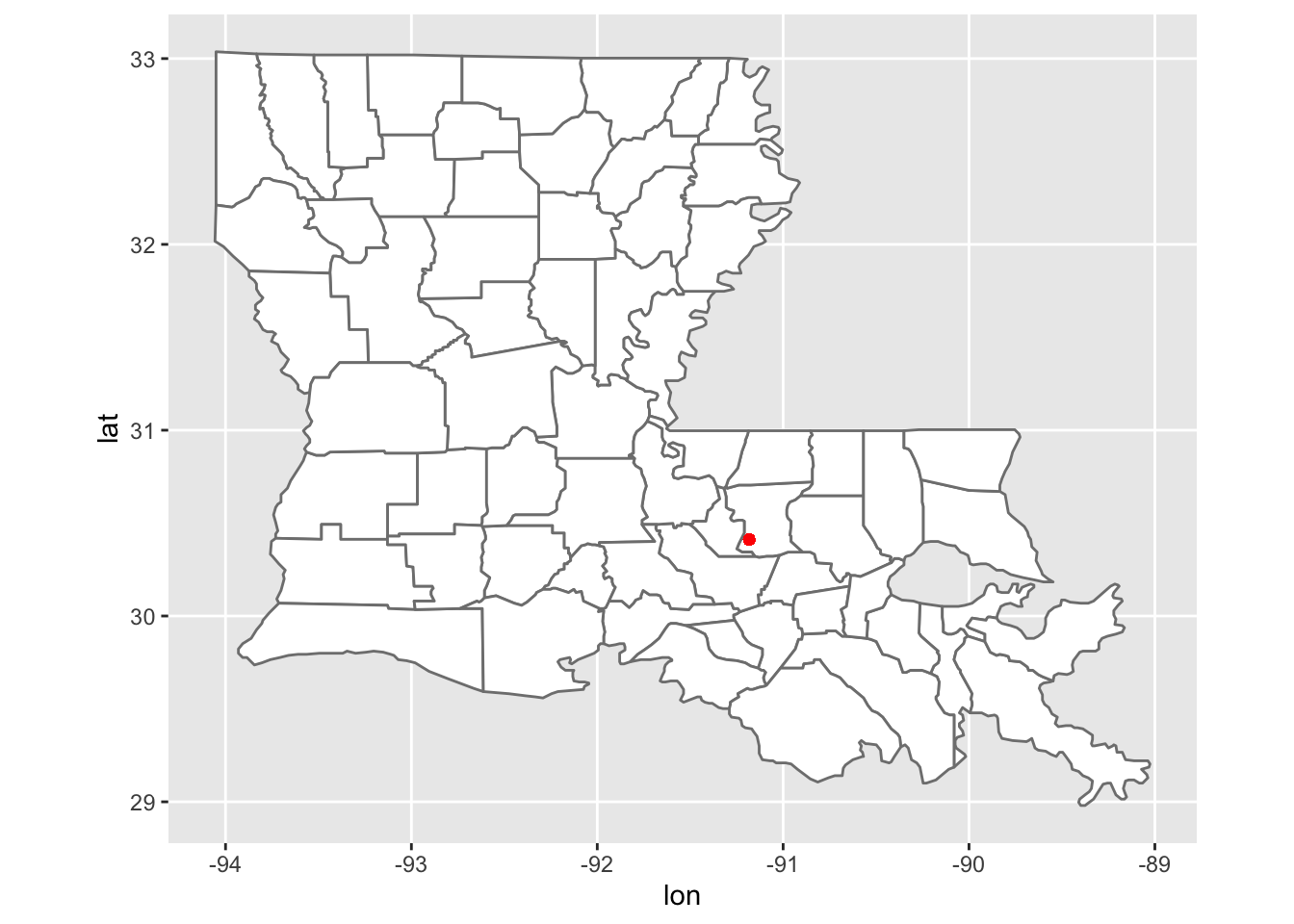Here I made a map that contains the geolocation of our wetland observation greenhouses using ggplot. library(readr) Porewater_data <- read_csv("~/Desktop/R folder/Bookdown/Porewater_data.csv") head(Porewater_data, n=3) ## # A tibble: 3 x 7 ## Tank Date NO3 (uM) NH4 (uM) PO4 (uM) Conc of chl (ug/… Nutrient ## <dbl> <chr> <dbl> <dbl> <dbl> <dbl> <chr> ## 1 1 6/6/18 2.07 1.35 3.08 2.77 A ## 2 2 6/6/18 4.68 2.48 2.45 5.3 N ## 3 3 6/6/18 3.05 1.77 4.62 2.99 A Here I read the porewater dataset in and headed the first three lines ### 2.27.2 Scatter plot ggplot(Porewater_data, aes(x=Conc of chl (ug/L), y=NH4 (uM), group=Nutrient)) + geom_point(aes(shape=Nutrient, color=Nutrient), size=2)+ theme_classic()+ theme(legend.background = element_rect(fill="gray"))+ geom_hline(yintercept=2, linetype="dashed", color = "black")+ geom_abline()+ scale_shape_manual(values=c(3, 16))+ scale_color_manual(values=c('red','blue'))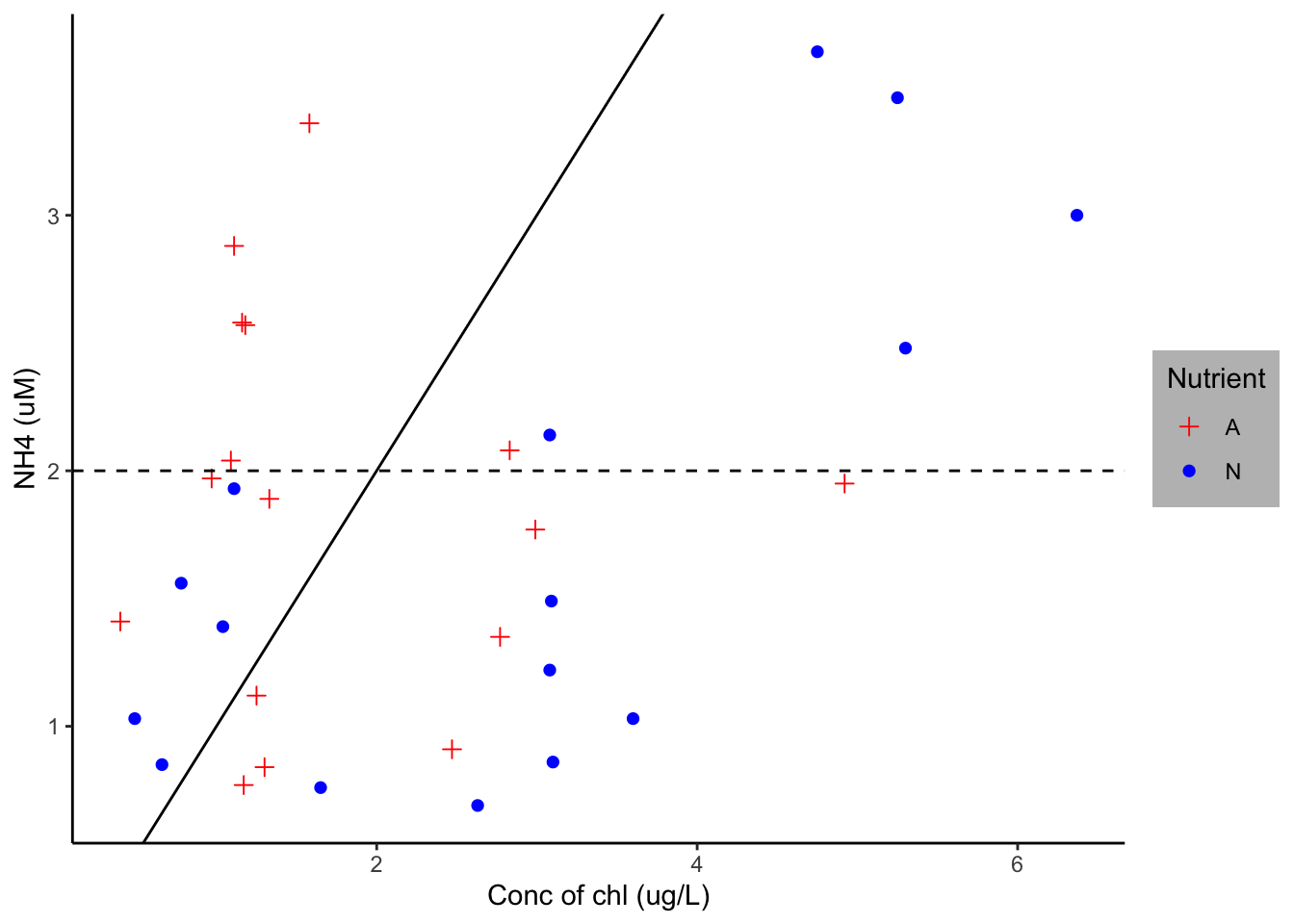From the porewater dataset, I set x as concentration of chl and y as NH4 and grouped them by nutrients. I then distinguished the nutrient treatments using geom_point, which made them different schapes. I removed the lined background using the theme_classic argument. I added a mid-line and abline using the geom_hline and geom_abline argument respectively. I then manually changed the treatment colors to blue and red. ### 2.27.3 Scatterplot by date SamplePoint<-factor(Porewater_data$Date, levels = c("6/6/18", "9/24/18", "12/17/18", "1/26/19"))
ggplot(Porewater_data, aes(x=SamplePoint, y=NH4 (uM), group=Nutrient)) + geom_point(aes(shape=Nutrient, color=Nutrient), size=2)+ scale_shape_manual(values=c(3, 16))+ scale_color_manual(values=c('red','blue'))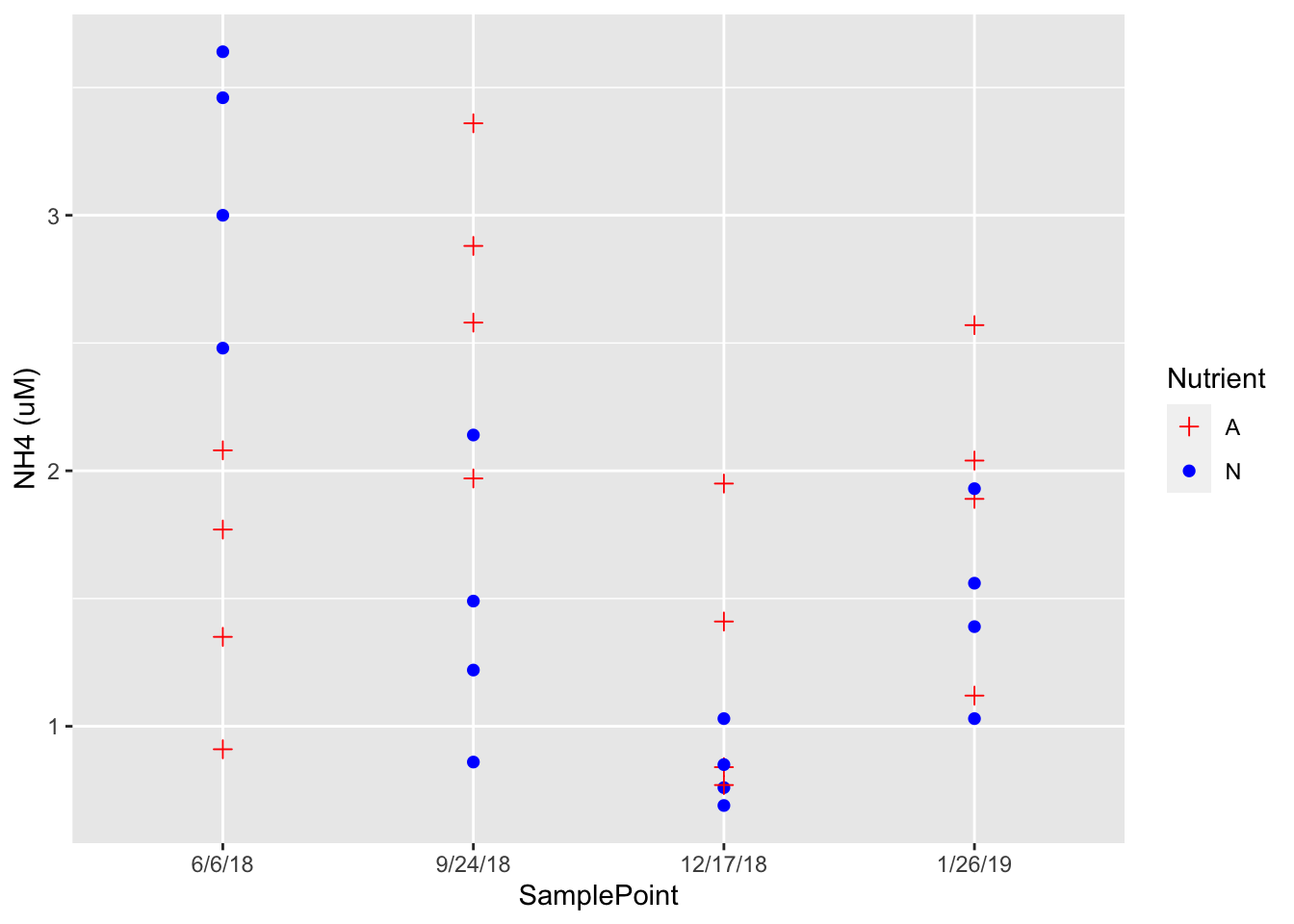First I made the dates a factor in the porewater dataset in order so they would be distributed simillarly in any future graphs, and named it Sample Point. I replace concentration of chl with Sample point for x, removed the theme, abline and halfline. This data show the scatter distribution of NH4 as it relates to nutrient treatment over 4 time points.

### 2.27.4 Boxplot

ggplot(Porewater_data, aes(x=SamplePoint, y=NH4 (uM), fill=Nutrient))+ geom_boxplot()  + theme_classic()+ labs(fill = "Treatment")+ theme(legend.background = element_rect(fill="gray"))`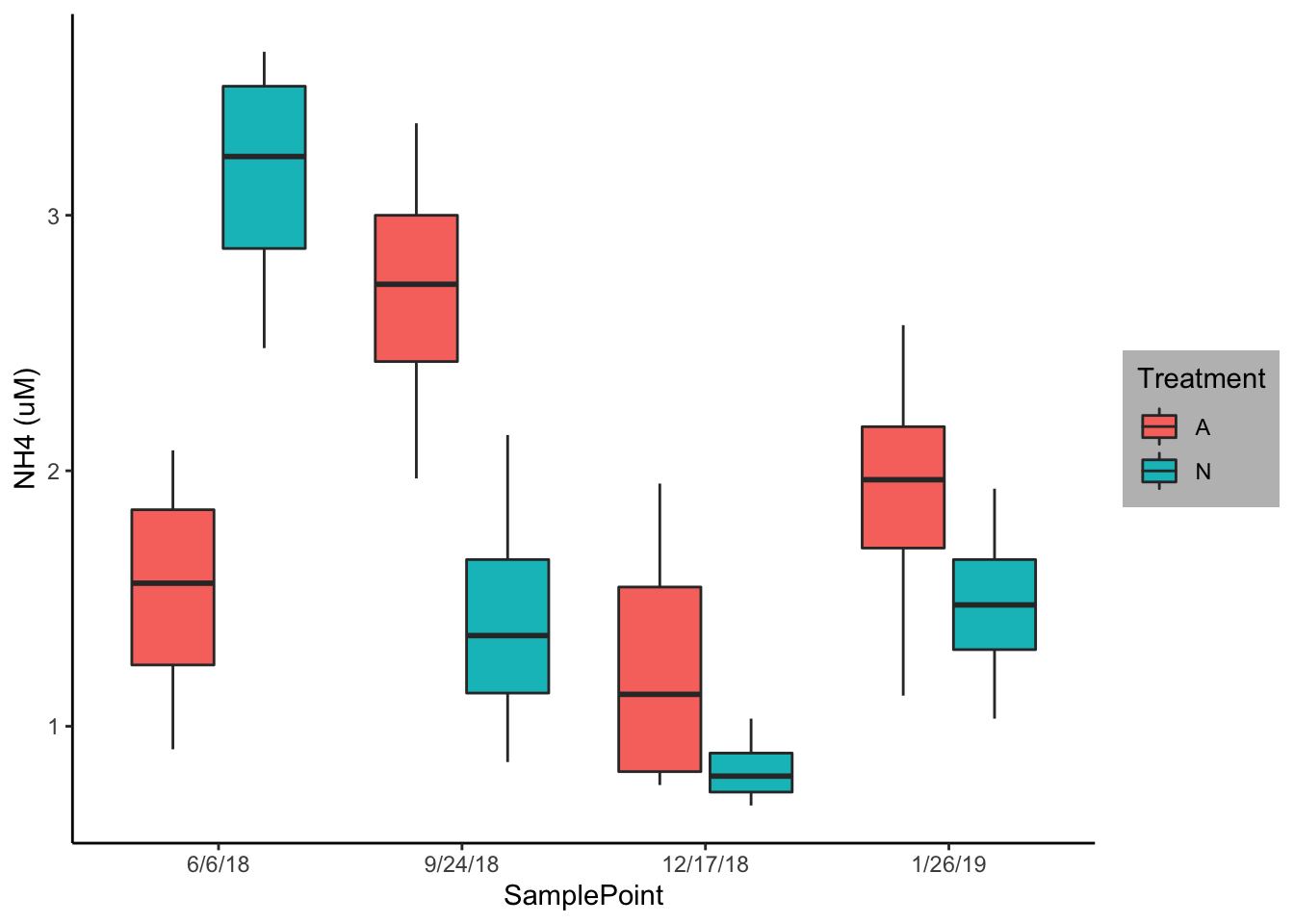I used the same data construction as above but here I created a boxplot using geom_boxplot. I once again removed the background noise using theme_classic and changed the legend title to treatment. I would most likely use this figure to describe any interaction.